2019年06月25日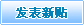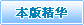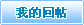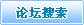本页主题: 〓★〓趣味简述四版四大伟人蓝色100元人民币数字水印与流水号之间关联性。 人气： | 收藏主题 | 上一主题 | 下一主题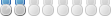*沈万三。就是3和7组合【金8玉5满13堂11划8+5+13+11=37】。【沈7万3三3划7+3+3=13。因为多了一个3（太有财了）】【胜9利7划9+7=16。1+6=7起来=起10来7划10+7=17要起。失5败8划5+8=13。1+3=4=倒10下3划。10+3=13。1+3=4】。元末明初通过开展海外贸易成为江南首富商人，吴兴南浔（今属浙江湖12州6【12+6=18要发配】）人，被朱元璋【朱6元4璋15划。6+4+15=25。2+5=7。少了一个3。从沈万三商人手里拿，抑制商人。另外肃贪财。】发配充军云4南9划。【4+9=13。】。度过了他的余生。如果当时【挪9海10外5划。9+10+5=24。2+4=6顺利。挪9划=活9划。】可以避免发配充军。【商11人2划11+2=13搏13划（搏利）】【大3买6办4划。3+6+4=13搏13划（搏利）。★7柒：是起财，要财，求财，得财，盈利，起业。有进有出。五福。是9一1把7开4启7财7富12之3门3钥9匙11划9+1+7+4+7+7+12+3+3+9+11=73。【芝6麻11划。6+11=17要开启，要财。开4门3划4+3=7】。★3叁：是发财，生财，招财，聚财，搏（取）利。聚拢钱财。多进少出。五位财神。
★ 3和7组合【就是升起=春9风4得11意13划9+4+11+13=37划】【沪7深11股8票11划。7+11+8+11=37财富之门】★3和7组合就是才与财的组合★【是9一1把7开4启7财7富12之3门3钥9匙11划9+1+7+4+7+7+12+3+3+9+11=73】可以【纵7横15四5海10划7+15+5+10=37。】【贫8穷7者8求7财7划8+7+8+7+7=37】【创6造10划6+10=16。1+6=7。才3划+财7划=10圆】【焕11然12一1新13划11+12+1+13=37】【惊11喜12连7连7划11+12+7+7=37】【广3州6纵7原10市5场6划3+6+7+10+5+6=37】【上3海10卢5工3邮7币4卡5划3+10+5+3+7+4+5=37】【绝9路13逢10生5划9+13+10+5=37】【仙5人2指9明8路13划5+2+9+8+13=37】【钱10袋11子3鼓13划10+11+3+13=37】【拿10不4完7，用5不4完7划10+4+7+5+4+7=37】【加5官8进7爵17划5+8+7+17=37】【有6聚14宝8盆9划6+14+8+9=37】【独9占5鳌18头5划9+5+18+5=37】【一1心4想13发5天4下3财7划1+4+13+5+4+3+7=37】【资10源13共6享8划10+13+6+8=37】【故9宫9展10览9划9+9+10+9=37】【现8金8雄12厚9划8+8+12+9=37】【腰13缠13万3贯8划13+13+3+8=37】【金8玉5满13堂11划8+5+13+11=37】【观6音9菩11萨11划6+9+11+11=37。有三十三个化身。三十三观音图，现藏于日本东京国立博物馆。】。
★【多6想13生5财7道12划6+13+5+7+12=43画+广3开4致10富12门3划3+4+10+12+3=32画=75。7+5=12。1+2=3发财】★
★【喜12风4入2喜12财7入2户4划12+4+2+12+7+2+4=43画+岁6月4更7新13福13满13门3划6+4+7+13+13+13+3=59画=102要你爱。1+0+2=3生财】★
★【九2州6鸿11运7进7福12门3，四5海10财7源13聚14宝8地6划2+6+11+7+7+12+3+5+10+7+13+14+8+6=111要要要1+1+1=3。要9要9要9划9+9+9=27爱财】★

万3划。事8划。如6划。意13划。3+8+6+13=30画。
居8划。家10划。快7划。乐5划。8+10+7+5=30画。
热10划。火4划。朝12划。天4划。10+4+12+4=30画。
一1划。路13划。连7划。科9划1+13+7+9=30画。
福13划。寿7划。双4划。全6划。13+7+4+6=30画。
生5划。财7划。有6划。道12划。5+7+6+12=30画。
万3划。商11划。云4划。集12划。3+11+4+12=30画。
招8划。财7划。进7划。宝8划。 8+7+7+8=30画。
财7划。源13划。广3划。进7划。7+13+3+7=30画。
外5划。汇5划。储12划。备8划。5+5+12+8=30画。
上3证7综11指9划3+7+11+9=30画。建行一1触13即7贷9划
1+13+7+9=1+13+7+9=30（快7贷9划7+9=16。1+6=7财）。
求7真10务5实8划7+10+5+8=30。文4明8划4+8=12。1+2=3。
外5贸9展10会6划5+9+10+6=30。3+0=3生财。
人2往8高10处5去5划2+8+10+5+5=30。得11财7富12划11+7+12=30
融16幣14划16+14=30。3+0=3生财。錢16幣14划16+14=30。
上海话：银11洋9钿diàn，tián。10划11+9+10=30。3+0=3。
顺9水4推11舟6划9+4+11+6=30。忠8贞6不4渝12划8+6+4+12=30。
唐10僧14肉6划10+14+6=30。月4宫9仙5子3划4+9+5+3=21。2+1=3。
天4元4太4保9划4+4+4+9=21。无4量12寿7佛7划4+12+7+7=30。
获10利7丰4厚9划10+7+4+9=30。满13堂11红6划13+11+6=30。
如6获10至6宝8划6+10+6+8=30。自6得11其8乐5划6+11+8+5=30。
喜12洋9洋9划 12+9+9=30。刷8微13信9划8+13+9=30。3+0=3
（西南财神）端14木4赐12划14+4+12=30。3+0=3。
貔17貅13划  17+13=30。3+0=3升，盛。进财的。
只5进7不4出5划。5+7+4+5=21。抓7住7财7划7+7+7=21。2+1=3。
拿10薪16水4划10+16+4=30=领11死6工3资10划11+6+3+10=30。
富12划。1+2=3。富12贵9划。12+9=21。雄12厚9划12+9=21。2+1=3。
捞10实8惠12划10+8+12=30。高10质8量12划10+8+12=30。
独9尊12划9+12=21=尊12贵9划12+9=21。2+1=3。
车4载10斗4量12划4+10+4+12=30。横15扫6千3军6划15+6+3+6=30
络9绎8不4绝9划9+8+4+9=30。买6空8卖8空8划6+8+8+8=30。
购8物8卡5划。8+8+5=21。2+1=3。禄12划1+2=3。
几2个3职11业5划2+3+11+5=21。2+1=3。聪15慧15划15+15=30。
祈8福13划。8+13=21。2+1=3。关6羽6划（西）。
6+6=12。1+2=3。贷9款12划。9+12=21。一1路13长4久3划1+13+4+3=21。
房8源13划8+13=21。2+1=3。真10谛11划10+11=21。
圆10梦11划。10+11=21。健10康11划10+11=21。2+1=3。
底8气4十2足7划。8+4+2+7=21.2+1=3钱多。前9程12划9+12=21。
气4壮6山3河8划4+6+3+8=21。心4平5气4和8划4+5+4+8=21。
言7归5于3好6划7+5+3+6=21。晒10一1晒10划10+1+10=21。2+1=3。
钱10多6。10+6=16。1+6=7财。铜11钱10划11+10=21。2+1=3。
月财神赵9公4明8划。9+4+8=21。幸8福13划8+13=21。2+1=3。
玄5天4上3帝9划4+5+3+9=21。风4云4际7会6划4+4+7+6=21。
天4官8天4仙5划4+8+4+5=21。吉6人2天4相9划6+2+4+9=21。
富12饶9划12+9=21。2+1=3。获10得11划10+11=21。2+1=3。
道教玄5坛7元4帅5划5+7+4+5=21。2+1=3。焦12点9划12+9=21。
三3十2三3重9天4划3+2+3+9+4=21。和8合6二2仙5划8+6+2+5=21。
祈8福13划8+13=21。2+1=3。财7气4冲6天4划7+4+6+4=21。
飞3神9划3+9=12。1+2=3。命8长4划8+4=12。1+2=3生。
结9缘12划9+12=21。2+1=3。总9裁12划9+12=21。2+1=3。
秋9水4伊6人2划9+4+6+2=21。井4井4有6条7划4+4+6+7=21。
管14财7划。14+7=21。2+1=3。理11钱10划11+10=21。2+1=3。
发5财7树9划5+7+9=21。2+1=3。饱8满13划8+13=21。2+1=3。
金8像13划8+13=21。试8金8石58+8+5=21。2+1=3。
股8权6证7划8+6+7=21。2+1=3。揽12活9划12+9=21。2+1=3。
进7库7里7划7+7+7=21.2+1=3.和8睦13划8+13=21。2+1=3
剩12划。1+2=3。回6扣6划6+6=12。1+2=3。能10力2划10+2=12。
日4升4昌8记5划4+4+8+5=21。田5黄11石5划5+11+5=21。2+1=3。
赚14钱10妙7招8划14+10+7+8=39。3+9=12。1+2=3。
有6钱10是9任6性8划6+10+9+6+8=39。3+9=12。1+2=3。
上3帝9划3+9=12。1+2=3。圣5灵7划5+7=12。1+2=3。
圣5诞8节5快7乐5划5+8+5+7+5=30。快7乐5划7+5=12。1+2=3。
未5来7划5+7=12。1+2=3。奋8斗4划8+4=12。1+2=3。
中4国8人2寿7划4+8+2+7=21。中4国8保9险9划4+8+9+9=30。
中4国8石5化4划4+8+5+4=21。万3事8通10划。3+8+10=21。2+1=3。
百6战9百6胜9划6+9+6+9=30【不4胜9枚8举9划4+9+8+9=30】
【知8己3知8彼8划8+3+8+8=27爱才+百6战9百6胜9划
6+9+6+9=30】=57我胜利（胜9利7划9+7=16。1+6=7）5+7=12。
出5纳7划。5+7=12。1+2=3生财。宝8贝4划8+4=12。1+2=3。
北5京8马3甸7邮7币4卡5划5+8+3+7+7+4+5=39。+9=12。1+2=3。【★恭10喜12发5财7划。10+12+5+7=34立于3市场4。谐音升势。盛世。3+4=7】【求7划。财7划。迎7划。利7划。来7划。吞7。进7划。库7划。里7划。余7划。佛7划。秀7划。应7划。快7划。你7我7划。时7间7划。身7体7划】【盈9划，利7划。9+7=16画，一路顺。1+6=7财，来7划（来财）】【进7划（进财）运7划（财运）。足7划（财足）】【有6钱10划。6+10=16。1+6=7财】【转8账8划8+8=16。1+6=7财】【走7鸿11运7划7+11+7=25。2+5=7财】【大3户4划3+4=7财】【寿7划】【吉6祥10划6+10=16。1+6=7利。白5象11划5+11=16。1+6=7。长4久3划。4+3=7财】【禧16划一路顺1+6=7财】【胜9利7划9+7=16。1+6=7财】【活9财7划9+7=16。1+6=7财】【涨10价6划10+6=16。1+6=7财】【高10价6划10+6=16。1+6=7财】启7划开启财源之门。★【龍16划。1+6=7。腾13划，飞3划13+3=16。1+6=7。谐音：起】【活9财7神9划.9+7+9=25。2+5=7。财7神9划7+9=16一路顺。1+6=7财。修9财7划。9+7=16。1+6=7妙。显9灵7划9+7=16。1+6=7妙】【有6求7必5应7划6+7+5+7=25。2+5=7财 】【金8银11财7宝8划8+11+7+8=34。3+4=7财】【金8蟾19划（又称三足金蟾。招8财7蟾19划。8+7+19=34。3+4=7财。）8+19=27爱财】【富12甲5全6球11划12+5+6+11=34盛世。3+4=7财】【金8融16外5汇5划8+16+5+5=34。3+4=7财】【保9佑7划。9+7=16。1+6=7利。27+16=43。4+3=7财】【福13禄12划。13+12=25。2+5=7财】【财神：比4干3划（东）4+3=7。】【算14盘11划14+11=25。2+5=7财】清朝广州的世界商人首富伍秉鉴【伍6秉8鉴13划。6+8+13=27爱财】【首9屈8一1指9划9+8+1+9=27爱财】【清朝末年山西祁县乔致庸（乔6致10庸11划）6+10+11=27爱财，活了89岁8+9=17要财】【壮6志7凌10云4划6+7+10+4=27爱财】【钦9差9大3臣6划9+9+3+6=27爱财】【锐12不4可5当6划12+4+5+6=27】【无4本5生5意13划4+5+5+13=27】【进7账7丰4厚9划7+7+4+9=27爱财】【大3富12大3贵9划3+12+3+9=27爱财】【鱼8跃11龙5门3划8+11+5+3=27爱才】【保9险9公4司5划。9+9+4+5=27爱财】【几2块7洋9钿diàn，tián.10划（几块钱）2+7+9+10=27爱财（出自银11洋9钿10划11+9+10=30。3+0=3生财）】【宝8贵9划8+9=17】【伟6业5腾13飞3划6+5+13+3=27爱财】【暴15富12划。15+12=27爱财】【盛11气4凌10人2划11+4+10+2=27】【宝8葫12芦7划。8+12+7=27爱财】【头5彩11+红6包5划=27爱财】【打5开4幸8运7门3划5+4+8+7+3=27爱财】【名6正5言7顺9划6+5+7+9=27】【万3能10力2量12划3+10+2+12=27启】【起10死6回6生5划10+6+6+5=27希】【冒9青8烟10划9+8+10=27。显9荣9贵9划9+9+9=27】【日4新13月4异6划4+13+4+6=27】【国8色6天4香9划8+6+4+9=27丽】【宾10至6如6归5划。10+6+6+5=27爱迎】【三3十2六4计4，走7为4上3划3+2+4+4+7+4+3=27】【同6舟6共6济9划6+6+6+9=27】【相9安6无4事8划9+6+4+8=27玖】【知8己3知8彼8划8+3+8+8=27爱才】【要9出5人2头5地6划9+5+2+5+6=27爱财】【灵7丹4妙7药9划7+4+7+9=27爱材】【他5项9权6证7划5+9+6+7=27爱财】【财7政9划。7+9=16。1+6=7财。钱10庄6划。10+6=16。1+6=7财。银11号5划。11+5=16。1+6=7财。银11行6划。11+6=17要财。光6票11划。6+11=17银11划（银行用）。汇5票11划5+11=16。1+6=7财】【社7保9划7+9=16。1+6=7财】【一1卡5通10划1+5+10=16。一路顺。1+6=7更7划。快7划】【商11业5划。11+5=16.1+6=7财】【资10本5家10划。10+5+10=25。2+5=7财】【特10权6划10+6=16。1+6=7狂】【联12手4划12+4=16。1+6=7财】【储12蓄13划12+13=25。2+5=7财。存6钱10划6+10=16。1+6=7财】【一1本5万3利7划1+5+3+7=16。1+6=7财】【官8钱10局7划8+10+7=25。2+5=7财】【一1马3当6先6划1+3+6+6=16。1+6=7快】【赐12福13划12+13=25。2+5=7纳】【喜12鹊13划12+13=25。2+5=7告】【收6获10划6+10=16。1+6=7财】【天4降8好6运7划4+8+6+7=25。2+5=7利】【增15益10划15+10=25。2+5=7利】【持9之3以4恒9划9+3+4+9=25。2+5=7玖】【翻18身7划18+7=25。2+5=7财】【辉12煌13划12+13=25。2+5=7玖】【一1顺9百6顺9划1+9+6+9=25。2+5=7财】【门3庭9若8市5划3+9+8+5=25。2+5=7迎】【兴6旺8发5达6划6+8+5+6=25。2+5=7财】【永5久3昌8盛11财5+3+8+11=27爱启】【花7好6月4圆10划7+6+4+10=27求】【行6大3运7划6+3+7=16。1+6=7财】【独9一1无4二2划9+1+4+2=16。1+6=7财】【税12务5划12+5=17要财】【兑7换10划7+10=17要财】【金8银11财7宝8划8+11+7+8=34。3+4=7财】。【金8店8划8+8=16。1+6=7财】【真10金8不4怕8火4炼9划10+8+4+8+4+9=43。4+3=7财】【锦13上3添11花7划13+3+11+7=34。3+4=7丽】【赚14钱10方4式6划14+10+4+6=34。3+4=7财】【挣9钱10妙7招8划9+10+7+8=34。3+4=7财】【排11山3倒10海10划11+3+10+10=34。3+4=7起】【发5财7致10富12划5+7+10+12=34。3+4=7财】【满13载10而6归5划13+10+6+5=34。3+4=7财】【财7库7高10涨10划7+7+10+10=34。3+4=7财】【毕6恭10毕6敬12划6+10+6+12=34。3+4=7】【做11发5财7梦11划11+5+7+11=34。3+4=7财】【太4阳6出5来7喽12划4+6+5+7+12=34。3+4=7迎。晒10一1晒10划10+1+10=21。2+1=3。】【光6辉12灿7烂9划6+12+7+9=34。3+4=7财】【饿10了2要9吃6饭7划10+2+9+6+7=34。3+4=7纳财】【兴6盛11划6+11=17有财】【★你7不4理11会6财7，财7不4理11会6你7划7+4+11+6+7+7+4+11+6+7=70。7+0=7财★】【家10业5昌8隆11划10+5+8+11=34。3+4=7财】【真10金8白5银11划10+8+5+11=34。3+4=7财】【金8字6招8牌12划8+6+8+12=34。3+4=7财】【生5意13景12气4划5+13+12+4=34。3+4=7财】【乘10风4破10浪10划10+4+10+10=34。3+4=7快】【嘴16壮6吃6八2方4划16+6+6+2+4=34。3+4=7财】【商11路13通10划11+13+10=34。3+4=7财】【钻10石5翡14翠14划10+5+14+14=43。4+3=7财】。【胜9利7划9+7=16。1+6=7起来=起10来7划10+7=17要起。失5败8划5+8=13。1+3=4=倒10下3划。10+3=13。1+3=4】【找7机6遇12划7+6+12=25。2+5=7利】【薪16划。1+6=7财】【美9股8划。9+8=17要财】【筹13款12划。13+12=25。2+5=7财】【福13禄12划13+12=25。2+5=7财】【职11业5划11+5=16。1+6=7财】【学8业5有6成6划8+5+6+6=25。2+5=7才】【状7元4及3第11划7+4+3+11=25。2+5=7才】【好6前9途10划6+9+10=25。2+5=7利】【年6年6有6余7划。6+6+6+7=25。2+5=7财】【拥8戴17划8+17=25。2+5=7利】【胸10怀7大3志7划10+7+3+7=27】【奖9学8金8划。9+8+8=25。2+5=7财】【柒9上3捌10下3划。9+3+10+3=25。2+5=7起。谐音（起上趴下）降8划。贫8划。（不4会6划6算14一1世5贫8划4+6+5+14+1+5+8=44。4+4=8扒）（贫8讲6就12划8+6+12=26。2+6=8趴）（算14盘11珠10子3不4饶9人2划14+11+10+3+4+9+2=53。5+3=8扒】。奖9利7划9+7=16.1+6=7财。年6钱10划。6+10=16。1+6=7财【会6计4师6划6+4+6=16。1+6=7财】【内4销12划4+12=16。1+6=7财】【丰4富12划4+12=16。1+6=7财】【赶10场6划10+6=16。1+6=7财】【官8爵17划8+17=25。2+5=7求】【指9日4高10升4划9+4+10+4=27爱才】【海10阔12天4空8划10+12+4+8=34。3+4=7启】【收6钱10划6+10=16。1+6=7财】【出5售11划5+11=16。1+6=7】【放8货8划8+8=16。1+6=7财】【定8金8划划8+8=16。1+6=7财】【不4动6产6划4+6+6=16。1+6=7财】【金8鱼8划8+8=16。1+6=7财】【经8纪6人2划8+6+2=16。1+6=7财】【管14理11划。14+11=25。2+5=7财】赢17划（要来财）【挣9财7划。9+7=16。1+6=7利】【创6造10划6+10=16。1+6=7才，财】【猎11头5划11+5=16。1+6=7人才】【兼10并6划。10+6=16。1+6=7纳】【提12升4划12+4=16。1+6=7努】【彩11礼5划11+5=16。1+6=7利】【东5南9西6北5划。5+9+6+5=25。2+5=7财】【相9助7划9+7=16。1+6=7利】【贸9易8划9+8=17要财】【支4付5宝8划4+5+8=17要财】【执6著11划。6+11=17】【集12市5划。12+5=17搏（取）利】【开4源13划4+13=17。利7益10划。7+10=17】【开4工3划4+3=7启财】【开4业5吉6祥10划4+5+6+10=25。2+5=7财】【竟11争6划11+6=17要财】【奖9金8划。9+8=17要来财。分4红6利7划。4+6+7=17赡17划】【无4与3伦6比4划4+3+6+4=17有财】【现8钞9划8+9=17】【白5金8币4划5+8+4=17】【担8保9划8+9=17要财】【房8贷9划。8+9=17要9还7划。9+7=16.1+6=7利】【投7资10划。7+10=17要财。存6银11划6+11=17生利。计4数13划。4+13=17理钱】【清11算14划11+14=25。2+5=7核实】【地6产6业5划。6+6+5=17要财】【随11行6划11+6=17得财】【好6市5场6划。6+5+6=17赢。好6行6业5划。6+6+5=17赢】商11行6划。11+6=17要财。超12市5划12+5=17要财【通10天4下3划。10+4+3=17赢17画】【利7润10划7+10=17要财】【芝6麻11划。6+11=17要开启，要财。开4门3划4+3=7财】【养9老6颐13年6划9+6+13+6=34。3+4=7财】【钱10串7划10+7=17=多6钱10划6+10=16。1+6=7财】【源13远7流10长4划13+7+10+4=34。3+4=7财】【官8运7亨7通10，连7升4三3级6划8+7+7+10+7+4+3+6=52。5+2=7快7划】【丰4富12划4+12=16。1+6=7多彩。多6彩11划6+11=17】【神9通10广3大3划9+10+3+3=25。2+5=7财】【年6年6好6运7划6+6+6+7=25。2+5=7起来】【会6通10古5今4划6+10+5+4=25。2+5=7财】【事8业5有6成6划8+5+6+6=25。2+5=7财】【汇5川3纳7海10划5+3+7+10=25。2+5=7财】【吉6庆6有6余7划6+6+6+7=25。2+5=7财】【起10步7划10+7=17=起10财7划10+7=17】【周8济9划8+9=17有益。有6益10划6+10=16。1+6=7助7划】【齐6白5石5划6+5+5=16。1+6=7妙7划=美9人2归5划9+2+5=16。1+6=7妍7划】【金8杯8划8+8=16。1+6=7财】【老6婆11划6+11=17妻。妻8妾8划8+8=16。1+6=7】【土3豪14划。3+14=17】【结9账8。9+8=17要财】【千3方4百6计4划3+4+6+4=17要财】【瑞13气4划13+4=17赢。兴6隆11划6+11=17赢。喜12讯5划12+5=17赢。喜12乐5划12+5=17】【全6职11划6+11=17】【孤8品9划8+9=17要财】【贴9金8划9+8=17要财】【趸10批7划10+=7=17要财】【挂9单8划9+8=17要财】【集12市5划12+5=17要财】【计4酬13划4+13=17要财】【甲5等12划5+12=17要财】【内4幕13划4+13=17要财】【挖9货8划9+8=17要财】【企6望11划6+11=17要财】【索10财7划10+7=17要财】【外5销12划5+12=17要财】【投7资10划7+10=17要财】【平5等12划5+12=17戴】【明8星9划8+9=17瞧】首9饰8划9+8=17戴【古5董12划5+12=17要财。古5文4物8划5+4+8=17藏17划】【美9味8划9+8=17宜。悦10耳6划10+6=16。1+6=7宜】【商11务5划11+5=16。1+6=7财】【客9运7划9+7=16。1+6=7迎7划】【经8典8划8+8=16。1+6=7妙】【保9护7划9+7=16。1+6=7助】【走7后6门3划7+6+3=16。1+6=7利】【九2条7命8划2+7+8=17赡17划】【公4路13划4+13=17运财。航10运7划10+7=17。海10运7划10+7=17藏17划钱（有利）。飞3机6运7划3+6+7=16。1+6=7起财。地6铁10划。6+10=16。1+6=7财】【绿11灯6划11+6=17要通行。通10行6划10+6=16。1+6=7启。通10过6划10+6=16.1+6=7】【便9利7划9+7=16。1+6=7】【得11寸3进7尺4划11+3+7+4=25。2+5=7财】【久3久3平5安6划。3+3+5+6=17】【万3象11更7新13划3+11+7+13=34。3+4=7财】【引4人2入2胜9划4+2+2+9=17赢】【实8诚8划8+8=16。1+6=7利。诚8信9划。8+9=17得财】【别7开4生5面9划7+4+5+9=25。2+5=7】【神9通10广3大3划9+10+3+3=25。2+5=7才】【先6入2为4主5划6+2+4+5=17】【五4花7八2门3划4+7+2+3=16。1+6=7财】【气4宇6轩7昂8划4+6+7+8=25。2+5=7才】【运7筹13帷11幄12划7+13+11+12=43。4+3=7盈】。【快7贷9划7+9=16。1+6=7财】【有6机6可5承8划6+6+5+8=25。2+5=7乱】【一1鼓13作7气4划1+13+7+4=25。2+5=7快】【火4红6的8日4子3划4+6+8+4+3=25。2+5=7步】【迎7头5赶10上3划7+5+10+3=25。2+5=7快】【中4国8石5油8划4+8+5+8=25。2+5=7财】【避16险9划16+9=25。2+5=7财】【诸10葛12孔4明8划10+12+4+8=34。3+4=7才】【雷13公4划13+4=17狂。重9金8划9+8=17要财】【驱7邪6降8福13划7+6+8+13=34。3+4=7贡】【有6钱10能10使8鬼9推11磨16划6+10+10+8+9+11+16=70。7+0=7财】【金8本5位7划+银11本5位7划=43。4+3=7财】【不4愁13吃6喝12，不4愁13穿9划4+13+6+12+4+13+9=61。6+1=7财】【贵9金8属12交6易8划9+8+12+6+8=43。4+3=7财。钌7、铑11、钯9、锇12、铱11、铂10、黄11金8划7+11+9+12+11+10+11+8=79。7+9=16。1+6=7财】【一1尘6划一路顺1+6=7起财】【电5力2划5+2=7财】【七2颗14宝8珠10划2+14+8+10=34。3+4=7财。化成。善12财7童12子3划12+7+12+3=34生世。3+4=7财】【金8山3银11山3划8+3+11+3=25。2+7=7财富】【日4增15月4盛11划4+15+4+11=34。3+4=7财】【有6偿11划6+11=17。要财】【重9金8划9+8=17要财】【金8盆9划8+9=17】 【金8矿8划8+8=16。1+6=7财】【金8屋9划8+9=17要财】【撑15死6胆9大3的8，饿10死6胆9小3的8划15+6+9+3+8+10+6+9+3+8=77财利，启起】【国8际7结9算14使8用5黄11金8划8+7+9+14+8+5+11+8=70。7+0=7财】【北5京8报7国8寺6划5+8+7+8+6=34。3+4=7财】【自6知8之3明8划6+8+3+8=25。2+5=7况】【财7富12中4心4划7+12+4+4=27爱财】【日4日4生5财7划4+4+5+7=16。1+6=7财。四5方4来7贺9划5+4+7+9=25。2+5=7迎】【百6世5其8昌8划6+5+8+8=27爱盈。芝6兰5并6茂8划6+5+6+8=25。2+5=7壮财】。【道12高10一1尺4，魔20高10一1丈3划12+10+1+4+20+10+1+3=61。6+1=7抓】【琉11璃14厂2划11+14+2=27爱财】【潘15家10园7市5场6划15+10+7+5+6=43。4+3=7财】【以4理11服8人2划4+11+8+2=25。2+5=7抚】【美9猴12王4划9+12+4=25。2+5=7灵。金8箍14棒12划8+14+12=34。3+4=7护。】【生5命8之3树9划。5+8+3+9=25。2+5=7】【乾8坤8划8+8=16。1+6=7启局】【南9海10观6音9划9+10+6+9=34。3+4=7启。总高33米。】【十2八2罗8汉5划2+2+8+5=17要启】【仙5丹4妙7药9划5+4+7+9=25。2+5=7财】【起10死6回6生5划10+6+6+5=27玖】【老6天4爷6划6+4+6=16。1+6=7灵】【天4子3万3年6划4+3+3+6=16。1+6=7玖】【神9医7划9+7=16。1+6=7才】【风4水4轮8流10转8划4+4+8+10+7=34。3+4=7财】【文4王4百6子3划4+4+6+3=17要求】【双4龙5戏6珠10划4+5+6+10=25。2+5=7表。海10屋9添11筹13划10+9+11+13=43。4+3=7寿】【宝8顶8划8+8=16。1+6=7寿】【线8上3销12售11划8+3+12+11=34。线8下3销12售11划8+3+12+11=34。3+4=7财】【网6上3购8物8划6+3+8+8=25。2+5=7财】【红6酒10划6+10=16。1+6=7迎】【外5贸9库7存6划5+9+7+6=27入财】【宽10容10易8行6划10+10+8+6=34。3+4=7】【东5南9西6北5划5+9+6+5=25。2+5=7财】【【咒8语9划8+9=17赢】【天4下3太4平5划4+3+4+5=16。1+6=7玖】【利7国8利7民5划7+8+7+5=27双盈利。盈9利7划9+7=16。1+6=7】【互4惠12互4利7划4+12+4+7=27双盈利】【出5将9入2相9划5+9+2+9=25。2+5=7起来】【王4牌12划4+12=16。1+6=7财。王4牌12企6业5划4+12+6+5=27双财】【柴10米6油8盐10划10+6+8+10=34。+酱13醋15茶9划13+15+9=37。+开4门3七2件6事8划4+3+2+6+8=23。+酸14甜11苦8辣14咸9香9涩10划14+11+8+14+9+9+10=75=169一路长久（尝9酒10划9+10=19要久）。1+6+9=16。1+6=7财】【炒8房8划，金8划，股8划，8+8=16。1+6=7财。炒8古5币4划8+5+4=17】【豪14华6会6所8划14+6+6+8=34。3+4=7财】【上3等12人2划3+12+2=17要财】【开4工3划，门3划，4+3=7财】【兴6盛11划6+11=17】【焕11发5划11+5=16。1+6=7丽】【太4平5盛11世5划4+5+11+5=25。2+5=7起】【兴6旺8发5达6划6+8+5+6=25。2+5=7财】【日4增15月4盛11划4+15+4+11=34。3+4=7财】【人2山3人2海10划2+3+2+10=17一起】【车4水4马3龙5划4+4+3+5=16。1+6=7财】。【居8留10证7划8+10+7=25。2+5=7告】【国8籍20证7明8划8+20+7+8=43。4+3=7告】全世界都将7看作幸运数字。潮15流10划15+10=25。2+5=7迎】【正5能10量12划5+10+12=27爱启发】人体有7个脉轮，佛家有7根智慧柱。色彩有“七色”;音乐有“七音”;诗歌有七言、七绝、七律诗。七月七夕。一个星期有七天。七层天。七美德。时7划，间7划。等。】【吃6得11苦8中4苦8划+方4为4人2上3人2划6+11+8+4+8+4+4+2+3+2=52。5+2=7进】【毕6业5生5划6+5+5=16。1+6=7。大3学8生5划3+8+5=16。1+6=7】【长4久3划4+3=7伴。永5远5长4久3划5+5+4+3=17五福齐全】【五4福13齐6全6划4+13+6+6=29。2+9=11。1+1=2爱好（长4寿7划+富12贵9划+康11宁5划+好6德15划+善12终8划=89。8+9=17赢）】【征8信9划8+9=17赤】【秦始皇：嬴16政9划16+9=25。2+5=7坐】【魏文帝：曹11丕5划11+5=16。1+6=7坐】【隋文帝：普12六4茹9划12+4+9=25。2+5=7坐。杨7坚7划。】【李7世5民5划7+5+5=17赢】【宋太祖，赵匡胤（yìn）赵9香9孩9划9+9+9=27】【朱6元4璋15划6+4+15=25。2+5=7胜利。徐10达6划10+6=16。1+6=7助】【清太宗：顺9治8划9+8=17赢。爱10新13觉9罗8·皇9太4极7划10+13+9+8+9+4+7=60。6+0=6顺治】【一1世5成6功5划1+5+6+5=17要起来】【日春神青8帝9划8+9=17（东方）】。
★【万3里7鹏13程12添11锦13绣10,千3秋9伟6业5更7辉12煌13划。3+7+13+12+11+13+10+3+9+6+5+7+12+13=124要爱中4划。1+2+4=7起10来7划10+7=17要起来。】★*沈万三。就是3和7组合【金8玉5满13堂11划8+5+13+11=37】。【沈7万3三3划7+3+3=13。因为多了一个3（太有财了）】【胜9利7划9+7=16。1+6=7起来=起10来7划10+7=17要起。失5败8划5+8=13。1+3=4=倒10下3划。10+3=13。1+3=4】。元末明初通过开展海外贸易成为江南首富商人，吴兴南浔（今属浙江湖12州6【12+6=18要发配】）人，被朱元璋【朱6元4璋15划。6+4+15=25。2+5=7。少了一个3。从沈万三商人手里拿，抑制商人。另外肃贪财。】发配充军云4南9划。【4+9=13。】。度过了他的余生。如果当时【挪9海10外5划。9+10+5=24。2+4=6顺利。挪9划=活9划。】可以避免发配充军。【商11人2划11+2=13搏13划（搏利）】【大3买6办4划。3+6+4=13搏13划（搏利）。★7柒：是起财，要财，求财，得财，盈利，起业。有进有出。五福。是9一1把7开4启7财7富12之3门3钥9匙11划9+1+7+4+7+7+12+3+3+9+11=73。【芝6麻11划。6+11=17要开启，要财。开4门3划4+3=7】。★3叁：是发财，生财，招财，聚财，搏（取）利。聚拢钱财。多进少出。五位财神。
★ 3和7组合【就是升起=春9风4得11意13划9+4+11+13=37划】【沪7深11股8票11划。7+11+8+11=37财富之门】★3和7组合就是才与财的组合★【是9一1把7开4启7财7富12之3门3钥9匙11划9+1+7+4+7+7+12+3+3+9+11=73】可以【纵7横15四5海10划7+15+5+10=37。】【贫8穷7者8求7财7划8+7+8+7+7=37】【创6造10划6+10=16。1+6=7。才3划+财7划=10圆】【焕11然12一1新13划11+12+1+13=37】【惊11喜12连7连7划11+12+7+7=37】【广3州6纵7原10市5场6划3+6+7+10+5+6=37】【上3海10卢5工3邮7币4卡5划3+10+5+3+7+4+5=37】【绝9路13逢10生5划9+13+10+5=37】【仙5人2指9明8路13划5+2+9+8+13=37】【钱10袋11子3鼓13划10+11+3+13=37】【拿10不4完7，用5不4完7划10+4+7+5+4+7=37】【加5官8进7爵17划5+8+7+17=37】【有6聚14宝8盆9划6+14+8+9=37】【独9占5鳌18头5划9+5+18+5=37】【一1心4想13发5天4下3财7划1+4+13+5+4+3+7=37】【资10源13共6享8划10+13+6+8=37】【故9宫9展10览9划9+9+10+9=37】【现8金8雄12厚9划8+8+12+9=37】【腰13缠13万3贯8划13+13+3+8=37】【金8玉5满13堂11划8+5+13+11=37】【观6音9菩11萨11划6+9+11+11=37。有三十三个化身。三十三观音图，现藏于日本东京国立博物馆。】。
★【多6想13生5财7道12划6+13+5+7+12=43画+广3开4致10富12门3划3+4+10+12+3=32画=75。7+5=12。1+2=3发财】★
★【喜12风4入2喜12财7入2户4划12+4+2+12+7+2+4=43画+岁6月4更7新13福13满13门3划6+4+7+13+13+13+3=59画=102要你爱。1+0+2=3生财】★
★【九2州6鸿11运7进7福12门3，四5海10财7源13聚14宝8地6划2+6+11+7+7+12+3+5+10+7+13+14+8+6=111要要要1+1+1=3。要9要9要9划9+9+9=27爱财】★

万3划。事8划。如6划。意13划。3+8+6+13=30画。
居8划。家10划。快7划。乐5划。8+10+7+5=30画。
热10划。火4划。朝12划。天4划。10+4+12+4=30画。
一1划。路13划。连7划。科9划1+13+7+9=30画。
福13划。寿7划。双4划。全6划。13+7+4+6=30画。
生5划。财7划。有6划。道12划。5+7+6+12=30画。
万3划。商11划。云4划。集12划。3+11+4+12=30画。
招8划。财7划。进7划。宝8划。 8+7+7+8=30画。
财7划。源13划。广3划。进7划。7+13+3+7=30画。
外5划。汇5划。储12划。备8划。5+5+12+8=30画。
上3证7综11指9划3+7+11+9=30画。建行一1触13即7贷9划
1+13+7+9=1+13+7+9=30（快7贷9划7+9=16。1+6=7财）。
求7真10务5实8划7+10+5+8=30。文4明8划4+8=12。1+2=3。
外5贸9展10会6划5+9+10+6=30。3+0=3生财。
人2往8高10处5去5划2+8+10+5+5=30。得11财7富12划11+7+12=30
融16幣14划16+14=30。3+0=3生财。錢16幣14划16+14=30。
上海话：银11洋9钿diàn，tián。10划11+9+10=30。3+0=3。
顺9水4推11舟6划9+4+11+6=30。忠8贞6不4渝12划8+6+4+12=30。
唐10僧14肉6划10+14+6=30。月4宫9仙5子3划4+9+5+3=21。2+1=3。
天4元4太4保9划4+4+4+9=21。无4量12寿7佛7划4+12+7+7=30。
获10利7丰4厚9划10+7+4+9=30。满13堂11红6划13+11+6=30。
如6获10至6宝8划6+10+6+8=30。自6得11其8乐5划6+11+8+5=30。
喜12洋9洋9划 12+9+9=30。刷8微13信9划8+13+9=30。3+0=3
（西南财神）端14木4赐12划14+4+12=30。3+0=3。
貔17貅13划  17+13=30。3+0=3升，盛。进财的。
只5进7不4出5划。5+7+4+5=21。抓7住7财7划7+7+7=21。2+1=3。
拿10薪16水4划10+16+4=30=领11死6工3资10划11+6+3+10=30。
富12划。1+2=3。富12贵9划。12+9=21。雄12厚9划12+9=21。2+1=3。
捞10实8惠12划10+8+12=30。高10质8量12划10+8+12=30。
独9尊12划9+12=21=尊12贵9划12+9=21。掘11起10划11+10=21。2+1=3。
车4载10斗4量12划4+10+4+12=30。横15扫6千3军6划15+6+3+6=30
络9绎8不4绝9划9+8+4+9=30。买6空8卖8空8划6+8+8+8=30。
购8物8卡5划。8+8+5=21。2+1=3。禄12划1+2=3。
几2个3职11业5划2+3+11+5=21。2+1=3。聪15慧15划15+15=30。
祈8福13划。8+13=21。2+1=3。关6羽6划（西）。
6+6=12。1+2=3。贷9款12划。9+12=21。一1路13长4久3划1+13+4+3=21。
房8源13划8+13=21。2+1=3。真10谛11划10+11=21。
研9究7生5划9+7+5=21。博12士3后6划12+3+6=21。2+1=3。
圆10梦11划。10+11=21。健10康11划10+11=21。2+1=3。
底8气4十2足7划。8+4+2+7=21.2+1=3钱多。前9程12划9+12=21。
气4壮6山3河8划4+6+3+8=21。心4平5气4和8划4+5+4+8=21。
言7归5于3好6划7+5+3+6=21。晒10一1晒10划10+1+10=21。2+1=3。
钱10多6。10+6=16。1+6=7财。铜11钱10划11+10=21。2+1=3。
月财神赵9公4明8划。9+4+8=21。幸8福13划8+13=21。2+1=3。
玄5天4上3帝9划4+5+3+9=21。风4云4际7会6划4+4+7+6=21。
天4官8天4仙5划4+8+4+5=21。吉6人2天4相9划6+2+4+9=21。
富12饶9划12+9=21。2+1=3。获10得11划10+11=21。2+1=3。
道教玄5坛7元4帅5划5+7+4+5=21。2+1=3。焦12点9划12+9=21。
三3十2三3重9天4划3+2+3+9+4=21。和8合6二2仙5划8+6+2+5=21。
祈8福13划8+13=21。2+1=3。财7气4冲6天4划7+4+6+4=21。
飞3神9划3+9=12。1+2=3。命8长4划8+4=12。1+2=3生。
结9缘12划9+12=21。2+1=3。总9裁12划9+12=21。2+1=3。
秋9水4伊6人2划9+4+6+2=21。井4井4有6条7划4+4+6+7=21。
管14财7划。14+7=21。2+1=3。理11钱10划11+10=21。2+1=3。
发5财7树9划5+7+9=21。2+1=3。饱8满13划8+13=21。2+1=3。
金8像13划8+13=21。试8金8石58+8+5=21。2+1=3。
股8权6证7划8+6+7=21。2+1=3。揽12活9划12+9=21。2+1=3。
进7库7里7划7+7+7=21.2+1=3.和8睦13划8+13=21。2+1=3
剩12划。1+2=3。回6扣6划6+6=12。1+2=3。能10力2划10+2=12。
日4升4昌8记5划4+4+8+5=21。田5黄11石5划5+11+5=21。2+1=3。
赚14钱10妙7招8划14+10+7+8=39。3+9=12。1+2=3。房8车4划8+4=12。
有6钱10是9任6性8划6+10+9+6+8=39。3+9=12。1+2=3。
上3帝9划3+9=12。1+2=3。圣5灵7划5+7=12。1+2=3。
圣5诞8节5快7乐5划5+8+5+7+5=30。快7乐5划7+5=12。1+2=3。
未5来7划5+7=12。1+2=3。奋8斗4划8+4=12。1+2=3。
中4国8人2寿7划4+8+2+7=21。中4国8保9险9划4+8+9+9=30。
中4国8石5化4划4+8+5+4=21。万3事8通10划。3+8+10=21。2+1=3。
百6战9百6胜9划6+9+6+9=30【不4胜9枚8举9划4+9+8+9=30】
【知8己3知8彼8划8+3+8+8=27爱才+百6战9百6胜9划
6+9+6+9=30】=57我胜利（胜9利7划9+7=16。1+6=7）5+7=12。
出5纳7划。5+7=12。1+2=3生财。宝8贝4划8+4=12。1+2=3。
北5京8马3甸7邮7币4卡5划5+8+3+7+7+4+5=39。+9=12。1+2=3。【★恭10喜12发5财7划。10+12+5+7=34立于3市场4。谐音升势。盛世。3+4=7】【求7划。财7划。迎7划。利7划。来7划。吞7。进7划。库7划。里7划。余7划。佛7划。秀7划。应7划。快7划。你7我7划。时7间7划。身7体7划】【盈9划，利7划。9+7=16画，一路顺。1+6=7财，来7划（来财）】【进7划（进财）运7划（财运）。足7划（财足）】【有6钱10划。6+10=16。1+6=7财】【转8账8划8+8=16。1+6=7财】【走7鸿11运7划7+11+7=25。2+5=7财】【大3户4划3+4=7财】【寿7划】【吉6祥10划6+10=16。1+6=7利。白5象11划5+11=16。1+6=7。长4久3划。4+3=7财】【禧16划一路顺1+6=7财】【胜9利7划9+7=16。1+6=7财】【活9财7划9+7=16。1+6=7财】【涨10价6划10+6=16。1+6=7财】【高10价6划10+6=16。1+6=7财】启7划开启财源之门。★【龍16划。1+6=7。腾13划，飞3划13+3=16。1+6=7。谐音：起】【活9财7神9划.9+7+9=25。2+5=7。财7神9划7+9=16一路顺。1+6=7财。修9财7划。9+7=16。1+6=7妙。显9灵7划9+7=16。1+6=7妙】【有6求7必5应7划6+7+5+7=25。2+5=7财 】【金8银11财7宝8划8+11+7+8=34。3+4=7财】【金8蟾19划（又称三足金蟾。招8财7蟾19划。8+7+19=34。3+4=7财。）8+19=27爱财】【富12甲5全6球11划12+5+6+11=34盛世。3+4=7财】【金8融16外5汇5划8+16+5+5=34。3+4=7财】【保9佑7划。9+7=16。1+6=7利。27+16=43。4+3=7财】【福13禄12划。13+12=25。2+5=7财】【财神：比4干3划（东）4+3=7。】【算14盘11划14+11=25。2+5=7财】清朝广州的世界商人首富伍秉鉴【伍6秉8鉴13划。6+8+13=27爱财】【首9屈8一1指9划9+8+1+9=27爱财】【清朝末年山西祁县乔致庸（乔6致10庸11划）6+10+11=27爱财，活了89岁8+9=17要财】【壮6志7凌10云4划6+7+10+4=27爱财】【钦9差9大3臣6划9+9+3+6=27爱财】【锐12不4可5当6划12+4+5+6=27】【无4本5生5意13划4+5+5+13=27】【进7账7丰4厚9划7+7+4+9=27爱财】【大3富12大3贵9划3+12+3+9=27爱财】【鱼8跃11龙5门3划8+11+5+3=27爱才】【保9险9公4司5划。9+9+4+5=27爱财】【几2块7洋9钿diàn，tián.10划（几块钱）2+7+9+10=27爱财（出自银11洋9钿10划11+9+10=30。3+0=3生财）】【宝8贵9划8+9=17】【伟6业5腾13飞3划6+5+13+3=27爱财】【暴15富12划。15+12=27爱财】【盛11气4凌10人2划11+4+10+2=27】【宝8葫12芦7划。8+12+7=27爱财】【头5彩11+红6包5划=27爱财】【打5开4幸8运7门3划5+4+8+7+3=27爱财】【名6正5言7顺9划6+5+7+9=27】【万3能10力2量12划3+10+2+12=27启】【起10死6回6生5划10+6+6+5=27希】【冒9青8烟10划9+8+10=27。显9荣9贵9划9+9+9=27】【日4新13月4异6划4+13+4+6=27】【国8色6天4香9划8+6+4+9=27丽】【宾10至6如6归5划。10+6+6+5=27爱迎】【三3十2六4计4，走7为4上3划3+2+4+4+7+4+3=27】【同6舟6共6济9划6+6+6+9=27】【相9安6无4事8划9+6+4+8=27玖】【知8己3知8彼8划8+3+8+8=27爱才】【要9出5人2头5地6划9+5+2+5+6=27爱财】【灵7丹4妙7药9划7+4+7+9=27爱材】【他5项9权6证7划5+9+6+7=27爱财】【财7政9划。7+9=16。1+6=7财。钱10庄6划。10+6=16。1+6=7财。银11号5划。11+5=16。1+6=7财。银11行6划。11+6=17要财。光6票11划。6+11=17银11划（银行用）。汇5票11划5+11=16。1+6=7财】【社7保9划7+9=16。1+6=7财】【一1卡5通10划1+5+10=16。一路顺。1+6=7更7划。快7划】【商11业5划。11+5=16.1+6=7财】【资10本5家10划。10+5+10=25。2+5=7财】【特10权6划10+6=16。1+6=7狂】【联12手4划12+4=16。1+6=7财】【储12蓄13划12+13=25。2+5=7财。存6钱10划6+10=16。1+6=7财】【一1本5万3利7划1+5+3+7=16。1+6=7财】【官8钱10局7划8+10+7=25。2+5=7财】【一1马3当6先6划1+3+6+6=16。1+6=7快】【赐12福13划12+13=25。2+5=7纳】【喜12鹊13划12+13=25。2+5=7告】【收6获10划6+10=16。1+6=7财】【天4降8好6运7划4+8+6+7=25。2+5=7利】【增15益10划15+10=25。2+5=7利】【持9之3以4恒9划9+3+4+9=25。2+5=7玖】【翻18身7划18+7=25。2+5=7财】【辉12煌13划12+13=25。2+5=7玖】【一1顺9百6顺9划1+9+6+9=25。2+5=7财】【门3庭9若8市5划3+9+8+5=25。2+5=7迎】【兴6旺8发5达6划6+8+5+6=25。2+5=7财】【永5久3昌8盛11财5+3+8+11=27爱启】【花7好6月4圆10划7+6+4+10=27求】【行6大3运7划6+3+7=16。1+6=7财】【独9一1无4二2划9+1+4+2=16。1+6=7财】【税12务5划12+5=17要财】【兑7换10划7+10=17要财】【金8银11财7宝8划8+11+7+8=34。3+4=7财】。【金8店8划8+8=16。1+6=7财】【真10金8不4怕8火4炼9划10+8+4+8+4+9=43。4+3=7财】【锦13上3添11花7划13+3+11+7=34。3+4=7丽】【赚14钱10方4式6划14+10+4+6=34。3+4=7财】【挣9钱10妙7招8划9+10+7+8=34。3+4=7财】【排11山3倒10海10划11+3+10+10=34。3+4=7起】【发5财7致10富12划5+7+10+12=34。3+4=7财】【满13载10而6归5划13+10+6+5=34。3+4=7财】【财7库7高10涨10划7+7+10+10=34。3+4=7财】【毕6恭10毕6敬12划6+10+6+12=34。3+4=7】【做11发5财7梦11划11+5+7+11=34。3+4=7财】【太4阳6出5来7喽12划4+6+5+7+12=34。3+4=7迎。晒10一1晒10划10+1+10=21。2+1=3。】【光6辉12灿7烂9划6+12+7+9=34。3+4=7财】【饿10了2要9吃6饭7划10+2+9+6+7=34。3+4=7纳财】【兴6盛11划6+11=17有财】【★你7不4理11会6财7，财7不4理11会6你7划7+4+11+6+7+7+4+11+6+7=70。7+0=7财★】【家10业5昌8隆11划10+5+8+11=34。3+4=7财】【真10金8白5银11划10+8+5+11=34。3+4=7财】【金8字6招8牌12划8+6+8+12=34。3+4=7财】【生5意13景12气4划5+13+12+4=34。3+4=7财】【乘10风4破10浪10划10+4+10+10=34。3+4=7快】【嘴16壮6吃6八2方4划16+6+6+2+4=34。3+4=7财】【商11路13通10划11+13+10=34。3+4=7财】【钻10石5翡14翠14划10+5+14+14=43。4+3=7财】。【胜9利7划9+7=16。1+6=7起来=起10来7划10+7=17要起。失5败8划5+8=13。1+3=4=倒10下3划。10+3=13。1+3=4】【找7机6遇12划7+6+12=25。2+5=7利】【薪16划。1+6=7财】【美9股8划。9+8=17要财】【筹13款12划。13+12=25。2+5=7财】【福13禄12划13+12=25。2+5=7财】【职11业5划11+5=16。1+6=7财】【学8业5有6成6划8+5+6+6=25。2+5=7才】【状7元4及3第11划7+4+3+11=25。2+5=7才】【好6前9途10划6+9+10=25。2+5=7利】【年6年6有6余7划。6+6+6+7=25。2+5=7财】【拥8戴17划8+17=25。2+5=7利】【胸10怀7大3志7划10+7+3+7=27】【奖9学8金8划。9+8+8=25。2+5=7财】【柒9上3捌10下3划。9+3+10+3=25。2+5=7起。谐音（起上趴下）降8划。贫8划。（不4会6划6算14一1世5贫8划4+6+5+14+1+5+8=44。4+4=8扒）（贫8讲6就12划8+6+12=26。2+6=8趴）（算14盘11珠10子3不4饶9人2划14+11+10+3+4+9+2=53。5+3=8扒】。奖9利7划9+7=16.1+6=7财。年6钱10划。6+10=16。1+6=7财【会6计4师6划6+4+6=16。1+6=7财】【内4销12划4+12=16。1+6=7财】【丰4富12划4+12=16。1+6=7财】【赶10场6划10+6=16。1+6=7财】【官8爵17划8+17=25。2+5=7求】【指9日4高10升4划9+4+10+4=27爱才】【海10阔12天4空8划10+12+4+8=34。3+4=7启】【收6钱10划6+10=16。1+6=7财】【出5售11划5+11=16。1+6=7】【放8货8划8+8=16。1+6=7财】【定8金8划划8+8=16。1+6=7财】【不4动6产6划4+6+6=16。1+6=7财】【金8鱼8划8+8=16。1+6=7财】【经8纪6人2划8+6+2=16。1+6=7财】【管14理11划。14+11=25。2+5=7财】赢17划（要来财）【挣9财7划。9+7=16。1+6=7利】【创6造10划6+10=16。1+6=7才，财】【猎11头5划11+5=16。1+6=7人才】【兼10并6划。10+6=16。1+6=7纳】【提12升4划12+4=16。1+6=7努】【彩11礼5划11+5=16。1+6=7利】【东5南9西6北5划。5+9+6+5=25。2+5=7财】【相9助7划9+7=16。1+6=7利】【贸9易8划9+8=17要财】【支4付5宝8划4+5+8=17要财】【执6著11划。6+11=17】【集12市5划。12+5=17搏（取）利】【开4源13划4+13=17。利7益10划。7+10=17】【开4工3划4+3=7启财】【开4业5吉6祥10划4+5+6+10=25。2+5=7财】【竟11争6划11+6=17要财】【奖9金8划。9+8=17要来财。分4红6利7划。4+6+7=17赡17划】【无4与3伦6比4划4+3+6+4=17有财】【现8钞9划8+9=17】【白5金8币4划5+8+4=17】【担8保9划8+9=17要财】【房8贷9划。8+9=17要9还7划。9+7=16.1+6=7利】【投7资10划。7+10=17要财。存6银11划6+11=17生利。计4数13划。4+13=17理钱】【清11算14划11+14=25。2+5=7核实】【地6产6业5划。6+6+5=17要财】【随11行6划11+6=17得财】【好6市5场6划。6+5+6=17赢。好6行6业5划。6+6+5=17赢】商11行6划。11+6=17要财。超12市5划12+5=17要财【通10天4下3划。10+4+3=17赢17画】【利7润10划7+10=17要财】【芝6麻11划。6+11=17要开启，要财。开4门3划4+3=7财】【养9老6颐13年6划9+6+13+6=34。3+4=7财】【钱10串7划10+7=17=多6钱10划6+10=16。1+6=7财】【源13远7流10长4划13+7+10+4=34。3+4=7财】【官8运7亨7通10，连7升4三3级6划8+7+7+10+7+4+3+6=52。5+2=7快7划】【丰4富12划4+12=16。1+6=7多彩。多6彩11划6+11=17】【神9通10广3大3划9+10+3+3=25。2+5=7财】【年6年6好6运7划6+6+6+7=25。2+5=7起来】【会6通10古5今4划6+10+5+4=25。2+5=7财】【事8业5有6成6划8+5+6+6=25。2+5=7财】【汇5川3纳7海10划5+3+7+10=25。2+5=7财】【吉6庆6有6余7划6+6+6+7=25。2+5=7财】【起10步7划10+7=17=起10财7划10+7=17】【周8济9划8+9=17有益。有6益10划6+10=16。1+6=7助7划】【齐6白5石5划6+5+5=16。1+6=7妙7划=美9人2归5划9+2+5=16。1+6=7妍7划】【金8杯8划8+8=16。1+6=7财】【老6婆11划6+11=17妻。妻8妾8划8+8=16。1+6=7】【土3豪14划。3+14=17】【结9账8。9+8=17要财】【千3方4百6计4划3+4+6+4=17要财】【瑞13气4划13+4=17赢。兴6隆11划6+11=17赢。喜12讯5划12+5=17赢。喜12乐5划12+5=17】【全6职11划6+11=17】【孤8品9划8+9=17要财】【贴9金8划9+8=17要财】【趸10批7划10+=7=17要财】【挂9单8划9+8=17要财】【集12市5划12+5=17要财】【计4酬13划4+13=17要财】【甲5等12划5+12=17要财】【内4幕13划4+13=17要财】【挖9货8划9+8=17要财】【企6望11划6+11=17要财】【索10财7划10+7=17要财】【外5销12划5+12=17要财】【投7资10划7+10=17要财】【平5等12划5+12=17戴】【明8星9划8+9=17瞧】首9饰8划9+8=17戴【古5董12划5+12=17要财。古5文4物8划5+4+8=17藏17划】【美9味8划9+8=17宜。悦10耳6划10+6=16。1+6=7宜】【商11务5划11+5=16。1+6=7财】【客9运7划9+7=16。1+6=7迎7划】【经8典8划8+8=16。1+6=7妙】【保9护7划9+7=16。1+6=7助】【走7后6门3划7+6+3=16。1+6=7利】【九2条7命8划2+7+8=17赡17划】【公4路13划4+13=17运财。航10运7划10+7=17。海10运7划10+7=17藏17划钱（有利）。飞3机6运7划3+6+7=16。1+6=7起财。地6铁10划。6+10=16。1+6=7财】【绿11灯6划11+6=17要通行。通10行6划10+6=16。1+6=7启。通10过6划10+6=16.1+6=7】【便9利7划9+7=16。1+6=7】【得11寸3进7尺4划11+3+7+4=25。2+5=7财】【久3久3平5安6划。3+3+5+6=17】【万3象11更7新13划3+11+7+13=34。3+4=7财】【引4人2入2胜9划4+2+2+9=17赢】【实8诚8划8+8=16。1+6=7利。诚8信9划。8+9=17得财】【别7开4生5面9划7+4+5+9=25。2+5=7】【神9通10广3大3划9+10+3+3=25。2+5=7才】【先6入2为4主5划6+2+4+5=17】【五4花7八2门3划4+7+2+3=16。1+6=7财】【气4宇6轩7昂8划4+6+7+8=25。2+5=7才】【运7筹13帷11幄12划7+13+11+12=43。4+3=7盈】。【快7贷9划7+9=16。1+6=7财】【有6机6可5承8划6+6+5+8=25。2+5=7乱】【一1鼓13作7气4划1+13+7+4=25。2+5=7快】【火4红6的8日4子3划4+6+8+4+3=25。2+5=7步】【迎7头5赶10上3划7+5+10+3=25。2+5=7快】【中4国8石5油8划4+8+5+8=25。2+5=7财】【避16险9划16+9=25。2+5=7财】【诸10葛12孔4明8划10+12+4+8=34。3+4=7才】【雷13公4划13+4=17狂。重9金8划9+8=17要财】【驱7邪6降8福13划7+6+8+13=34。3+4=7贡】【有6钱10能10使8鬼9推11磨16划6+10+10+8+9+11+16=70。7+0=7财】【金8本5位7划+银11本5位7划=43。4+3=7财】【不4愁13吃6喝12，不4愁13穿9划4+13+6+12+4+13+9=61。6+1=7财】【贵9金8属12交6易8划9+8+12+6+8=43。4+3=7财。钌7、铑11、钯9、锇12、铱11、铂10、黄11金8划7+11+9+12+11+10+11+8=79。7+9=16。1+6=7财】【一1尘6划一路顺1+6=7起财】【电5力2划5+2=7财】【七2颗14宝8珠10划2+14+8+10=34。3+4=7财。化成。善12财7童12子3划12+7+12+3=34生世。3+4=7财】【金8山3银11山3划8+3+11+3=25。2+7=7财富】【日4增15月4盛11划4+15+4+11=34。3+4=7财】【有6偿11划6+11=17。要财】【重9金8划9+8=17要财】【金8盆9划8+9=17】 【金8矿8划8+8=16。1+6=7财】【金8屋9划8+9=17要财】【撑15死6胆9大3的8，饿10死6胆9小3的8划15+6+9+3+8+10+6+9+3+8=77财利，启起】【国8际7结9算14使8用5黄11金8划8+7+9+14+8+5+11+8=70。7+0=7财】【北5京8报7国8寺6划5+8+7+8+6=34。3+4=7财】【自6知8之3明8划6+8+3+8=25。2+5=7况】【财7富12中4心4划7+12+4+4=27爱财】【日4日4生5财7划4+4+5+7=16。1+6=7财。四5方4来7贺9划5+4+7+9=25。2+5=7迎】【百6世5其8昌8划6+5+8+8=27爱盈。芝6兰5并6茂8划6+5+6+8=25。2+5=7壮财】。【道12高10一1尺4，魔20高10一1丈3划12+10+1+4+20+10+1+3=61。6+1=7抓】【琉11璃14厂2划11+14+2=27爱财】【潘15家10园7市5场6划15+10+7+5+6=43。4+3=7财】【以4理11服8人2划4+11+8+2=25。2+5=7抚】【美9猴12王4划9+12+4=25。2+5=7灵。金8箍14棒12划8+14+12=34。3+4=7护。】【生5命8之3树9划。5+8+3+9=25。2+5=7】【乾8坤8划8+8=16。1+6=7启局】【南9海10观6音9划9+10+6+9=34。3+4=7启。总高33米。】【十2八2罗8汉5划2+2+8+5=17要启】【仙5丹4妙7药9划5+4+7+9=25。2+5=7财】【起10死6回6生5划10+6+6+5=27玖】【老6天4爷6划6+4+6=16。1+6=7灵】【天4子3万3年6划4+3+3+6=16。1+6=7玖】【神9医7划9+7=16。1+6=7才】【风4水4轮8流10转8划4+4+8+10+7=34。3+4=7财】【文4王4百6子3划4+4+6+3=17要求】【双4龙5戏6珠10划4+5+6+10=25。2+5=7表。海10屋9添11筹13划10+9+11+13=43。4+3=7寿】【宝8顶8划8+8=16。1+6=7寿】【线8上3销12售11划8+3+12+11=34。线8下3销12售11划8+3+12+11=34。3+4=7财】【网6上3购8物8划6+3+8+8=25。2+5=7财】【红6酒10划6+10=16。1+6=7迎】【外5贸9库7存6划5+9+7+6=27入财】【宽10容10易8行6划10+10+8+6=34。3+4=7】【东5南9西6北5划5+9+6+5=25。2+5=7财】【【咒8语9划8+9=17赢】【天4下3太4平5划4+3+4+5=16。1+6=7玖】【利7国8利7民5划7+8+7+5=27双盈利。盈9利7划9+7=16。1+6=7】【互4惠12互4利7划4+12+4+7=27双盈利】【出5将9入2相9划5+9+2+9=25。2+5=7起来】【王4牌12划4+12=16。1+6=7财。王4牌12企6业5划4+12+6+5=27双财】【口3福13划3+13=16。1+6=7纳】【现8汇5买6入2价6划8+5+6+2+6=27爱财】【柴10米6油8盐10划10+6+8+10=34。+酱13醋15茶9划13+15+9=37。+开4门3七2件6事8划4+3+2+6+8=23。+酸14甜11苦8辣14咸9香9涩10划14+11+8+14+9+9+10=75=169一路长久（尝9酒10划9+10=19要久）。1+6+9=16。1+6=7财】【炒8房8划，金8划，股8划，8+8=16。1+6=7财。炒8古5币4划8+5+4=17】【豪14华6会6所8划14+6+6+8=34。3+4=7财】【上3等12人2划3+12+2=17要财】【开4工3划，门3划，4+3=7财】【兴6盛11划6+11=17】【焕11发5划11+5=16。1+6=7丽】【太4平5盛11世5划4+5+11+5=25。2+5=7起】【兴6旺8发5达6划6+8+5+6=25。2+5=7财】【日4增15月4盛11划4+15+4+11=34。3+4=7财】【人2山3人2海10划2+3+2+10=17一起】【车4水4马3龙5划4+4+3+5=16。1+6=7财】。【居8留10证7划8+10+7=25。2+5=7告】【国8籍20证7明8划8+20+7+8=43。4+3=7告】全世界都将7看作幸运数字。潮15流10划15+10=25。2+5=7迎】【正5能10量12划5+10+12=27爱启发】人体有7个脉轮，佛家有7根智慧柱。色彩有“七色”;音乐有“七音”;诗歌有七言、七绝、七律诗。七月七夕。一个星期有七天。七层天。七美德。时7划，间7划。等。】【吃6得11苦8中4苦8划+方4为4人2上3人2划6+11+8+4+8+4+4+2+3+2=52。5+2=7进】【毕6业5生5划6+5+5=16。1+6=7。大3学8生5划3+8+5=16。1+6=7】【长4久3划4+3=7伴。永5远5长4久3划5+5+4+3=17五福齐全】【五4福13齐6全6划4+13+6+6=29。2+9=11。1+1=2爱好（长4寿7划+富12贵9划+康11宁5划+好6德15划+善12终8划=89。8+9=17赢）】【征8信9划8+9=17赤】【秦始皇：嬴16政9划16+9=25。2+5=7坐】【魏文帝：曹11丕5划11+5=16。1+6=7坐】【隋文帝：普12六4茹9划12+4+9=25。2+5=7坐。杨7坚7划。】【李7世5民5划7+5+5=17赢】【宋太祖，赵匡胤（yìn）赵9香9孩9划9+9+9=27】【朱6元4璋15划6+4+15=25。2+5=7胜利。徐10达6划10+6=16。1+6=7助】【清太宗：顺9治8划9+8=17赢。爱10新13觉9罗8·皇9太4极7划10+13+9+8+9+4+7=60。6+0=6顺治】【一1世5成6功5划1+5+6+5=17要起来】【日春神青8帝9划8+9=17（东方）】。
★【万3里7鹏13程12添11锦13绣10,千3秋9伟6业5更7辉12煌13划。3+7+13+12+11+13+10+3+9+6+5+7+12+13=124要爱中4划。1+2+4=7起10来7划10+7=17要起来。】★*沈万三。就是3和7组合【金8玉5满13堂11划8+5+13+11=37】。【沈7万3三3划7+3+3=13。因为多了一个3（太有财了）】【胜9利7划9+7=16。1+6=7起来=起10来7划10+7=17要起。失5败8划5+8=13。1+3=4=倒10下3划。10+3=13。1+3=4】。元末明初通过开展海外贸易成为江南首富商人，吴兴南浔（今属浙江湖12州6【12+6=18要发配】）人，被朱元璋【朱6元4璋15划。6+4+15=25。2+5=7。少了一个3。从沈万三商人手里拿，抑制商人。另外肃贪财。】发配充军云4南9划。【4+9=13。】。度过了他的余生。如果当时【挪9海10外5划。9+10+5=24。2+4=6顺利。挪9划=活9划。】可以避免发配充军。【商11人2划11+2=13搏13划（搏利）】【大3买6办4划。3+6+4=13搏13划（搏利）。★7柒：是起财，要财，求财，得财，盈利，起业。有进有出。五福。是9一1把7开4启7财7富12之3门3钥9匙11划9+1+7+4+7+7+12+3+3+9+11=73。【芝6麻11划。6+11=17要开启，要财。开4门3划4+3=7】。★3叁：是发财，生财，招财，聚财，搏（取）利。聚拢钱财。多进少出。五位财神。
★ 3和7组合【就是升起=春9风4得11意13划9+4+11+13=37划】【沪7深11股8票11划。7+11+8+11=37财富之门】★3和7组合就是才与财的组合★【是9一1把7开4启7财7富12之3门3钥9匙11划9+1+7+4+7+7+12+3+3+9+11=73】可以【纵7横15四5海10划7+15+5+10=37。】【贫8穷7者8求7财7划8+7+8+7+7=37】【创6造10划6+10=16。1+6=7。才3划+财7划=10圆】【焕11然12一1新13划11+12+1+13=37】【惊11喜12连7连7划11+12+7+7=37】【广3州6纵7原10市5场6划3+6+7+10+5+6=37】【上3海10卢5工3邮7币4卡5划3+10+5+3+7+4+5=37】【绝9路13逢10生5划9+13+10+5=37】【仙5人2指9明8路13划5+2+9+8+13=37】【钱10袋11子3鼓13划10+11+3+13=37】【拿10不4完7，用5不4完7划10+4+7+5+4+7=37】【高10级6别7墅14划10+6+7+14=37】【加5官8进7爵17划5+8+7+17=37】【有6聚14宝8盆9划6+14+8+9=37】【独9占5鳌18头5划9+5+18+5=37】【一1心4想13发5天4下3财7划1+4+13+5+4+3+7=37】【资10源13共6享8划10+13+6+8=37】【故9宫9展10览9划9+9+10+9=37】【现8金8雄12厚9划8+8+12+9=37】【腰13缠13万3贯8划13+13+3+8=37】【金8玉5满13堂11划8+5+13+11=37】【观6音9菩11萨11划6+9+11+11=37。有三十三个化身。三十三观音图，现藏于日本东京国立博物馆。】。
★【多6想13生5财7道12划6+13+5+7+12=43画+广3开4致10富12门3划3+4+10+12+3=32画=75。7+5=12。1+2=3发财】★
★【喜12风4入2喜12财7入2户4划12+4+2+12+7+2+4=43画+岁6月4更7新13福13满13门3划6+4+7+13+13+13+3=59画=102要你爱。1+0+2=3生财】★
★【九2州6鸿11运7进7福12门3，四5海10财7源13聚14宝8地6划2+6+11+7+7+12+3+5+10+7+13+14+8+6=111要要要1+1+1=3。要9要9要9划9+9+9=27爱财】★

万3划。事8划。如6划。意13划。3+8+6+13=30画。
居8划。家10划。快7划。乐5划。8+10+7+5=30画。
热10划。火4划。朝12划。天4划。10+4+12+4=30画。
一1划。路13划。连7划。科9划1+13+7+9=30画。
福13划。寿7划。双4划。全6划。13+7+4+6=30画。
生5划。财7划。有6划。道12划。5+7+6+12=30画。
万3划。商11划。云4划。集12划。3+11+4+12=30画。
招8划。财7划。进7划。宝8划。 8+7+7+8=30画。
财7划。源13划。广3划。进7划。7+13+3+7=30画。
外5划。汇5划。储12划。备8划。5+5+12+8=30画。
上3证7综11指9划3+7+11+9=30画。建行一1触13即7贷9划
1+13+7+9=1+13+7+9=30（快7贷9划7+9=16。1+6=7财）。
求7真10务5实8划7+10+5+8=30。文4明8划4+8=12。1+2=3。
外5贸9展10会6划5+9+10+6=30。3+0=3生财。
人2往8高10处5去5划2+8+10+5+5=30。得11财7富12划11+7+12=30
融16幣14划16+14=30。3+0=3生财。錢16幣14划16+14=30。
上海话：银11洋9钿diàn，tián。10划11+9+10=30。3+0=3。
顺9水4推11舟6划9+4+11+6=30。忠8贞6不4渝12划8+6+4+12=30。
唐10僧14肉6划10+14+6=30。月4宫9仙5子3划4+9+5+3=21。2+1=3。
天4元4太4保9划4+4+4+9=21。无4量12寿7佛7划4+12+7+7=30。
获10利7丰4厚9划10+7+4+9=30。满13堂11红6划13+11+6=30。
如6获10至6宝8划6+10+6+8=30。自6得11其8乐5划6+11+8+5=30。
喜12洋9洋9划 12+9+9=30。刷8微13信9划8+13+9=30。3+0=3
（西南财神）端14木4赐12划14+4+12=30。3+0=3。
貔17貅13划  17+13=30。3+0=3升，盛。进财的。
只5进7不4出5划。5+7+4+5=21。抓7住7财7划7+7+7=21。2+1=3。
拿10薪16水4划10+16+4=30=领11死6工3资10划11+6+3+10=30。
富12划。1+2=3。富12贵9划。12+9=21。雄12厚9划12+9=21。2+1=3。
捞10实8惠12划10+8+12=30。高10质8量12划10+8+12=30。
星9光6大3道12划9+6+3+12=30。3+0=3生才。
独9尊12划9+12=21=尊12贵9划12+9=21。掘11起10划11+10=21。2+1=3。
车4载10斗4量12划4+10+4+12=30。横15扫6千3军6划15+6+3+6=30
络9绎8不4绝9划9+8+4+9=30。买6空8卖8空8划6+8+8+8=30。
购8物8卡5划。8+8+5=21。2+1=3。禄12划1+2=3发财。
几2个3职11业5划2+3+11+5=21。2+1=3。聪15慧15划15+15=30。
祈8福13划。8+13=21。2+1=3。关6羽6划（西）。
6+6=12。1+2=3。贷9款12划。9+12=21。一1路13长4久3划1+13+4+3=21。
房8源13划8+13=21。2+1=3。真10谛11划10+11=21。
研9究7生5划9+7+5=21。博12士3后6划12+3+6=21。2+1=3。
圆10梦11划。10+11=21。健10康11划10+11=21。2+1=3。
底8气4十2足7划。8+4+2+7=21.2+1=3钱多。前9程12划9+12=21。
气4壮6山3河8划4+6+3+8=21。心4平5气4和8划4+5+4+8=21。
言7归5于3好6划7+5+3+6=21。晒10一1晒10划10+1+10=21。2+1=3。
钱10多6。10+6=16。1+6=7财。铜11钱10划11+10=21。2+1=3。
月财神赵9公4明8划。9+4+8=21。幸8福13划8+13=21。2+1=3。
玄5天4上3帝9划4+5+3+9=21。风4云4际7会6划4+4+7+6=21。
天4官8天4仙5划4+8+4+5=21。吉6人2天4相9划6+2+4+9=21。
富12饶9划12+9=21。2+1=3。获10得11划10+11=21。2+1=3。
道教玄5坛7元4帅5划5+7+4+5=21。2+1=3。焦12点9划12+9=21。
三3十2三3重9天4划3+2+3+9+4=21。和8合6二2仙5划8+6+2+5=21。
祈8福13划8+13=21。2+1=3。财7气4冲6天4划7+4+6+4=21。
飞3神9划3+9=12。1+2=3。命8长4划8+4=12。1+2=3生。
结9缘12划9+12=21。2+1=3。总9裁12划9+12=21。2+1=3。
秋9水4伊6人2划9+4+6+2=21。井4井4有6条7划4+4+6+7=21。
管14财7划。14+7=21。2+1=3。理11钱10划11+10=21。2+1=3。
发5财7树9划5+7+9=21。2+1=3。饱8满13划8+13=21。2+1=3。
金8像13划8+13=21。试8金8石58+8+5=21。2+1=3。
股8权6证7划8+6+7=21。2+1=3。揽12活9划12+9=21。2+1=3。
进7库7里7划7+7+7=21.2+1=3.和8睦13划8+13=21。2+1=3
剩12划。1+2=3。回6扣6划6+6=12。1+2=3。能10力2划10+2=12。
日4升4昌8记5划4+4+8+5=21。田5黄11石5划5+11+5=21。2+1=3。
赚14钱10妙7招8划14+10+7+8=39。3+9=12。1+2=3。房8车4划8+4=12。
有6钱10是9任6性8划6+10+9+6+8=39。3+9=12。1+2=3。
上3帝9划3+9=12。1+2=3。圣5灵7划5+7=12。1+2=3。
圣5诞8节5快7乐5划5+8+5+7+5=30。快7乐5划7+5=12。1+2=3。
未5来7划5+7=12。1+2=3。奋8斗4划8+4=12。1+2=3。
中4国8人2寿7划4+8+2+7=21。中4国8保9险9划4+8+9+9=30。
中4国8石5化4划4+8+5+4=21。万3事8通10划。3+8+10=21。2+1=3。
百6战9百6胜9划6+9+6+9=30【不4胜9枚8举9划4+9+8+9=30】
【知8己3知8彼8划8+3+8+8=27爱才+百6战9百6胜9划
6+9+6+9=30】=57我胜利（胜9利7划9+7=16。1+6=7）5+7=12。
出5纳7划。5+7=12。1+2=3生财。宝8贝4划8+4=12。1+2=3。
北5京8马3甸7邮7币4卡5划5+8+3+7+7+4+5=39。+9=12。1+2=3。【★恭10喜12发5财7划。10+12+5+7=34立于3市场4。谐音升势。盛世。3+4=7】【求7划。财7划。迎7划。利7划。来7划。吞7。进7划。库7划。里7划。余7划。佛7划。秀7划。应7划。快7划。你7我7划。时7间7划。身7体7划】【盈9划，利7划。9+7=16画，一路顺。1+6=7财，来7划（来财）】【进7划（进财）运7划（财运）。足7划（财足）】【有6钱10划。6+10=16。1+6=7财】【转8账8划8+8=16。1+6=7财】【走7鸿11运7划7+11+7=25。2+5=7财】【大3户4划3+4=7财】【寿7划】【吉6祥10划6+10=16。1+6=7利。白5象11划5+11=16。1+6=7。长4久3划。4+3=7财】【禧16划一路顺1+6=7财】【胜9利7划9+7=16。1+6=7财】【活9财7划9+7=16。1+6=7财】【涨10价6划10+6=16。1+6=7财】【高10价6划10+6=16。1+6=7财】启7划开启财源之门。★【龍16划。1+6=7。腾13划，飞3划13+3=16。1+6=7。谐音：起】【活9财7神9划.9+7+9=25。2+5=7。财7神9划7+9=16一路顺。1+6=7财。修9财7划。9+7=16。1+6=7妙。显9灵7划9+7=16。1+6=7妙】【有6求7必5应7划6+7+5+7=25。2+5=7财 】【金8银11财7宝8划8+11+7+8=34。3+4=7财】【金8蟾19划（又称三足金蟾。招8财7蟾19划。8+7+19=34。3+4=7财。）8+19=27爱财】【富12甲5全6球11划12+5+6+11=34盛世。3+4=7财】【金8融16外5汇5划8+16+5+5=34。3+4=7财】【保9佑7划。9+7=16。1+6=7利。27+16=43。4+3=7财】【福13禄12划。13+12=25。2+5=7财】【财神：比4干3划（东）4+3=7。】【算14盘11划14+11=25。2+5=7财】清朝广州的世界商人首富伍秉鉴【伍6秉8鉴13划。6+8+13=27爱财】【首9屈8一1指9划9+8+1+9=27爱财】【清朝末年山西祁县乔致庸（乔6致10庸11划）6+10+11=27爱财，活了89岁8+9=17要财】【壮6志7凌10云4划6+7+10+4=27爱财】【钦9差9大3臣6划9+9+3+6=27爱财】【锐12不4可5当6划12+4+5+6=27】【无4本5生5意13划4+5+5+13=27】【进7账7丰4厚9划7+7+4+9=27爱财】【大3富12大3贵9划3+12+3+9=27爱财】【鱼8跃11龙5门3划8+11+5+3=27爱才】【保9险9公4司5划。9+9+4+5=27爱财】【几2块7洋9钿diàn，tián.10划（几块钱）2+7+9+10=27爱财（出自银11洋9钿10划11+9+10=30。3+0=3生财）】【宝8贵9划8+9=17】【钱10财7划10+7=17要利】【伟6业5腾13飞3划6+5+13+3=27爱财】【暴15富12划。15+12=27爱财】【盛11气4凌10人2划11+4+10+2=27】【宝8葫12芦7划。8+12+7=27爱财】【头5彩11+红6包5划=27爱财】【打5开4幸8运7门3划5+4+8+7+3=27爱财】【名6正5言7顺9划6+5+7+9=27】【万3能10力2量12划3+10+2+12=27启】【起10死6回6生5划10+6+6+5=27希】【冒9青8烟10划9+8+10=27。显9荣9贵9划9+9+9=27】【日4新13月4异6划4+13+4+6=27】【国8色6天4香9划8+6+4+9=27丽】【宾10至6如6归5划。10+6+6+5=27爱迎】【三3十2六4计4，走7为4上3划3+2+4+4+7+4+3=27】【同6舟6共6济9划6+6+6+9=27】【相9安6无4事8划9+6+4+8=27玖】【知8己3知8彼8划8+3+8+8=27爱才】【要9出5人2头5地6划9+5+2+5+6=27爱财】【灵7丹4妙7药9划7+4+7+9=27爱材】【他5项9权6证7划5+9+6+7=27爱财】【财7政9划。7+9=16。1+6=7财。钱10庄6划。10+6=16。1+6=7财。银11号5划。11+5=16。1+6=7财。银11行6划。11+6=17要财。光6票11划。6+11=17银11划（银行用）。汇5票11划5+11=16。1+6=7财】【社7保9划7+9=16。1+6=7财】【一1卡5通10划1+5+10=16。一路顺。1+6=7更7划。快7划】【商11业5划。11+5=16.1+6=7财】【资10本5家10划。10+5+10=25。2+5=7财】【特10权6划10+6=16。1+6=7狂】【联12手4划12+4=16。1+6=7财】【储12蓄13划12+13=25。2+5=7财。存6钱10划6+10=16。1+6=7财】【一1本5万3利7划1+5+3+7=16。1+6=7财】【官8钱10局7划8+10+7=25。2+5=7财】【一1马3当6先6划1+3+6+6=16。1+6=7快】【赐12福13划12+13=25。2+5=7纳】【喜12鹊13划12+13=25。2+5=7告】【收6获10划6+10=16。1+6=7财】【天4降8好6运7划4+8+6+7=25。2+5=7利】【增15益10划15+10=25。2+5=7利】【持9之3以4恒9划9+3+4+9=25。2+5=7玖】【翻18身7划18+7=25。2+5=7财】【辉12煌13划12+13=25。2+5=7玖】【一1顺9百6顺9划1+9+6+9=25。2+5=7财】【门3庭9若8市5划3+9+8+5=25。2+5=7迎】【兴6旺8发5达6划6+8+5+6=25。2+5=7财】【永5久3昌8盛11财5+3+8+11=27爱启】【花7好6月4圆10划7+6+4+10=27求】【行6大3运7划6+3+7=16。1+6=7财】【独9一1无4二2划9+1+4+2=16。1+6=7财】【税12务5划12+5=17要财】【兑7换10划7+10=17要财】【金8银11财7宝8划8+11+7+8=34。3+4=7财】。【金8店8划8+8=16。1+6=7财】【真10金8不4怕8火4炼9划10+8+4+8+4+9=43。4+3=7财】【锦13上3添11花7划13+3+11+7=34。3+4=7丽】【赚14钱10方4式6划14+10+4+6=34。3+4=7财】【挣9钱10妙7招8划9+10+7+8=34。3+4=7财】【排11山3倒10海10划11+3+10+10=34。3+4=7起】【发5财7致10富12划5+7+10+12=34。3+4=7财】【满13载10而6归5划13+10+6+5=34。3+4=7财】【财7库7高10涨10划7+7+10+10=34。3+4=7财】【毕6恭10毕6敬12划6+10+6+12=34。3+4=7】【做11发5财7梦11划11+5+7+11=34。3+4=7财】【太4阳6出5来7喽12划4+6+5+7+12=34。3+4=7迎。晒10一1晒10划10+1+10=21。2+1=3。】【光6辉12灿7烂9划6+12+7+9=34。3+4=7财】【饿10了2要9吃6饭7划10+2+9+6+7=34。3+4=7纳财】【兴6盛11划6+11=17有财】【★你7不4理11会6财7，财7不4理11会6你7划7+4+11+6+7+7+4+11+6+7=70。7+0=7财★】【家10业5昌8隆11划10+5+8+11=34。3+4=7财】【真10金8白5银11划10+8+5+11=34。3+4=7财】【金8字6招8牌12划8+6+8+12=34。3+4=7财】【生5意13景12气4划5+13+12+4=34。3+4=7财】【乘10风4破10浪10划10+4+10+10=34。3+4=7快】【嘴16壮6吃6八2方4划16+6+6+2+4=34。3+4=7财】【商11路13通10划11+13+10=34。3+4=7财】【钻10石5翡14翠14划10+5+14+14=43。4+3=7财】。【胜9利7划9+7=16。1+6=7起来=起10来7划10+7=17要起。失5败8划5+8=13。1+3=4=倒10下3划。10+3=13。1+3=4】【找7机6遇12划7+6+12=25。2+5=7利】【薪16划。1+6=7财】【美9股8划。9+8=17要财】【筹13款12划。13+12=25。2+5=7财】【福13禄12划13+12=25。2+5=7财】【职11业5划11+5=16。1+6=7财】【学8业5有6成6划8+5+6+6=25。2+5=7才】【状7元4及3第11划7+4+3+11=25。2+5=7才】【好6前9途10划6+9+10=25。2+5=7利】【年6年6有6余7划。6+6+6+7=25。2+5=7财】【拥8戴17划8+17=25。2+5=7利】【胸10怀7大3志7划10+7+3+7=27】【奖9学8金8划。9+8+8=25。2+5=7财】【柒9上3捌10下3划。9+3+10+3=25。2+5=7起。谐音（起上趴下）降8划。贫8划。（不4会6划6算14一1世5贫8划4+6+5+14+1+5+8=44。4+4=8扒）（贫8讲6就12划8+6+12=26。2+6=8趴）（算14盘11珠10子3不4饶9人2划14+11+10+3+4+9+2=53。5+3=8扒】。奖9利7划9+7=16.1+6=7财。年6钱10划。6+10=16。1+6=7财【会6计4师6划6+4+6=16。1+6=7财】【内4销12划4+12=16。1+6=7财】【丰4富12划4+12=16。1+6=7财】【赶10场6划10+6=16。1+6=7财】【官8爵17划8+17=25。2+5=7求】【指9日4高10升4划9+4+10+4=27爱才】【海10阔12天4空8划10+12+4+8=34。3+4=7启】【收6钱10划6+10=16。1+6=7财】【出5售11划5+11=16。1+6=7】【放8货8划8+8=16。1+6=7财】【定8金8划划8+8=16。1+6=7财】【不4动6产6划4+6+6=16。1+6=7财】【金8鱼8划8+8=16。1+6=7财】【经8纪6人2划8+6+2=16。1+6=7财】【管14理11划。14+11=25。2+5=7财】赢17划（要来财）【挣9财7划。9+7=16。1+6=7利】【创6造10划6+10=16。1+6=7才，财】【猎11头5划11+5=16。1+6=7人才】【兼10并6划。10+6=16。1+6=7纳】【提12升4划12+4=16。1+6=7努】【彩11礼5划11+5=16。1+6=7利】【东5南9西6北5划。5+9+6+5=25。2+5=7财】【相9助7划9+7=16。1+6=7利】【贸9易8划9+8=17要财】【支4付5宝8划4+5+8=17要财】【执6著11划。6+11=17】【集12市5划。12+5=17搏（取）利】【开4源13划4+13=17。利7益10划。7+10=17】【开4工3划4+3=7启财】【开4业5吉6祥10划4+5+6+10=25。2+5=7财】【竟11争6划11+6=17要财】【奖9金8划。9+8=17要来财。分4红6利7划。4+6+7=17赡17划】【无4与3伦6比4划4+3+6+4=17有财】【现8钞9划8+9=17】【白5金8币4划5+8+4=17】【担8保9划8+9=17要财】【房8贷9划。8+9=17要9还7划。9+7=16.1+6=7利】【投7资10划。7+10=17要财。存6银11划6+11=17生利。计4数13划。4+13=17理钱】【清11算14划11+14=25。2+5=7核实】【地6产6业5划。6+6+5=17要财】【随11行6划11+6=17得财】【好6市5场6划。6+5+6=17赢。好6行6业5划。6+6+5=17赢】商11行6划。11+6=17要财。超12市5划12+5=17要财【通10天4下3划。10+4+3=17赢17画】【利7润10划7+10=17要财】【芝6麻11划。6+11=17要开启，要财。开4门3划4+3=7财】【养9老6颐13年6划9+6+13+6=34。3+4=7财】【钱10串7划10+7=17=多6钱10划6+10=16。1+6=7财】【源13远7流10长4划13+7+10+4=34。3+4=7财】【官8运7亨7通10，连7升4三3级6划8+7+7+10+7+4+3+6=52。5+2=7快7划】【丰4富12划4+12=16。1+6=7多彩。多6彩11划6+11=17】【神9通10广3大3划9+10+3+3=25。2+5=7财】【年6年6好6运7划6+6+6+7=25。2+5=7起来】【会6通10古5今4划6+10+5+4=25。2+5=7财】【事8业5有6成6划8+5+6+6=25。2+5=7财】【汇5川3纳7海10划5+3+7+10=25。2+5=7财】【吉6庆6有6余7划6+6+6+7=25。2+5=7财】【起10步7划10+7=17=起10财7划10+7=17】【周8济9划8+9=17有益。有6益10划6+10=16。1+6=7助7划】【齐6白5石5划6+5+5=16。1+6=7妙7划=美9人2归5划9+2+5=16。1+6=7妍7划】【金8杯8划8+8=16。1+6=7财】【老6婆11划6+11=17妻。妻8妾8划8+8=16。1+6=7】【土3豪14划。3+14=17】【结9账8。9+8=17要财】【千3方4百6计4划3+4+6+4=17要财】【瑞13气4划13+4=17赢。兴6隆11划6+11=17赢。喜12讯5划12+5=17赢。喜12乐5划12+5=17】【全6职11划6+11=17】【孤8品9划8+9=17要财】【贴9金8划9+8=17要财】【趸10批7划10+=7=17要财】【挂9单8划9+8=17要财】【集12市5划12+5=17要财】【计4酬13划4+13=17要财】【甲5等12划5+12=17要财】【内4幕13划4+13=17要财】【挖9货8划9+8=17要财】【企6望11划6+11=17要财】【索10财7划10+7=17要财】【外5销12划5+12=17要财】【投7资10划7+10=17要财】【平5等12划5+12=17戴】【明8星9划8+9=17瞧】首9饰8划9+8=17戴【古5董12划5+12=17要财。古5文4物8划5+4+8=17藏17划】【美9味8划9+8=17宜。悦10耳6划10+6=16。1+6=7宜】【商11务5划11+5=16。1+6=7财】【客9运7划9+7=16。1+6=7迎7划】【经8典8划8+8=16。1+6=7妙】【保9护7划9+7=16。1+6=7助】【走7后6门3划7+6+3=16。1+6=7利】【九2条7命8划2+7+8=17赡17划】【交6通10划6+10=16。1+6=7启】【公4路13划4+13=17运财。航10运7划10+7=17。海10运7划10+7=17藏17划钱（有利）。飞3机6运7划3+6+7=16。1+6=7起财。地6铁10划。6+10=16。1+6=7财】【绿11灯6划11+6=17要通行。通10行6划10+6=16。1+6=7启。通10过6划10+6=16.1+6=7】【便9利7划9+7=16。1+6=7】【得11寸3进7尺4划11+3+7+4=25。2+5=7财】【久3久3平5安6划。3+3+5+6=17】【万3象11更7新13划3+11+7+13=34。3+4=7财】【引4人2入2胜9划4+2+2+9=17赢】【实8诚8划8+8=16。1+6=7利。诚8信9划。8+9=17得财】【别7开4生5面9划7+4+5+9=25。2+5=7】【神9通10广3大3划9+10+3+3=25。2+5=7才】【先6入2为4主5划6+2+4+5=17】【五4花7八2门3划4+7+2+3=16。1+6=7财】【气4宇6轩7昂8划4+6+7+8=25。2+5=7才】【运7筹13帷11幄12划7+13+11+12=43。4+3=7盈】。【快7贷9划7+9=16。1+6=7财】【有6机6可5承8划6+6+5+8=25。2+5=7乱】【一1鼓13作7气4划1+13+7+4=25。2+5=7快】【火4红6的8日4子3划4+6+8+4+3=25。2+5=7步】【迎7头5赶10上3划7+5+10+3=25。2+5=7快】【中4国8石5油8划4+8+5+8=25。2+5=7财】【避16险9划16+9=25。2+5=7财】【诸10葛12孔4明8划10+12+4+8=34。3+4=7才】【雷13公4划13+4=17狂。重9金8划9+8=17要财】【驱7邪6降8福13划7+6+8+13=34。3+4=7贡】【有6钱10能10使8鬼9推11磨16划6+10+10+8+9+11+16=70。7+0=7财】【金8本5位7划+银11本5位7划=43。4+3=7财】【不4愁13吃6喝12，不4愁13穿9划4+13+6+12+4+13+9=61。6+1=7财】【贵9金8属12交6易8划9+8+12+6+8=43。4+3=7财。钌7、铑11、钯9、锇12、铱11、铂10、黄11金8划7+11+9+12+11+10+11+8=79。7+9=16。1+6=7财】【一1尘6划一路顺1+6=7起财】【电5力2划5+2=7财】【七2颗14宝8珠10划2+14+8+10=34。3+4=7财。化成。善12财7童12子3划12+7+12+3=34生世。3+4=7财】【金8山3银11山3划8+3+11+3=25。2+7=7财富】【日4增15月4盛11划4+15+4+11=34。3+4=7财】【有6偿11划6+11=17。要财】【重9金8划9+8=17要财】【金8盆9划8+9=17】 【金8矿8划8+8=16。1+6=7财】【金8屋9划8+9=17要财】【撑15死6胆9大3的8，饿10死6胆9小3的8划15+6+9+3+8+10+6+9+3+8=77财利，启起】【国8际7结9算14使8用5黄11金8划8+7+9+14+8+5+11+8=70。7+0=7财】【北5京8报7国8寺6划5+8+7+8+6=34。3+4=7财】【自6知8之3明8划6+8+3+8=25。2+5=7况】【财7富12中4心4划7+12+4+4=27爱财】【日4日4生5财7划4+4+5+7=16。1+6=7财。四5方4来7贺9划5+4+7+9=25。2+5=7迎】【百6世5其8昌8划6+5+8+8=27爱盈。芝6兰5并6茂8划6+5+6+8=25。2+5=7壮财】。【道12高10一1尺4，魔20高10一1丈3划12+10+1+4+20+10+1+3=61。6+1=7抓】【琉11璃14厂2划11+14+2=27爱财】【潘15家10园7市5场6划15+10+7+5+6=43。4+3=7财】【以4理11服8人2划4+11+8+2=25。2+5=7抚】【美9猴12王4划9+12+4=25。2+5=7灵。金8箍14棒12划8+14+12=34。3+4=7护。】【生5命8之3树9划。5+8+3+9=25。2+5=7】【乾8坤8划8+8=16。1+6=7启局】【南9海10观6音9划9+10+6+9=34。3+4=7启。总高33米。】【十2八2罗8汉5划2+2+8+5=17要启】【仙5丹4妙7药9划5+4+7+9=25。2+5=7财】【起10死6回6生5划10+6+6+5=27玖】【老6天4爷6划6+4+6=16。1+6=7灵】【天4子3万3年6划4+3+3+6=16。1+6=7玖】【神9医7划9+7=16。1+6=7才】【风4水4轮8流10转8划4+4+8+10+7=34。3+4=7财】【文4王4百6子3划4+4+6+3=17要求】【双4龙5戏6珠10划4+5+6+10=25。2+5=7表。海10屋9添11筹13划10+9+11+13=43。4+3=7寿】【宝8顶8划8+8=16。1+6=7寿】【线8上3销12售11划8+3+12+11=34。线8下3销12售11划8+3+12+11=34。3+4=7财】【网6上3购8物8划6+3+8+8=25。2+5=7财】【红6酒10划6+10=16。1+6=7迎】【外5贸9库7存6划5+9+7+6=27入财】【宽10容10易8行6划10+10+8+6=34。3+4=7】【东5南9西6北5划5+9+6+5=25。2+5=7财】【【咒8语9划8+9=17赢】【天4下3太4平5划4+3+4+5=16。1+6=7玖】【利7国8利7民5划7+8+7+5=27双盈利。盈9利7划9+7=16。1+6=7】【互4惠12互4利7划4+12+4+7=27双盈利】【出5将9入2相9划5+9+2+9=25。2+5=7起来】【王4牌12划4+12=16。1+6=7财。王4牌12企6业5划4+12+6+5=27双财】【口3福13划3+13=16。1+6=7纳】【现8汇5买6入2价6划8+5+6+2+6=27爱财】【柴10米6油8盐10划10+6+8+10=34。+酱13醋15茶9划13+15+9=37。+开4门3七2件6事8划4+3+2+6+8=23。+酸14甜11苦8辣14咸9香9涩10划14+11+8+14+9+9+10=75=169一路长久（尝9酒10划9+10=19要久）。1+6+9=16。1+6=7财】【炒8房8划，金8划，股8划，8+8=16。1+6=7财。炒8古5币4划8+5+4=17】【豪14华6会6所8划14+6+6+8=34。3+4=7财】【上3等12人2划3+12+2=17要财】【开4工3划，门3划，4+3=7财】【兴6盛11划6+11=17】【焕11发5划11+5=16。1+6=7丽】【太4平5盛11世5划4+5+11+5=25。2+5=7起】【兴6旺8发5达6划6+8+5+6=25。2+5=7财】【日4增15月4盛11划4+15+4+11=34。3+4=7财】【人2山3人2海10划2+3+2+10=17一起，繁】【车4水4马3龙5划4+4+3+5=16。1+6=7财】。【国8色6天4香9划8+6+4+9=27】【苹8果8划8+8=16。1+6=7财】【诺10基11亚6划10+11+6=27】【努7比4亚6划7+4+6=17】【亚6马3逊9公4司5划6+3+9+4+5=27】【奢11华6划11+6=17繁】【国8宝8划8+8=16。1+6=7财。熊14猫11划14+11=25。2+5=7。金8丝5猴12划8+5+12=25。2+5=7】【居8留10证7划8+10+7=25。2+5=7告】【国8籍20证7明8划8+20+7+8=43。4+3=7告】【追9求7划9+7=16。1+6=7希】全世界都将7看作幸运数字。潮15流10划15+10=25。2+5=7迎】【正5能10量12划5+10+12=27爱启发】人体有7个脉轮，佛家有7根智慧柱。色彩有“七色”;音乐有“七音”;诗歌有七言、七绝、七律诗。七月七夕。一个星期有七天。七层天。七美德。时7划，间7划。等。】【吃6得11苦8中4苦8划+方4为4人2上3人2划6+11+8+4+8+4+4+2+3+2=52。5+2=7进】【毕6业5生5划6+5+5=16。1+6=7。大3学8生5划3+8+5=16。1+6=7】【长4久3划4+3=7伴。永5远5长4久3划5+5+4+3=17五福齐全】【五4福13齐6全6划4+13+6+6=29。2+9=11。1+1=2爱好（长4寿7划+富12贵9划+康11宁5划+好6德15划+善12终8划=89。8+9=17赢）】【征8信9划8+9=17赤】【秦始皇：嬴16政9划16+9=25。2+5=7坐】【魏文帝：曹11丕5划11+5=16。1+6=7坐】【隋文帝：普12六4茹9划12+4+9=25。2+5=7坐。杨7坚7划。】【李7世5民5划7+5+5=17赢】【宋太祖，赵匡胤（yìn）赵9香9孩9划9+9+9=27】【朱6元4璋15划6+4+15=25。2+5=7胜利。徐10达6划10+6=16。1+6=7助】【清太宗：顺9治8划9+8=17赢。爱10新13觉9罗8·皇9太4极7划10+13+9+8+9+4+7=60。6+0=6顺治】【一1世5成6功5划1+5+6+5=17要起来】【日春神青8帝9划8+9=17（东方）】。
★【万3里7鹏13程12添11锦13绣10,千3秋9伟6业5更7辉12煌13划。3+7+13+12+11+13+10+3+9+6+5+7+12+13=124要爱中4划。1+2+4=7起10来7划10+7=17要起来。】★*沈万三。就是3和7组合【金8玉5满13堂11划8+5+13+11=37】。【沈7万3三3划7+3+3=13。因为多了一个3（太有财了）】【胜9利7划9+7=16。1+6=7起来=起10来7划10+7=17要起。失5败8划5+8=13。1+3=4=倒10下3划。10+3=13。1+3=4】。元末明初通过开展海外贸易成为江南首富商人，吴兴南浔（今属浙江湖12州6【12+6=18要发配】）人，被朱元璋【朱6元4璋15划。6+4+15=25。2+5=7。少了一个3。从沈万三商人手里拿，抑制商人。另外肃贪财。】发配充军云4南9划。【4+9=13。】。度过了他的余生。如果当时【挪9海10外5划。9+10+5=24。2+4=6顺利。挪9划=活9划。】可以避免发配充军。【商11人2划11+2=13搏13划（搏利）】【大3买6办4划。3+6+4=13搏13划（搏利）。★7柒：是起财，要财，求财，得财，盈利，起业。有进有出。五福。是9一1把7开4启7财7富12之3门3钥9匙11划9+1+7+4+7+7+12+3+3+9+11=73。【芝6麻11划。6+11=17要开启，要财。开4门3划4+3=7】。★3叁：是发财，生财，招财，聚财，搏（取）利。聚拢钱财。多进少出。五位财神。
★ 3和7组合【就是升起=春9风4得11意13划9+4+11+13=37划】【沪7深11股8票11划。7+11+8+11=37财富之门】★3和7组合就是才与财的组合★【是9一1把7开4启7财7富12之3门3钥9匙11划9+1+7+4+7+7+12+3+3+9+11=73】可以【纵7横15四5海10划7+15+5+10=37。】【贫8穷7者8求7财7划8+7+8+7+7=37】【摩15托6罗8拉8划15+6+8+8=37】【创6造10划6+10=16。1+6=7。才3划+财7划=10圆】【焕11然12一1新13划11+12+1+13=37】【惊11喜12连7连7划11+12+7+7=37】【广3州6纵7原10市5场6划3+6+7+10+5+6=37】【上3海10卢5工3邮7币4卡5划3+10+5+3+7+4+5=37】【绝9路13逢10生5划9+13+10+5=37】【仙5人2指9明8路13划5+2+9+8+13=37】【钱10袋11子3鼓13划10+11+3+13=37】【拿10不4完7，用5不4完7划10+4+7+5+4+7=37】【高10级6别7墅14划10+6+7+14=37】【加5官8进7爵17划5+8+7+17=37】【有6聚14宝8盆9划6+14+8+9=37】【独9占5鳌18头5划9+5+18+5=37】【一1心4想13发5天4下3财7划1+4+13+5+4+3+7=37】【资10源13共6享8划10+13+6+8=37】【故9宫9展10览9划9+9+10+9=37】【现8金8雄12厚9划8+8+12+9=37】【腰13缠13万3贯8划13+13+3+8=37】【金8玉5满13堂11划8+5+13+11=37】【观6音9菩11萨11划6+9+11+11=37。有三十三个化身。三十三观音图，现藏于日本东京国立博物馆。】。
★【多6想13生5财7道12划6+13+5+7+12=43画+广3开4致10富12门3划3+4+10+12+3=32画=75。7+5=12。1+2=3发财】★
★【喜12风4入2喜12财7入2户4划12+4+2+12+7+2+4=43画+岁6月4更7新13福13满13门3划6+4+7+13+13+13+3=59画=102要你爱。1+0+2=3生财】★
★【九2州6鸿11运7进7福12门3，四5海10财7源13聚14宝8地6划2+6+11+7+7+12+3+5+10+7+13+14+8+6=111要要要1+1+1=3。要9要9要9划9+9+9=27爱财】★

万3划。事8划。如6划。意13划。3+8+6+13=30画。
居8划。家10划。快7划。乐5划。8+10+7+5=30画。
热10划。火4划。朝12划。天4划。10+4+12+4=30画。
一1划。路13划。连7划。科9划1+13+7+9=30画。
福13划。寿7划。双4划。全6划。13+7+4+6=30画。
生5划。财7划。有6划。道12划。5+7+6+12=30画。
万3划。商11划。云4划。集12划。3+11+4+12=30画。
招8划。财7划。进7划。宝8划。 8+7+7+8=30画。
财7划。源13划。广3划。进7划。7+13+3+7=30画。
外5划。汇5划。储12划。备8划。5+5+12+8=30画。
上3证7综11指9划3+7+11+9=30画。建行一1触13即7贷9划                      1+13+7+9=1+13+7+9=30（快7贷9划7+9=16。1+6=7财）。
求7真10务5实8划7+10+5+8=30。现8货8交6易8划8+8+6+8=30。
外5贸9展10会6划5+9+10+6=30。3+0=3生财。文4明8划4+8=12。
人2往8高10处5去5划2+8+10+5+5=30。得11财7富12划11+7+12=30
融16幣14划16+14=30。3+0=3生财。錢16幣14划16+14=30。
上海话：银11洋9钿diàn，tián。10划11+9+10=30。3+0=3。
顺9水4推11舟6划9+4+11+6=30。忠8贞6不4渝12划8+6+4+12=30。
唐10僧14肉6划10+14+6=30。月4宫9仙5子3划4+9+5+3=21。2+1=3。
天4元4太4保9划4+4+4+9=21。无4量12寿7佛7划4+12+7+7=30。
获10利7丰4厚9划10+7+4+9=30。满13堂11红6划13+11+6=30。
如6获10至6宝8划6+10+6+8=30。自6得11其8乐5划6+11+8+5=30。
喜12洋9洋9划 12+9+9=30。刷8微13信9划8+13+9=30。3+0=3
（西南财神）端14木4赐12划14+4+12=30。3+0=3。
貔17貅13划  17+13=30。3+0=3升，盛。进财的。
只5进7不4出5划。5+7+4+5=21。抓7住7财7划7+7+7=21。2+1=3。
拿10薪16水4划10+16+4=30=领11死6工3资10划11+6+3+10=30。
富12划。1+2=3。富12贵9划。12+9=21。雄12厚9划12+9=21。2+1=3。
捞10实8惠12划10+8+12=30。高10质8量12划10+8+12=30。
星9光6大3道12划9+6+3+12=30。3+0=3生才。
微13软8公4司5划13+8+4+5=30。3+0=3发财。
独9尊12划9+12=21=尊12贵9划12+9=21。掘11起10划11+10=21。2+1=3。
车4载10斗4量12划4+10+4+12=30。横15扫6千3军6划15+6+3+6=30
络9绎8不4绝9划9+8+4+9=30。买6空8卖8空8划6+8+8+8=30。
购8物8卡5划。8+8+5=21。2+1=3。禄12划1+2=3发财。
几2个3职11业5划2+3+11+5=21。2+1=3。聪15慧15划15+15=30。
祈8福13划。8+13=21。2+1=3。关6羽6划（西）。
6+6=12。1+2=3。贷9款12划。9+12=21。一1路13长4久3划1+13+4+3=21。
房8源13划8+13=21。2+1=3。真10谛11划10+11=21。
办4公4楼13划4+4+13=21。期12待9划12+9=21。尊12贵9划12+9=21。
研9究7生5划9+7+5=21。博12士3后6划12+3+6=21。2+1=3。
圆10梦11划。10+11=21。健10康11划10+11=21。2+1=3。
底8气4十2足7划。8+4+2+7=21.2+1=3钱多。前9程12划9+12=21。
气4壮6山3河8划4+6+3+8=21。心4平5气4和8划4+5+4+8=21。
言7归5于3好6划7+5+3+6=21。晒10一1晒10划10+1+10=21。2+1=3。
钱10多6。10+6=16。1+6=7财。铜11钱10划11+10=21。2+1=3。
月财神赵9公4明8划。9+4+8=21。幸8福13划8+13=21。2+1=3。
玄5天4上3帝9划4+5+3+9=21。风4云4际7会6划4+4+7+6=21。
天4官8天4仙5划4+8+4+5=21。吉6人2天4相9划6+2+4+9=21。
富12饶9划12+9=21。2+1=3。获10得11划10+11=21。2+1=3。
道教玄5坛7元4帅5划5+7+4+5=21。2+1=3。焦12点9划12+9=21。
三3十2三3重9天4划3+2+3+9+4=21。和8合6二2仙5划8+6+2+5=21。
祈8福13划8+13=21。2+1=3。财7气4冲6天4划7+4+6+4=21。
飞3神9划3+9=12。1+2=3。命8长4划8+4=12。1+2=3生。
结9缘12划9+12=21。2+1=3。总9裁12划9+12=21。2+1=3。
秋9水4伊6人2划9+4+6+2=21。井4井4有6条7划4+4+6+7=21。
管14财7划。14+7=21。2+1=3。理11钱10划11+10=21。2+1=3。
发5财7树9划5+7+9=21。2+1=3。饱8满13划8+13=21。2+1=3。
金8像13划8+13=21。试8金8石58+8+5=21。2+1=3。
股8权6证7划8+6+7=21。2+1=3。揽12活9划12+9=21。2+1=3。
进7库7里7划7+7+7=21.2+1=3.和8睦13划8+13=21。2+1=3
剩12划。1+2=3。回6扣6划6+6=12。1+2=3。能10力2划10+2=12。
日4升4昌8记5划4+4+8+5=21。田5黄11石5划5+11+5=21。2+1=3。
赚14钱10妙7招8划14+10+7+8=39。3+9=12。1+2=3。房8车4划8+4=12。
有6钱10是9任6性8划6+10+9+6+8=39。3+9=12。1+2=3。
上3帝9划3+9=12。1+2=3。圣5灵7划5+7=12。1+2=3。
圣5诞8节5快7乐5划5+8+5+7+5=30。快7乐5划7+5=12。1+2=3。
未5来7划5+7=12。1+2=3。奋8斗4划8+4=12。1+2=3。
中4国8人2寿7划4+8+2+7=21。中4国8保9险9划4+8+9+9=30。
中4国8石5化4划4+8+5+4=21。万3事8通10划。3+8+10=21。2+1=3。
百6战9百6胜9划6+9+6+9=30【不4胜9枚8举9划4+9+8+9=30】
【知8己3知8彼8划8+3+8+8=27爱才+百6战9百6胜9划
6+9+6+9=30】=57我胜利（胜9利7划9+7=16。1+6=7）5+7=12。
出5纳7划。5+7=12。1+2=3生财。宝8贝4划8+4=12。1+2=3。
北5京8马3甸7邮7币4卡5划5+8+3+7+7+4+5=39。+9=12。1+2=3。【★恭10喜12发5财7划。10+12+5+7=34立于3市场4。谐音升势。盛世。3+4=7】【求7划。财7划。迎7划。利7划。来7划。吞7。进7划。库7划。里7划。余7划。佛7划。秀7划。应7划。快7划。你7我7划。时7间7划。身7体7划】【盈9划，利7划。9+7=16画，一路顺。1+6=7财，来7划（来财）】【进7划（进财）运7划（财运）。足7划（财足）】【有6钱10划。6+10=16。1+6=7财】【转8账8划8+8=16。1+6=7财】【走7鸿11运7划7+11+7=25。2+5=7财】【大3户4划3+4=7财】【寿7划】【吉6祥10划6+10=16。1+6=7利。白5象11划5+11=16。1+6=7。长4久3划。4+3=7财】【禧16划一路顺1+6=7财】【胜9利7划9+7=16。1+6=7财】【活9财7划9+7=16。1+6=7财】【涨10价6划10+6=16。1+6=7财】【高10价6划10+6=16。1+6=7财】启7划开启财源之门。★【龍16划。1+6=7。腾13划，飞3划13+3=16。1+6=7。谐音：起】【活9财7神9划.9+7+9=25。2+5=7。财7神9划7+9=16一路顺。1+6=7财。修9财7划。9+7=16。1+6=7妙。显9灵7划9+7=16。1+6=7妙】【有6求7必5应7划6+7+5+7=25。2+5=7财 】【金8银11财7宝8划8+11+7+8=34。3+4=7财】【金8蟾19划（又称三足金蟾。招8财7蟾19划。8+7+19=34。3+4=7财。）8+19=27爱财】【富12甲5全6球11划12+5+6+11=34盛世。3+4=7财】【金8融16外5汇5划8+16+5+5=34。3+4=7财】【保9佑7划。9+7=16。1+6=7利。27+16=43。4+3=7财】【福13禄12划。13+12=25。2+5=7财】【财神：比4干3划（东）4+3=7。】【算14盘11划14+11=25。2+5=7财】清朝广州的世界商人首富伍秉鉴【伍6秉8鉴13划。6+8+13=27爱财】【首9屈8一1指9划9+8+1+9=27爱财】【清朝末年山西祁县乔致庸（乔6致10庸11划）6+10+11=27爱财，活了89岁8+9=17要财】【壮6志7凌10云4划6+7+10+4=27爱财】【钦9差9大3臣6划9+9+3+6=27爱财】【锐12不4可5当6划12+4+5+6=27】【无4本5生5意13划4+5+5+13=27】【进7账7丰4厚9划7+7+4+9=27爱财】【大3富12大3贵9划3+12+3+9=27爱财】【鱼8跃11龙5门3划8+11+5+3=27爱才】【保9险9公4司5划。9+9+4+5=27爱财】【几2块7洋9钿diàn，tián.10划（几块钱）2+7+9+10=27爱财（出自银11洋9钿10划11+9+10=30。3+0=3生财）】【宝8贵9划8+9=17】【钱10财7划10+7=17要利】【伟6业5腾13飞3划6+5+13+3=27爱财】【暴15富12划。15+12=27爱财】【盛11气4凌10人2划11+4+10+2=27】【宝8葫12芦7划。8+12+7=27爱财】【头5彩11+红6包5划=27爱财】【打5开4幸8运7门3划5+4+8+7+3=27爱财】【名6正5言7顺9划6+5+7+9=27】【万3能10力2量12划3+10+2+12=27启】【起10死6回6生5划10+6+6+5=27希】【冒9青8烟10划9+8+10=27。显9荣9贵9划9+9+9=27】【日4新13月4异6划4+13+4+6=27】【国8色6天4香9划8+6+4+9=27丽】【宾10至6如6归5划。10+6+6+5=27爱迎】【三3十2六4计4，走7为4上3划3+2+4+4+7+4+3=27】【同6舟6共6济9划6+6+6+9=27】【相9安6无4事8划9+6+4+8=27玖】【知8己3知8彼8划8+3+8+8=27爱才】【要9出5人2头5地6划9+5+2+5+6=27爱财】【灵7丹4妙7药9划7+4+7+9=27爱材】【他5项9权6证7划5+9+6+7=27爱财】【财7政9划。7+9=16。1+6=7财。钱10庄6划。10+6=16。1+6=7财。银11号5划。11+5=16。1+6=7财。银11行6划。11+6=17要财。光6票11划。6+11=17银11划（银行用）。汇5票11划5+11=16。1+6=7财】【社7保9划7+9=16。1+6=7财】【一1卡5通10划1+5+10=16。一路顺。1+6=7更7划。快7划】【商11业5划。11+5=16.1+6=7财】【资10本5家10划。10+5+10=25。2+5=7财】【特10权6划10+6=16。1+6=7狂】【联12手4划12+4=16。1+6=7财】【储12蓄13划12+13=25。2+5=7财。存6钱10划6+10=16。1+6=7财】【一1本5万3利7划1+5+3+7=16。1+6=7财】【官8钱10局7划8+10+7=25。2+5=7财】【一1马3当6先6划1+3+6+6=16。1+6=7快】【赐12福13划12+13=25。2+5=7纳】【喜12鹊13划12+13=25。2+5=7告】【收6获10划6+10=16。1+6=7财】【天4降8好6运7划4+8+6+7=25。2+5=7利】【增15益10划15+10=25。2+5=7利】【持9之3以4恒9划9+3+4+9=25。2+5=7玖】【翻18身7划18+7=25。2+5=7财】【辉12煌13划12+13=25。2+5=7玖】【一1顺9百6顺9划1+9+6+9=25。2+5=7财】【门3庭9若8市5划3+9+8+5=25。2+5=7迎】【兴6旺8发5达6划6+8+5+6=25。2+5=7财】【永5久3昌8盛11财5+3+8+11=27爱启】【花7好6月4圆10划7+6+4+10=27求】【行6大3运7划6+3+7=16。1+6=7财】【独9一1无4二2划9+1+4+2=16。1+6=7财】【税12务5划12+5=17要财】【兑7换10划7+10=17要财】【金8银11财7宝8划8+11+7+8=34。3+4=7财】。【金8店8划8+8=16。1+6=7财】【真10金8不4怕8火4炼9划10+8+4+8+4+9=43。4+3=7财】【锦13上3添11花7划13+3+11+7=34。3+4=7丽】【赚14钱10方4式6划14+10+4+6=34。3+4=7财】【挣9钱10妙7招8划9+10+7+8=34。3+4=7财】【排11山3倒10海10划11+3+10+10=34。3+4=7起】【发5财7致10富12划5+7+10+12=34。3+4=7财】【满13载10而6归5划13+10+6+5=34。3+4=7财】【财7库7高10涨10划7+7+10+10=34。3+4=7财】【毕6恭10毕6敬12划6+10+6+12=34。3+4=7】【做11发5财7梦11划11+5+7+11=34。3+4=7财】【太4阳6出5来7喽12划4+6+5+7+12=34。3+4=7迎。晒10一1晒10划10+1+10=21。2+1=3。】【光6辉12灿7烂9划6+12+7+9=34。3+4=7财】【饿10了2要9吃6饭7划10+2+9+6+7=34。3+4=7纳财】【兴6盛11划6+11=17有财】【★你7不4理11会6财7，财7不4理11会6你7划7+4+11+6+7+7+4+11+6+7=70。7+0=7财★】【家10业5昌8隆11划10+5+8+11=34。3+4=7财】【真10金8白5银11划10+8+5+11=34。3+4=7财】【金8字6招8牌12划8+6+8+12=34。3+4=7财】【生5意13景12气4划5+13+12+4=34。3+4=7财】【乘10风4破10浪10划10+4+10+10=34。3+4=7快】【嘴16壮6吃6八2方4划16+6+6+2+4=34。3+4=7财】【商11路13通10划11+13+10=34。3+4=7财】【钻10石5翡14翠14划10+5+14+14=43。4+3=7财】。【胜9利7划9+7=16。1+6=7起来=起10来7划10+7=17要起。失5败8划5+8=13。1+3=4=倒10下3划。10+3=13。1+3=4】【找7机6遇12划7+6+12=25。2+5=7利】【薪16划。1+6=7财】【美9股8划。9+8=17要财】【筹13款12划。13+12=25。2+5=7财】【福13禄12划13+12=25。2+5=7财】【职11业5划11+5=16。1+6=7财】【学8业5有6成6划8+5+6+6=25。2+5=7才】【状7元4及3第11划7+4+3+11=25。2+5=7才】【好6前9途10划6+9+10=25。2+5=7利】【年6年6有6余7划。6+6+6+7=25。2+5=7财】【拥8戴17划8+17=25。2+5=7利】【胸10怀7大3志7划10+7+3+7=27】【奖9学8金8划。9+8+8=25。2+5=7财】【柒9上3捌10下3划。9+3+10+3=25。2+5=7起。谐音（起上趴下）降8划。贫8划。（不4会6划6算14一1世5贫8划4+6+5+14+1+5+8=44。4+4=8扒）（贫8讲6就12划8+6+12=26。2+6=8趴）（算14盘11珠10子3不4饶9人2划14+11+10+3+4+9+2=53。5+3=8扒】。奖9利7划9+7=16.1+6=7财。年6钱10划。6+10=16。1+6=7财【会6计4师6划6+4+6=16。1+6=7财】【内4销12划4+12=16。1+6=7财】【丰4富12划4+12=16。1+6=7财】【赶10场6划10+6=16。1+6=7财】【官8爵17划8+17=25。2+5=7求】【指9日4高10升4划9+4+10+4=27爱才】【海10阔12天4空8划10+12+4+8=34。3+4=7启】【收6钱10划6+10=16。1+6=7财】【出5售11划5+11=16。1+6=7】【放8货8划8+8=16。1+6=7财】【定8金8划划8+8=16。1+6=7财】【不4动6产6划4+6+6=16。1+6=7财】【金8鱼8划8+8=16。1+6=7财】【经8纪6人2划8+6+2=16。1+6=7财】【管14理11划。14+11=25。2+5=7财】赢17划（要来财）【挣9财7划。9+7=16。1+6=7利】【创6造10划6+10=16。1+6=7才，财】【猎11头5划11+5=16。1+6=7人才】【兼10并6划。10+6=16。1+6=7纳】【提12升4划12+4=16。1+6=7努】【彩11礼5划11+5=16。1+6=7利】【东5南9西6北5划。5+9+6+5=25。2+5=7财】【相9助7划9+7=16。1+6=7利】【贸9易8划9+8=17要财】【支4付5宝8划4+5+8=17要财】【执6著11划。6+11=17】【集12市5划。12+5=17搏（取）利】【开4源13划4+13=17。利7益10划。7+10=17】【开4工3划4+3=7启财】【开4业5吉6祥10划4+5+6+10=25。2+5=7财】【竟11争6划11+6=17要财】【奖9金8划。9+8=17要来财。分4红6利7划。4+6+7=17赡17划】【无4与3伦6比4划4+3+6+4=17有财】【现8钞9划8+9=17】【白5金8币4划5+8+4=17】【担8保9划8+9=17要财】【房8贷9划。8+9=17要9还7划。9+7=16.1+6=7利】【投7资10划。7+10=17要财。存6银11划6+11=17生利。计4数13划。4+13=17理钱】【清11算14划11+14=25。2+5=7核实】【地6产6业5划。6+6+5=17要财】【随11行6划11+6=17得财】【好6市5场6划。6+5+6=17赢。好6行6业5划。6+6+5=17赢】商11行6划。11+6=17要财。超12市5划12+5=17要财【通10天4下3划。10+4+3=17赢17画】【利7润10划7+10=17要财】【芝6麻11划。6+11=17要开启，要财。开4门3划4+3=7财】【养9老6颐13年6划9+6+13+6=34。3+4=7财】【钱10串7划10+7=17=多6钱10划6+10=16。1+6=7财】【源13远7流10长4划13+7+10+4=34。3+4=7财】【官8运7亨7通10，连7升4三3级6划8+7+7+10+7+4+3+6=52。5+2=7快7划】【丰4富12划4+12=16。1+6=7多彩。多6彩11划6+11=17】【神9通10广3大3划9+10+3+3=25。2+5=7财】【年6年6好6运7划6+6+6+7=25。2+5=7起来】【会6通10古5今4划6+10+5+4=25。2+5=7财】【事8业5有6成6划8+5+6+6=25。2+5=7财】【汇5川3纳7海10划5+3+7+10=25。2+5=7财】【吉6庆6有6余7划6+6+6+7=25。2+5=7财】【起10步7划10+7=17=起10财7划10+7=17】【周8济9划8+9=17有益。有6益10划6+10=16。1+6=7助7划】【齐6白5石5划6+5+5=16。1+6=7妙7划=美9人2归5划9+2+5=16。1+6=7妍7划】【金8杯8划8+8=16。1+6=7财】【老6婆11划6+11=17妻。妻8妾8划8+8=16。1+6=7】【土3豪14划。3+14=17】【结9账8。9+8=17要财】【千3方4百6计4划3+4+6+4=17要财】【瑞13气4划13+4=17赢。兴6隆11划6+11=17赢。喜12讯5划12+5=17赢。喜12乐5划12+5=17】【全6职11划6+11=17】【孤8品9划8+9=17要财】【贴9金8划9+8=17要财】【趸10批7划10+=7=17要财】【挂9单8划9+8=17要财】【集12市5划12+5=17要财】【计4酬13划4+13=17要财】【甲5等12划5+12=17要财】【内4幕13划4+13=17要财】【挖9货8划9+8=17要财】【企6望11划6+11=17要财】【索10财7划10+7=17要财】【外5销12划5+12=17要财】【投7资10划7+10=17要财】【平5等12划5+12=17戴】【明8星9划8+9=17瞧】【首9饰8划9+8=17戴】【古5董12划5+12=17要财。古5文4物8划5+4+8=17藏17划】【美9味8划9+8=17宜。悦10耳6划10+6=16。1+6=7宜】【商11务5划11+5=16。1+6=7财】【客9运7划9+7=16。1+6=7迎7划】【经8典8划8+8=16。1+6=7妙】【保9护7划9+7=16。1+6=7助】【走7后6门3划7+6+3=16。1+6=7利】【九2条7命8划2+7+8=17赡17划】【交6通10划6+10=16。1+6=7启】【公4路13划4+13=17运财。航10运7划10+7=17。海10运7划10+7=17藏17划钱（有利）。飞3机6运7划3+6+7=16。1+6=7起财。地6铁10划。6+10=16。1+6=7财】【绿11灯6划11+6=17要通行。通10行6划10+6=16。1+6=7启。通10过6划10+6=16.1+6=7】【便9利7划9+7=16。1+6=7】【得11寸3进7尺4划11+3+7+4=25。2+5=7财】【久3久3平5安6划。3+3+5+6=17】【万3象11更7新13划3+11+7+13=34。3+4=7财】【引4人2入2胜9划4+2+2+9=17赢】【实8诚8划8+8=16。1+6=7利。诚8信9划。8+9=17得财】【别7开4生5面9划7+4+5+9=25。2+5=7】【神9通10广3大3划9+10+3+3=25。2+5=7才】【先6入2为4主5划6+2+4+5=17】【五4花7八2门3划4+7+2+3=16。1+6=7财】【气4宇6轩7昂8划4+6+7+8=25。2+5=7才】【运7筹13帷11幄12划7+13+11+12=43。4+3=7盈】。【快7贷9划7+9=16。1+6=7财】【有6机6可5承8划6+6+5+8=25。2+5=7乱】【一1鼓13作7气4划1+13+7+4=25。2+5=7快】【火4红6的8日4子3划4+6+8+4+3=25。2+5=7步】【迎7头5赶10上3划7+5+10+3=25。2+5=7快】【中4国8石5油8划4+8+5+8=25。2+5=7财】【避16险9划16+9=25。2+5=7财】【诸10葛12孔4明8划10+12+4+8=34。3+4=7才】【雷13公4划13+4=17狂。重9金8划9+8=17要财】【驱7邪6降8福13划7+6+8+13=34。3+4=7贡】【有6钱10能10使8鬼9推11磨16划6+10+10+8+9+11+16=70。7+0=7财】【金8本5位7划+银11本5位7划=43。4+3=7财】【不4愁13吃6喝12，不4愁13穿9划4+13+6+12+4+13+9=61。6+1=7财】【贵9金8属12交6易8划9+8+12+6+8=43。4+3=7财。钌7、铑11、钯9、锇12、铱11、铂10、黄11金8划7+11+9+12+11+10+11+8=79。7+9=16。1+6=7财】【一1尘6划一路顺1+6=7起财】【电5力2划5+2=7财】【七2颗14宝8珠10划2+14+8+10=34。3+4=7财。化成。善12财7童12子3划12+7+12+3=34生世。3+4=7财】【金8山3银11山3划8+3+11+3=25。2+7=7财富】【日4增15月4盛11划4+15+4+11=34。3+4=7财】【有6偿11划6+11=17。要财】【重9金8划9+8=17要财】【金8盆9划8+9=17】 【金8矿8划8+8=16。1+6=7财】【金8屋9划8+9=17要财】【金8钱10树9划8+10+9=27爱财】【撑15死6胆9大3的8，饿10死6胆9小3的8划15+6+9+3+8+10+6+9+3+8=77财利，启起】【国8际7结9算14使8用5黄11金8划8+7+9+14+8+5+11+8=70。7+0=7财】【北5京8报7国8寺6划5+8+7+8+6=34。3+4=7财】【自6知8之3明8划6+8+3+8=25。2+5=7况】【财7富12中4心4划7+12+4+4=27爱财】【日4日4生5财7划4+4+5+7=16。1+6=7财。四5方4来7贺9划5+4+7+9=25。2+5=7迎】【百6世5其8昌8划6+5+8+8=27爱盈。芝6兰5并6茂8划6+5+6+8=25。2+5=7壮财】。【道12高10一1尺4，魔20高10一1丈3划12+10+1+4+20+10+1+3=61。6+1=7抓】【琉11璃14厂2划11+14+2=27爱财】【潘15家10园7市5场6划15+10+7+5+6=43。4+3=7财】【以4理11服8人2划4+11+8+2=25。2+5=7抚】【美9猴12王4划9+12+4=25。2+5=7灵。金8箍14棒12划8+14+12=34。3+4=7护。】【生5命8之3树9划。5+8+3+9=25。2+5=7】【乾8坤8划8+8=16。1+6=7启局】【南9海10观6音9划9+10+6+9=34。3+4=7启。总高33米。】【十2八2罗8汉5划2+2+8+5=17要启】【仙5丹4妙7药9划5+4+7+9=25。2+5=7财】【起10死6回6生5划10+6+6+5=27玖】【老6天4爷6划6+4+6=16。1+6=7灵】【天4子3万3年6划4+3+3+6=16。1+6=7玖】【神9医7划9+7=16。1+6=7才】【风4水4轮8流10转8划4+4+8+10+7=34。3+4=7财】【文4王4百6子3划4+4+6+3=17要求】【双4龙5戏6珠10划4+5+6+10=25。2+5=7表。海10屋9添11筹13划10+9+11+13=43。4+3=7寿】【宝8顶8划8+8=16。1+6=7寿】【线8上3销12售11划8+3+12+11=34。线8下3销12售11划8+3+12+11=34。3+4=7财】【网6上3购8物8划6+3+8+8=25。2+5=7财】【红6酒10划6+10=16。1+6=7迎】【外5贸9库7存6划5+9+7+6=27入财】【宽10容10易8行6划10+10+8+6=34。3+4=7】【东5南9西6北5划5+9+6+5=25。2+5=7财】【【咒8语9划8+9=17赢】【天4下3太4平5划4+3+4+5=16。1+6=7玖】【利7国8利7民5划7+8+7+5=27双盈利。盈9利7划9+7=16。1+6=7】【互4惠12互4利7划4+12+4+7=27双盈利】【出5将9入2相9划5+9+2+9=25。2+5=7起来】【王4牌12划4+12=16。1+6=7财。王4牌12企6业5划4+12+6+5=27双财】【口3福13划3+13=16。1+6=7纳】【现8汇5买6入2价6划8+5+6+2+6=27爱财】【柴10米6油8盐10划10+6+8+10=34。+酱13醋15茶9划13+15+9=37。+开4门3七2件6事8划4+3+2+6+8=23。+酸14甜11苦8辣14咸9香9涩10划14+11+8+14+9+9+10=75=169一路长久（尝9酒10划9+10=19要久）。1+6+9=16。1+6=7财】【炒8房8划，金8划，股8划，8+8=16。1+6=7财。炒8古5币4划8+5+4=17】【豪14华6会6所8划14+6+6+8=34。3+4=7财】【上3等12人2划3+12+2=17要财】【开4工3划，门3划，4+3=7财】【兴6盛11划6+11=17】【焕11发5划11+5=16。1+6=7丽】【太4平5盛11世5划4+5+11+5=25。2+5=7起】【兴6旺8发5达6划6+8+5+6=25。2+5=7财】【日4增15月4盛11划4+15+4+11=34。3+4=7财】【人2山3人2海10划2+3+2+10=17一起，繁】【车4水4马3龙5划4+4+3+5=16。1+6=7财】。【国8色6天4香9划8+6+4+9=27】【苹8果8划8+8=16。1+6=7财】【诺10基11亚6划10+11+6=27】【努7比4亚6划7+4+6=17】【亚6马3逊9公4司5划6+3+9+4+5=27】【奢11华6划11+6=17繁】【国8宝8划8+8=16。1+6=7财。熊14猫11划14+11=25。2+5=7。金8丝5猴12划8+5+12=25。2+5=7】【居8留10证7划8+10+7=25。2+5=7告】【国8籍20证7明8划8+20+7+8=43。4+3=7告】【追9求7划9+7=16。1+6=7希】全世界都将7看作幸运数字。潮15流10划15+10=25。2+5=7迎】【正5能10量12划5+10+12=27爱启发】人体有7个脉轮，佛家有7根智慧柱。色彩有“七色”;音乐有“七音”;诗歌有七言、七绝、七律诗。七月七夕。一个星期有七天。七层天。七美德。时7划，间7划。等。】【吃6得11苦8中4苦8划+方4为4人2上3人2划6+11+8+4+8+4+4+2+3+2=52。5+2=7进】【毕6业5生5划6+5+5=16。1+6=7。大3学8生5划3+8+5=16。1+6=7】【长4久3划4+3=7伴。永5远5长4久3划5+5+4+3=17五福齐全】【五4福13齐6全6划4+13+6+6=29。2+9=11。1+1=2爱好（长4寿7划+富12贵9划+康11宁5划+好6德15划+善12终8划=89。8+9=17赢）】【征8信9划8+9=17赤】【秦始皇：嬴16政9划16+9=25。2+5=7坐】【魏文帝：曹11丕5划11+5=16。1+6=7坐】【隋文帝：普12六4茹9划12+4+9=25。2+5=7坐。杨7坚7划。】【李7世5民5划7+5+5=17赢】【宋太祖，赵匡胤（yìn）赵9香9孩9划9+9+9=27】【朱6元4璋15划6+4+15=25。2+5=7胜利。徐10达6划10+6=16。1+6=7助】【清太宗：顺9治8划9+8=17赢。爱10新13觉9罗8·皇9太4极7划10+13+9+8+9+4+7=60。6+0=6顺治】【一1世5成6功5划1+5+6+5=17要起来】【日春神青8帝9划8+9=17（东方）】。
★【万3里7鹏13程12添11锦13绣10,千3秋9伟6业5更7辉12煌13划。3+7+13+12+11+13+10+3+9+6+5+7+12+13=124要爱中4划。1+2+4=7起10来7划10+7=17要起来。】★*沈万三。就是3和7组合【金8玉5满13堂11划8+5+13+11=37】。【沈7万3三3划7+3+3=13。因为多了一个3（太有财了）】【胜9利7划9+7=16。1+6=7起来=起10来7划10+7=17要起。失5败8划5+8=13。1+3=4=倒10下3划。10+3=13。1+3=4】。元末明初通过开展海外贸易成为江南首富商人，吴兴南浔（今属浙江湖12州6【12+6=18要发配】）人，被朱元璋【朱6元4璋15划。6+4+15=25。2+5=7。少了一个3。从沈万三商人手里拿，抑制商人。另外肃贪财。】发配充军云4南9划。【4+9=13。】。度过了他的余生。如果当时【挪9海10外5划。9+10+5=24。2+4=6顺利。挪9划=活9划。】可以避免发配充军。【商11人2划11+2=13搏13划（搏利）】【大3买6办4划。3+6+4=13搏13划（搏利）。★7柒：是起财，要财，求财，得财，盈利，起业。有进有出。五福。是9一1把7开4启7财7富12之3门3钥9匙11划9+1+7+4+7+7+12+3+3+9+11=73。【芝6麻11划。6+11=17要开启，要财。开4门3划4+3=7】。★3叁：是发财，生财，招财，聚财，搏（取）利。聚拢钱财。多进少出。五位财神。
★ 3和7组合【就是升起=春9风4得11意13划9+4+11+13=37划】【沪7深11股8票11划。7+11+8+11=37财富之门】★3和7组合就是才与财的组合★【是9一1把7开4启7财7富12之3门3钥9匙11划9+1+7+4+7+7+12+3+3+9+11=73】可以【纵7横15四5海10划7+15+5+10=37。】【贫8穷7者8求7财7划8+7+8+7+7=37】【摩15托6罗8拉8划15+6+8+8=37】【创6造10划6+10=16。1+6=7。才3划+财7划=10圆】【焕11然12一1新13划11+12+1+13=37】【惊11喜12连7连7划11+12+7+7=37】【广3州6纵7原10市5场6划3+6+7+10+5+6=37】【上3海10卢5工3邮7币4卡5划3+10+5+3+7+4+5=37】【绝9路13逢10生5划9+13+10+5=37】【仙5人2指9明8路13划5+2+9+8+13=37】【钱10袋11子3鼓13划10+11+3+13=37】【拿10不4完7，用5不4完7划10+4+7+5+4+7=37】【高10级6别7墅14划10+6+7+14=37】【加5官8进7爵17划5+8+7+17=37】【有6聚14宝8盆9划6+14+8+9=37】【独9占5鳌18头5划9+5+18+5=37】【一1心4想13发5天4下3财7划1+4+13+5+4+3+7=37】【资10源13共6享8划10+13+6+8=37】【故9宫9展10览9划9+9+10+9=37】【现8金8雄12厚9划8+8+12+9=37】【腰13缠13万3贯8划13+13+3+8=37】【金8玉5满13堂11划8+5+13+11=37】【一1生5财7运7亨7通10划1+5+7+7+7+10=37】【观6音9菩11萨11划6+9+11+11=37。有三十三个化身。三十三观音图，现藏于日本东京国立博物馆。】。
★【多6想13生5财7道12划6+13+5+7+12=43画+广3开4致10富12门3划3+4+10+12+3=32画=75。7+5=12。1+2=3发财】★
★【喜12风4入2喜12财7入2户4划12+4+2+12+7+2+4=43画+岁6月4更7新13福13满13门3划6+4+7+13+13+13+3=59画=102要你爱。1+0+2=3生财】★
★【九2州6鸿11运7进7福12门3，四5海10财7源13聚14宝8地6划2+6+11+7+7+12+3+5+10+7+13+14+8+6=111要要要1+1+1=3。要9要9要9划9+9+9=27爱财】★

万3划。事8划。如6划。意13划。3+8+6+13=30画。
居8划。家10划。快7划。乐5划。8+10+7+5=30画。
热10划。火4划。朝12划。天4划。10+4+12+4=30画。
一1划。路13划。连7划。科9划1+13+7+9=30画。
福13划。寿7划。双4划。全6划。13+7+4+6=30画。
生5划。财7划。有6划。道12划。5+7+6+12=30画。
万3划。商11划。云4划。集12划。3+11+4+12=30画。
招8划。财7划。进7划。宝8划。 8+7+7+8=30画。
财7划。源13划。广3划。进7划。7+13+3+7=30画。
外5划。汇5划。储12划。备8划。5+5+12+8=30画。
上3证7综11指9划3+7+11+9=30画。建行一1触13即7贷9划
1+13+7+9=1+13+7+9=30（快7贷9划7+9=16。1+6=7财）。
求7真10务5实8划7+10+5+8=30。现8货8交6易8划8+8+6+8=30。
外5贸9展10会6划5+9+10+6=30。3+0=3生财。文4明8划4+8=12。
人2往8高10处5去5划2+8+10+5+5=30。得11财7富12划11+7+12=30
融16幣14划16+14=30。3+0=3生财。錢16幣14划16+14=30。
上海话：银11洋9钿diàn，tián。10划11+9+10=30。3+0=3。
顺9水4推11舟6划9+4+11+6=30。忠8贞6不4渝12划8+6+4+12=30。
唐10僧14肉6划10+14+6=30。月4宫9仙5子3划4+9+5+3=21。2+1=3。
天4元4太4保9划4+4+4+9=21。无4量12寿7佛7划4+12+7+7=30。
获10利7丰4厚9划10+7+4+9=30。满13堂11红6划13+11+6=30。
如6获10至6宝8划6+10+6+8=30。自6得11其8乐5划6+11+8+5=30。
喜12洋9洋9划 12+9+9=30。刷8微13信9划8+13+9=30。3+0=3
（西南财神）端14木4赐12划14+4+12=30。3+0=3。
貔17貅13划  17+13=30。3+0=3升，盛。进财的。
开4门3纳7财7划4+3+7+7=21。2+1=3发财。
只5进7不4出5划。5+7+4+5=21。抓7住7财7划7+7+7=21。2+1=3。
拿10薪16水4划10+16+4=30=领11死6工3资10划11+6+3+10=30。
富12划。1+2=3。富12贵9划。12+9=21。雄12厚9划12+9=21。2+1=3。
捞10实8惠12划10+8+12=30。高10质8量12划10+8+12=30。
星9光6大3道12划9+6+3+12=30。3+0=3生才。
微13软8公4司5划13+8+4+5=30。3+0=3发财。
独9尊12划9+12=21=尊12贵9划12+9=21。掘11起10划11+10=21。2+1=3。
车4载10斗4量12划4+10+4+12=30。横15扫6千3军6划15+6+3+6=30
络9绎8不4绝9划9+8+4+9=30。买6空8卖8空8划6+8+8+8=30。
购8物8卡5划。8+8+5=21。2+1=3。禄12划1+2=3发财。
几2个3职11业5划2+3+11+5=21。2+1=3。聪15慧15划15+15=30。
祈8福13划。8+13=21。2+1=3。关6羽6划（西）。
6+6=12。1+2=3。贷9款12划。9+12=21。一1路13长4久3划1+13+4+3=21。
房8源13划8+13=21。2+1=3。真10谛11划10+11=21。
办4公4楼13划4+4+13=21。期12待9划12+9=21。尊12贵9划12+9=21。
研9究7生5划9+7+5=21。博12士3后6划12+3+6=21。2+1=3。
圆10梦11划。10+11=21。健10康11划10+11=21。2+1=3。
底8气4十2足7划。8+4+2+7=21.2+1=3钱多。前9程12划9+12=21。
气4壮6山3河8划4+6+3+8=21。心4平5气4和8划4+5+4+8=21。
言7归5于3好6划7+5+3+6=21。晒10一1晒10划10+1+10=21。2+1=3。
钱10多6。10+6=16。1+6=7财。铜11钱10划11+10=21。2+1=3。
月财神赵9公4明8划。9+4+8=21。幸8福13划8+13=21。2+1=3。
玄5天4上3帝9划4+5+3+9=21。风4云4际7会6划4+4+7+6=21。
天4官8天4仙5划4+8+4+5=21。吉6人2天4相9划6+2+4+9=21。
富12饶9划12+9=21。2+1=3。获10得11划10+11=21。2+1=3。
道教玄5坛7元4帅5划5+7+4+5=21。2+1=3。焦12点9划12+9=21。
三3十2三3重9天4划3+2+3+9+4=21。和8合6二2仙5划8+6+2+5=21。
祈8福13划8+13=21。2+1=3。财7气4冲6天4划7+4+6+4=21。
飞3神9划3+9=12。1+2=3。命8长4划8+4=12。1+2=3生。
结9缘12划9+12=21。2+1=3。总9裁12划9+12=21。2+1=3。
秋9水4伊6人2划9+4+6+2=21。井4井4有6条7划4+4+6+7=21。
管14财7划。14+7=21。2+1=3。理11钱10划11+10=21。2+1=3。
发5财7树9划5+7+9=21。2+1=3。饱8满13划8+13=21。2+1=3。
金8像13划8+13=21。试8金8石58+8+5=21。2+1=3。
股8权6证7划8+6+7=21。2+1=3。揽12活9划12+9=21。2+1=3。
进7库7里7划7+7+7=21.2+1=3.和8睦13划8+13=21。2+1=3
剩12划。1+2=3。回6扣6划6+6=12。1+2=3。能10力2划10+2=12。
日4升4昌8记5划4+4+8+5=21。田5黄11石5划5+11+5=21。2+1=3。
赚14钱10妙7招8划14+10+7+8=39。3+9=12。1+2=3。房8车4划8+4=12。
有6钱10是9任6性8划6+10+9+6+8=39。3+9=12。1+2=3。
上3帝9划3+9=12。1+2=3。圣5灵7划5+7=12。1+2=3。
圣5诞8节5快7乐5划5+8+5+7+5=30。快7乐5划7+5=12。1+2=3。
未5来7划5+7=12。1+2=3。奋8斗4划8+4=12。1+2=3。
中4国8人2寿7划4+8+2+7=21。中4国8保9险9划4+8+9+9=30。
中4国8石5化4划4+8+5+4=21。万3事8通10划。3+8+10=21。2+1=3。
百6战9百6胜9划6+9+6+9=30【不4胜9枚8举9划4+9+8+9=30】
【知8己3知8彼8划8+3+8+8=27爱才+百6战9百6胜9划
6+9+6+9=30】=57我胜利（胜9利7划9+7=16。1+6=7）5+7=12。
出5纳7划。5+7=12。1+2=3生财。宝8贝4划8+4=12。1+2=3。
北5京8马3甸7邮7币4卡5划5+8+3+7+7+4+5=39。+9=12。1+2=3。〓★〓【九立神州，千年一旭】〓★〓      数字水印技术人民币。
***HU(犼:繁荣昌盛。繁17划。荣9划。昌8划。盛11划。17+9+8+11=45。4+5=9。）HU 05578371.【奇数3。5。7。9。】0+5+5+7+8+3+7+1=36。3+6=。万3划。岁6划。  3+6=9长久。【大3划。吉6划】【荣9华6富12贵9划9+6+12+9=36】。

【新13中4国8成6立5划 13+4+8+6+5=36。3+6=9长久36+27年=63江6划，山3划】
★开4创6一1新13中4国8划。4+6+1+13+4+8=36。3+6=9长久（万3岁6划）。
★【创6大3业5马3到8成6功5划 6+3+5+3+8+6+5=36画3+6=9长久】★
★【展10鸿11图8得11心4应7手4划10+11+8+11+4+7+4=55画】★
★【福13泽8宝8地6万3年6盛11划13+8+8+6+3+6+11=55画】★
★【千3秋9伟6业5更7辉12煌13划3+9+6+5+7+12+13=55】★
★人民大会堂主席台共有九排坐位。
★中国人民银行1948年12月1日成立于石家庄市新华区中华北大街【55号】。
★【东5南9西6北5广3进7财7源13划5+9+6+5+3+7+7+13=55】。
★中国工商银行总部位于北京复兴门内大街【55】号 。
★光速为299792458米/秒。【2+9+9+7+9+2+4+5+8=55】。日地距离平均值为149597870千米【1+4+9+5+9+7+8+7+0=50】。按此距离计算，太阳光到达地球表面需8分18秒。【8+1+8=17曙17划】。日地距离远日点最大值为15 210万千米【1+5+2+1+0=9】。太阳升起。譬如55÷89=0.618即为黄金分割率【中外比】人2和8划【2+8=10团结-圆10划】【保9险9划。9+9=18要发愿（呵护）1+8=9救急】

【★恭10喜12发5财7划。10+12+5+7=34立于3市场4。谐音升势。盛世。3+4=7求7划，财7划。迎7划，利7划。盈9划，利7划。9+7=16。一路顺。1+6=7财，来7划（来财）。进7划（进财）运7划（财运）。足7划（财足）。★龍16划。1+6=7。腾13划，飞3划13+3=16。1+6=7。谐音：起。活9财7神9划.9+7+9=25。2+5=7。财7神9划7+9=16一路顺。1+6=7财。金8银11财7宝8划8+11+7+8=34。3+4=7财。金8蟾19划（又称三足金蟾。招8财7蟾19划。8+7+19=34。3+4=7财。）8+19=27爱财。保9佑7划。9+7=16。1+6=7利。27+16=43。4+3=7财。福13禄12划。13+12=25。2+5=7财。财神：比4干3划（东）4+3=7。】清朝广州的世界商人首富伍秉鉴【伍6秉8鉴13划。6+8+13=27爱财】。清朝末年山西祁县乔致庸【乔6致10庸11划。6+10+11=27爱财，活了89岁8+9=17要财。钱10庄6划。10+6=16。1+6=7财。银11号5划。11+5=16。1+6=7财。银11行6划。11+6=17要财。执6（着11）著11划。6+11=17。久3久3平5安6划3+3+5+6=17。日春神青8帝9划8+9=17（掌管东方）。
★5578371*0.618约=3447433.278【3+4+4+7+4+3+3=28大3福13大3贵9划=28】★
【17世纪欧洲的一位数学家，称黄金分割率为各种算法中最可宝贵的算法。】

【472.92+566.39=1039.31。1+0+3+9+3+1=17★毛泽东三字共17划要胜利。赢17划。兴6划，盛11划。6+11=17。曙17划，17八进制21。】大小房屋20间，东边是毛主席家的房子，共14间【1+4=5】，西边是邻居的房子共5间，厅屋两家公用。生日1893年12月26日。基础数：93-12-26=55★.
【千3秋9伟6业5更7辉12煌13划3+9+6+5+7+12+13=55】
★太4阳6出5来7喽12划+晒10一1晒10划4+6+5+7+12+10+1+10=55画★
★中国人民银行人民银行：新成立的华北财经办事处，由董必武担任主任。1947年12月2日【1+9+4+7+1+2+2=26。2+6=8发行】华北财办致电中央，建议组建中央银行，统一发行货币；18日中央【1+8=9划中央】复电：银行名称，可以用中国人民银行。1948年12月1日【1+9+4+8+1+2+1=26。2+6=8发行】华北银行、北海银行和西北农民银行，合并成立中国人民银行最早的办公楼，小白灰楼坐落于石家庄市新华区中华北大街【55号】,前院内矗立着董必武和南汉宸【中国人民银行首任总经理(行长)，第一套人民币上的行名、年号和面额均出自当时已出任华北区政府主席的董必武之手。董必武（1886年3月5日-1975年4月2日:1+9+7+5+4+2=28），{原名：董12贤8琮12划12+8+12=32}，享年89周岁{8+9=17，银11行6,11+6=17}虚岁90岁{9+0=9,中国人民银行36划3+6=9。　1945年6月25日{1+9+4+5+6+2+5=32。3+2=5成功通过}联合国制宪会议全体大会一致通过联合国宪章。26日{1+9+4+5+6+2+6=33。3+3=6顺利入联}各国代表在联合国宪章上签字。中国第一个签字{二战第一受害国}中国联合政府董必武代表中共方面用毛笔在联合国宪章上签字。】全身铜质塑像。原定在1949年1月1日成立中国人民银行的决定，提前到1948年12月1日。第一套人民币就是从这里诞生的，中国人民银行遵照华北人民政府决议，发出通告：本行于本年12月1日，发行50元、20元、10元三种钞劵。钞版由石家庄直属印钞厂统一制作，然后供给各地翻制。上午【九】时，首批人民币在石家庄正式发行面市。首先在华北、山东和西北三大解放区流通使用。随后发行了1元、5元和100元三种券别的人民币。逐步推广到全国各个解放区。人民币兑冀南银行币、北海银行币、华中银行币和中州农民银行币比价为1：100；人民币兑晋察冀边区银行币、东北银行币、热河省银行币和长城银行币比价为1：1000；人民币兑西北农民银行币和陕甘宁边区商业流通券比价为1：2000。1949年2月2日迁入北平时办公总行还在三里河。一座青砖楼，是上世纪50年代专为部委建造的办公楼。楼高五层，长约80米，宽约10多米，东西朝向。北边三分之一多是核工业部，和人行、财政部完全隔绝，而人行和财政部的办公室混合着。行长的办公室是个套间不过30多个平方，条法司、稽核局、外汇局（当时还是总行的内设司局）、智力引进办、会计司营业室租了月坛南街一家服装厂楼上几层办公。所幸离总行就百十来米，不算远。历史上的人民银行，曾两次和财政部合并，人民银行更沦为了财政的出纳。从1979年开始，农业银行从人民银行分离了，1980年，中国银行独立了。1982年，中国人民保险公司也扯起了大旗。1983年，国务院146号文件确定工商信贷业务从人民银行剥离，成立中国工商银行，人民银行专门行使中央银行职能。从1984年到1990年的6年，人民银行处于中央银行的初创时期。现在办公大楼即呈山字形，又呈元宝形。计划于1985年【刚上任的陈慕华行长】，始建于1986年初， 建成于1990年，位于西长安街西端的复兴门内成方街【32】号。占地0.975公顷，总建筑面积39860平方米【3+9+8+6+0=26。2+6=8发行】，地上【9】层，另有生活设施。地下设中央金库，各种设备机房和地下车库等。1992年北京市政府启动了金融街的发展战略和1996年做出规划，才有了2.59平方公里的金融街【2+5+9=16一路顺1+6=7利润】总行，有28个司局和21个直属企事业单位所构成，有702名公务员【7+2=9】和1466名事业单位人员【1+4+6+6=17】和直属企业582名工作人员【5+8+2=15。1+5=6顺】共同组成的总行。中国走向了复兴，央行走向了复兴【复9划，兴6划。9+6=15要成功1+5=6顺利】
★中国人民银行成立于石家庄市新华区中华北大街【55号】，现在办公大楼位于北京市西长安街西端的复兴门内成方街【32】号【入2新13华6区4中4华6北5大3街12划2+13+6+4+4+6+5+3+12=55号】。【入2中4华6北5大3街12划。2+4+6+5+3+12=32号。成方街长约300米，宽约7米。成6方4街12划。6+4+12=22。1949年2月2日中国人民银行由石家庄市新华区中华北大街55号迁入北平市的。
1+9+4+9+2+2=27。建军。2+7=9长久。】
★中4划。国8划。人2划。民5划。银11划。行6划。4+8+2+5+11+6=36。3+6=9。
1995年3月18日中央银行法律确定日【★1+9+9+5+3+1+8=36. 3+6=9★】。
1987年4月25日【1+9+8+7+4+2+5=36。3+6=9】国务院颁布了发行第四套人民币的命令。共9种面值的纸币。
1949年【建立中华人民共和国】九个字。4*9=36【6*6=36路路顺】。1982年12月28日【1+9+8+2+1+2+2+8=33。3+3=6顺利】国旗护卫班由36名武警官兵组成，负责每天升降国旗。国旗基座于1991年2月23日【1+9+9+1+2+2+3=27年。2+7=9。91-2-23=66路路顺】开始改建。改建后，国旗杆升高至30米，国旗也改为长5米、宽3.3米的特号旗【49年3:2比例】。自2018年1月1日起由人民解放军担负国旗护卫和礼炮鸣放任务。武警天安门国旗护卫队的番号将取消。人数由过去36名增加到96名，平日由36名增加到【66】名。
1950年的10月1日至1977年底北京供电局27年。1977年底至1982年12月2018年1月1日卫戍部队5年。1982年12月28日至2018年1月1日武警北京总队近36年。

1927年8月1日。南昌起义（建军日）27-8-1=18。1+8=9长久。27+8+1=36。3+6=9九。救星【开4创6一1新13中4国8划。4+6+1+13+4+8=36。3+6=9长久（万岁）】
1+9+2+7+8+1=28【1921年建党日-1949年建国日28年走向胜利的革命历程】28+8发展=36。3+6=9久。
1927年9月9日。毛泽东、卢德铭领导的秋收起义。27+9+9=45年抗战胜利，走向解放。4+5=9。27-9-9=9。1+9+2+7+9+9=37。28年革命历程+9长久=37。
【37.改7划。革9划。开4划。放8划【7+9+4+8=28。】【邓小平：1978年12月18日。1+9+7+8+1+2+1+8=37。3+7=10。1+0=1起点。78+12+18=108。108/3立=36。3+6=9长久。万3划。岁6划。108/9=12。6+6=12路路通顺。全6划。中4划。国8划。6+4+8=18。18要发展。1+8=9长治久安《汉书·贾谊传》】
45+36=81【8月1日建军日，朱德与毛泽东红军井冈山会师】18+9=27年。
8+1+9+9=27年【会师】2+7=9。1+9+2+7=19。19+36=55。【1+9=10】45+10=55。
*1929年12月28日至29日【1+9+2+9+1+2+2+8=34。3+4=7胜】在福建省龙岩市上杭县古田召开的第九次党的代表大会。即红军第四军（井冈山会师后改编）第九次党代表大会。因在福建省上杭县古田村召开，史称为古田会议。此次会议后，毛泽东重新当选为书记。★1978-1929=49年建国。新的起点。29+70人民币发行70周年纪念钞=99久久。【9*9=81。1927年8月1日建军日。建国初期中国人民解放军总兵力达约【550】万人。
1927年9月9日秋收【1+9+2+7+9+9=37】。【36+37=73年毛泽东吸着氧气出席中国共产党第十次全国代表大会】。
1949年10月1日开国大典天安门上【中华人民共和国万岁】九个字。【中央人民政府万岁】八个字。9+8=17。赢得胜利【赢17划】。兴6划，盛11划。6+11=17。17八进制21。★中4划。国8划。人2划。民5划。银11划。行6划。4+8+2+5+11+6=36。3+6=9。
【人2划。民5划。银11划。行6划。2+5+11+6=24。24小时。24节气/年365天】

1995年3月18日中央银行法律确定日【★1+9+9+5+3+1+8=36. 3+6=9★长久确定下来。】
28年革命历程+8【八一，发展，发行，发布，发现。】=36。3+6=9。中4划。央5划。4+5=9。【闯6过6难10关6划6+6+10+6=28】
【1921年至1949年=28年革命历程。改7划。革9划。开4划。放8划。7+9+4+8=28。是9划。谋11划。发5划。展10划。9+11+5+10=35（立于成功）。3+5=8发展。28+35=63。6+3=9。繁17划。荣9划。昌8划。盛11划。17+9+8+11=45。4+5=9。国8划。际7划。和8划。平5划。8+7+8+5=28。】【银11划。行6划。11+6=17。】17八进制21。【统9划，一1划。9+1=10。圆10划】
★北 平（北京）。 1949年1月31日 和平解放【1+9+4+9+1+3+1=28。爱10和8谈10划。10+8+10=28。定都北京.1921-1949年=28。爱10好6和8平5划。10+6+8+5=29。2+9=11。北京行政代号110000。北京11。第1位。大3福13大3贵9划。3+13+3+9=28。中国共产党诞辰时毛泽东28岁（1893年至1921年）。中华人民共和国（People''s Republic of China）成立于1949年10月1日（建党至建国走过28年革命历程），28+28=56。开国大典时毛泽东56岁（1893年至1949年）。选举毛泽东为中央人民政府主席，朱德、刘少奇、宋庆龄、李济深、张澜、高岗为副主席，其他56人为中央人民政府委员。是一个56个民族共同组成的统一的多民族国家。大3团6结9划。3+6+9=18要发展。1+8=9顺9划。长久】南昌建军1+9+2+7+8+1=28年革命胜利历程+建党日1+9+2+1+7+1=21=49年建国【建8国8划。8+8=16。1+6=7胜利】。1949年10月1日【1+9+4+9+1+1=25。2+5=7胜利】。建国【建8国8划。8+8=16。1+6=7胜利】。建国就是胜利之日。49+21=70周年7+0=7胜利1949-2019.【开4国8大3典8划4+8+3+8=23。2+3=5成立】

【新13中4国8成6立5划 13+4+8+6+5=36。3+6=9长久36+27年=63江6划，山3划】

★数起于一，立于三，成于五，盛于七，处于九。 (055战舰）。【27+28=55】

【1壹。起点，而不是终点，不是0零。是首位。第一位，第一名，生生不息（年轻，强，优，佳）。只有价位数起落上包含贬义。1代表了神性，万物的开始和起源，表示统一，合一，自我，一致，独特，完整，先进，无限，谐音要。2贰。平数，和好，和平，连接，相同，双个，对称，爱好，区别，1+1=2爱护（110爱护你），平衡。一帆风顺。等。3叁。成立。确立，确定，签定（订），基础，江山，守约。谐音生命。万事如意。招财进宝。财源广进。恭贺新禧。等。4肆。突变数：肯定，结束，务实，始终，终议，违约，【遇12难10划12+10=22。2+2=4。失5事8划5+8=13。1+3=4。】少，不，无，，计，欠，六【顺9=活9。不顺=13划=4】长，止（战事结束等）。谐音：（是，思，誓,事）心想事成。包含褒贬。5伍。代表成功和荣耀，武装，自我，可以，加，健康长寿。多福多寿。官运亨通。等。6陆.1+2+3=6促成和谐和顺利，幸运，安宁，文明，友善，温馨，吉利，创造。四季平安。等。7柒。胜9划，利7划，9+7=16。【（银11划。行6划。11+6=17。要有利息。革9划。命8划。9+8=17。要胜利）（求7划。财7划。7+7=14。1+4=5要事业成功。）】，【要是路顺1+6=7有利和获利】，【改7划。革9划。7+9=16。一路顺，1+6=7获利胜】【延6划。续11划。6+11=17】丰厚，更新，收获。起来，起义，领导，希望，智慧。Alt+小键盘55可以输入''7''。兴6旺8发5达6划。6+8+5+6=25划。2+5=7胜利。吉6划祥10划6+10=16。1+6=7。龍16划。1+6=7起。利。财。等。8捌。1+7=8要有利才能发财，如含突变数4如没利则不发。发行，发展，发布，发生，发现，拓展，发愿。和。救星。拔除阻碍，龙凤呈祥。等。9玖.最大数，鼎盛，繁荣，丰富。长久。长远。就是。（中4划，央5划，4+5=9）。首。【开4业5划4+5=9】太平，信仰，成就，永恒，完美，祝贺，【1+3+1+4=9】【活9划。命9划。9+9=18。1+8=9长久】【国8划。家10划。8+10=18要发展，1+8=9长治久安】救星，救急。谐音救。【大3吉6划。3+6=9】五谷丰登。福星高照。荣华富贵。等。10拾。是一个合数，袖。更高一层的统一，团结(人2和8划2+8=10)。广3泛7划=10.公正清廉，切实执行。完整深刻。0零。0是偶数。无，洞，没有，凋零，谐音你。
【一1帆6风4顺9划。1+6+4+9=20。2+0=2】【兢14兢14业5业5划14+14+5+5=38。3+8=11。1+1=2】【旗14开4得11胜9划14+4+11+9=38。3+8=11。+1=2】【千3金8难10买6回6头5看9划3+8+10+6+6+5+9=47=11=2】【二2十2四5福13寿7划=29=11=2】【五4福13齐6全6划4+13+6+6=29。2+9=11。1+1=2爱好（长4寿7划+富12贵9划+康11宁5划+好6德15划+善12终8划=89。8+9=17丽）】【安6定8团6结9划6+8+6+9=29=11=2】【爱10好6和8平5划。10+6+8+5=29。2+9=11。1+1=2】【声7气4勃9勃9划7+4+9+9=29。2+9=11。1+1=2】【金8碧14辉12煌13划8+14+12+13=47。4+7=11.1+1=2】【纵7横15驰6骋10划7+15+6+10=38。3+8=11。1+1=2】【国8泰10民5安6划。8+10+5+6=29.2+9=11。1+1=2】【发5扬6光6大3划5+6+6+3=20。2+0=2】【成6人2之3美9划6+2+3+9=20。2+0=2】【鹤15发5童12颜15划11+5+12+15=47。4+7=11。1+1=2】。【2+0+9+9+9=29=永5久3幸8福13划5+3+8+13=29【2+9=11情11划。1+1=2。永5恒9的8玖7划5+9+8+7=29。】【恭10贺9新13禧16划。10+9+13+16=12。1+2=3】。大年三十祝福大家在新年里【万3事8如6意13划。3+8+6+13=30。3+0=3】【招8财7进7宝8划。8+7+7+8=30。3+0=3】【财7源13广3进7划。7+13+3+7=30。3+0=3】。
【★恭10喜12发5财7划。10+12+5+7=34立于3市场4。谐音升势，盛世。3+4=7求7划，利7划★龍16划。1+6=7。】【心4想13事8成6划。4+13+8+6=31。3+1=4事是】【全6家10团6圆10划。6+10+6+10=32。3+2=5成于5】【健10康11长4寿7划。10+11+4+7=32。3+2=5】【多6福13多6寿7划。6+13+6+7=32。3+2=5。】【官8运7亨7通10划。7+7+7+10=32。3+2=5成功】【轻9而6易8举9划9+6+8+9=32。32+=5成】【价6值10连7城9划6+10+7+9=32。3+2=5成】【救11援12划11+12=23。2+3=5成】【融16通10四5海10划。16+10+5+10=41。4+1=5成功】【★地6利7人2和8划。6+7+2+8=23。2+3=5成功★】【干3脆10利7落12划3+10+7+12=32。3+2=5成事】【鸿11图8大3展10划11+8+3+10=32。3+2=5成事】【万3福13来7临9划=32=5成功 】【自6强12不4息10划6+12+4+10=32。3+2=5成功】【青8云4直8上3划8+4+8+3=23。2+3=5成功】【花7开4富12贵9划7+4+12+9=32。3+2=5成功】【四5季8平5安6划。5+8+5+6=24。2+4=6顺利】【缘12订4三3生5划。12+4+3+5=24。2+4=6顺利】【后6继10有6人2划6+10+6+2=24。2+4=6】【尽6善12尽6美9划。6+12+6+9=33。3+3=6顺利】【平5步7青8云4划6+7+8+4=24.2+4=6路】【美9轮8美9奂7划。9+8+9+7=33。3+3=6】【吉6日4良7辰7划6+4+7+7=24。2+4=6顺】【生5生5不4息10划。5+5+4+10=24。2+4=6顺，24节气/年，24小时/天。】【四5季8平5安6划=24小时/每一天。】【安6居8乐5业5=24划。2+4=6顺。】【兴6旺8发5达6划。6+8+5+6=25划。2+5=7胜利】【年6年6有6余7划。6+6+6+7=25。2+5=7财。】【天4下3太4平5划4+3+4+5=16。1+6=7玖。利7国8利7民5划7+8+7+5=27双盈利。盈9利7划9+7=16。1+6=7】【 一1见4钟9情11划1+4+9+11=25。2+5=7启】【开4张7骏10业5划。4+7+10+5=26。2+6=8发展】【苦8尽6甘5来7划8+6+5+7=26。2+6=8拔】【座10无4虚11席10划10+4+11+10=35。3+5=8旺】【栋9梁11之3才3划=26。2+6=8拔。棋12逢10对5手4划=31画+将9遇12良7才3划=31画=62。6+2=8拔】【龙5凤4呈7祥10划。5+4+7+10=26。2+6=8】【吉6祥10如6意13划。6+10+6+13=35。3+5=8】【阖13家10幸8福13划13+10+8+13=44事事如意。4+4=8】【 锦13绣10前9程12划。13+10+9+12=44。4+4=8事事有进展】【豁17达6大3度9划17+6+3+9=35。3+5=8】【事8事8如6意13划。8+8+6+13=35。3+5=8】【脱11颖13而6出5划。11+13+6+5=35。3+5=8拔出】【开4山3辟13地6话4+3+13+6=26。2+6=8】【后6起10之3秀7划6+10+3+7=26。2+6=8拔】【良7辰7美9景12划7+7+9+12=35。3+5=8发展】【加5入2创6新13划。5+2+6+13=26。2+6=8。发展】【现8场6有6神9灵7划8+6+6+9+7=36。3+6=9】【贵9人2提12携13划9+2+12+13=36。3+6=9】【五4谷7丰4登12划。4+7+4+12=27。2+7=9】【福13星9高10照13划。13+9+10+13=45。4+5=9】【境14外5贸9易8划14+59+8=36。3+6=9久】【一1夜8暴15富12划1+8+15+12=36。3+6=9美】【荣9华6富12贵9划。9+6+12+9=36.3+6=9】【★鱼8跃11龙5门3划8+11+5+3=27。2+7=9】【文4星9高10照13划=36=9】【花7好6月4圆10划7+6+4+10=27。2+7=9长久】【财7旺8福13旺8 7+8+13+8=36。3+6=9 】【巧5夺6天4工3划5+6+4+3=18。1+8=9】【大3福13大3贵9划。3+13+3+9=28。2+8=10】【闯6过6难10关6划6+6+10+6=28】【事8业5一1日4千3里7划8+5+1+4+3+7=28爱发展】【心4花7怒9放8划4+7+9+8=28。2+8=10】【自6给9自6足7划6+9+6+7=28。2+8=10圆】【出5齐6制8胜9划5+6+8+9=28。2+8=10】【真10心4真10意13划10+4+10+13=37。3+7=10圆10划】【金8玉5满13堂11划8+5+13+11=37。3+7=10】【十2全6十2美9划。2+6+2+9=19。1+9=10】【天4长4地6久3划。谐音事事顺利-赢17划。4+4+6+3=17.起10划，来7划。10+7=17。祖9划，国8划9+8=17。】。【久3久3平5安6划。3+3+5+6=17】。
*正月十五【1+1+5=7求7划】，月4儿2圆10【4+2+10=16。1+6=7财利。求丰4划，收6划。4+6=10圆】【步7步7高10升4划。7+7+10+4=28。2+8=10】【十2全6十2美9划。2+6+2+9=19。1+9=10圆】【欢6天4喜12地6划6+4+12+6=28】【★恭10喜12发5财7划。10+12+5+7=34立于3市场4。谐音升势。盛世。3+4=7求7划，财7划。迎7划，利7划。盈9划，利7划。9+7=16。一路顺。1+6=7财，来7划（来财）。进7划（进财）运7划（财运）。足7划（财足）。★龍16划。1+6=7。腾13划，飞3划13+3=16。1+6=7。谐音：起。活9财7神9划.9+7+9=25。2+5=7。财7神9划7+9=16一路顺。1+6=7财。金8银11财7宝8划8+11+7+8=34。3+4=7财。金8蟾19划（又称三足金蟾。招8财7蟾19划。8+7+19=34。3+4=7财。）8+19=27爱财。保9佑7划。9+7=16。1+6=7利。27+16=43。4+3=7财。福13禄12划。13+12=25。2+5=7财。财神：比4干3划（东）4+3=7。】清朝广州的世界商人首富伍秉鉴【伍6秉8鉴13划。6+8+13=27爱财】。清朝末年山西祁县乔致庸【乔6致10庸11划。6+10+11=27爱财，活了89岁8+9=17要财。钱10庄6划。10+6=16。1+6=7财。银11号5划。11+5=16。1+6=7财。银11行6划。11+6=17要财。久3久3平5安6划。3+3+5+6=17。执6著11划。6+11=17。日春神青8帝9划8+9=17（东方）。

【人2各6有6志7划=21划。2+1=3。不4能10强12求7划=33划。3+3=6。】
【人2各6有6缘12划=26划。2+6=8。不4能10强12结9划=35划。3+5=8。】
【鸿11图8大3展10生5意13旺8， 伟6业5腾13飞3财7源13广3划=108=9久】

1937年1月13日，毛泽东进驻延安；到1947年3月18日，由王家坪撤离延安。毛泽东一共在延安战斗生活了十年又二个月零五天。1+9+3+7+1+1+3=25。2+5=7胜。
1+9+4+7+3+1+8=33。 3+3=6顺利。120个月+2个月=122。1+2+2=5.零五天【055】
★解放战争全面反攻三大战役和延安收复时1948年毛泽东【★55★】岁【48+7胜=55】【【胜9利7划9+7=161+6=7起来=起10来7划10+7=17要起。失5败8划5+8=13。1+3=4=倒10下3划。10+3=13。1+3=4】。
【1950年胜利出兵朝鲜时57岁】【55和57是成功时岁数】【0+5+5=拾.九划】
【第十次全国人大会主席台正中毛主席像两侧各五面红旗】【位于二楼宴会厅　28根直径1米沥粉贴金的廊柱高11米，国徽两侧各五面红旗】

【几千年中国几个朝代九州国土面积。】【大概参考臆测数值万平方千米（公里）：秦朝360.两汉890.唐朝1240.宋朝460.元代1680包括蒙古及西伯利亚部分。

〓★〓1949年3月25日上午（1+9+4+9+3+2+5=33 3+3=6顺利），毛泽东率中共中央机关一行抵达北平清华园车站，改乘汽车至颐和园休息。【1949-1919=30年毛泽东重又回到北平，立于（3+0=3）3.山.英雄纪念碑上3年和30年.】当天下午【5】时，入城仪式隆重举行。人民解放军的一个坦克师，一个炮兵师和一个步兵师接受检阅。晚上入住香山双清别墅。香山海拔【★557米★5+5+7=17。祖9划，国8划。9+8=17。毛泽东17划】。胜利出兵朝鲜时毛泽东【★57★】岁。中南海的东门门牌号是南长街81号【8+1=9。9*9=81】★HU05578371★0+5=5。5+5=10。5+5+7=17。5+5+7+8=25。2+5=7胜利。5+5+7+8+3=28【1921年到1949年28年走过的革命历程。大3福13大3贵9划。3+13+3+9=28。2+8=10。】5+5+7+8+3+7=35。3+5=8发展，发行，发布等。5+5+7+8+3+7+1=36。3+6=9【九】江6划，山3划。6+3=9长久。【新13中4国8成6立5划 13+4+8+6+5=36。3+6=9长久36+27年=63】
*人民大会堂吉林厅里面陈列着一棵重57钱的老山参.
*天安门城楼，有550多年历史.天安门城楼长66米、宽37米、高32米，66+37+32=135.1+3+5=九。 135/【5】=27。9+9+9=27年. ，施工高峰用工达2700多人，全国【21】个省市的216个部门参与，【2+1+6=9.】.1949年北京解放，更换木匾。在清顺治八年（1651年）改称天安门,历时298年。.2+9+8=19。1+9=10。1+0=1.【3+6+5=14。1+4=5】
298/2【清朝+民国=2】=149. 要1949年新中国来。（1+4+9=14要结束民国1+4=【5】.）
★1949年10月1日开国大典：天安门城楼上横幅：中华人民共和国中央人民政府成立典礼。共17个字【要站起来，要强盛起来。】1+9+4+9+1+1=25。2+5=7胜利。【龍16划。1+6=7。腾13划，飞3划13+3=16。1+6=7。谐音：起。】。
★北 平 1949年1月31日 和平解放【1+9+4+9+1+3+1=28。大3福13大3贵9划。3+13+3+9=28。中国共产党诞辰时毛泽东28岁。】南昌建军日1+9+2+7+8+1=28年革命胜利历程+建党日1+9+2+1+7+1=21=49年建国【建8国8划。8+8=16。1+6=7胜利】。1949年10月1日【1+9+4+9+1+1=25。2+5=7胜利】。建国【建8国8划。8+8=16。1+6=7胜利】。建国就是胜利之日。49+21=70周年。7+0=7胜利。
〓★〓现在中国国土面积963.4057【57】万平方公里。【9+6+3=18. 1+8=9长久。9+6+3+4+0+5+7=34。 3+4=7胜。963/55=17.5090909090909.毛泽东17划。曙17划。兴6盛11划。6+11=17。17八进制21。055. 6个09。顺利长久。 中华人民共和国万岁。九个字。4057/55=73.76363636363636。 【江6划，山3划。6+3=9】
1973年8月24日至28日，毛主席吸着氧气最后一次出席中国共产党第十次全国代表大会开幕式。1936年红军在陕北胜利会师。3+6=9。中华人民共和国万岁。九个字】
【在南海造陆面积已经超过了15平方公里。天津滨海新区吹填造地超100平方公里。河北省黄骅港区，用了近六年，吹填造陆近20平方公里等。累计新增陆域国土超过3000平方公里】。★天安门坐落在天安门广场的北端、故宫博物院的南端，占地面积4800平方米。【4800/5=960万平方公里国土。不含填海造地等。】★天安门城楼长66米【6*6=36。3+6=9。66大顺】宽37米【3*7=21。3+7=10】，总高34.7米【34/2=17】。赢17划。兴6划，盛11划。6+11=17。17八进制21。
1970年1月1日至4月5日，北京市重新修建天安门城楼，将旧城楼拆除，按原规模和建筑形制重建天安门城楼。重建的天安门城楼实际通高34.7米，比以前高了厘米。

1988年1月1日【1+9+8+8+1+1=28。1921年到1949年革命历程】，天安门城楼正式开放。〓★〓现在中国领海(内海和边海的水域面积)约470万平方公里。【470/55=8.5454545454545. 八（月薪8元。）
055.  45是我的领海.  4+5=9。 6个九，顺利长久】。
*，南中国海九段线以内的所有海域面积约为300万平方千米。中国领空为领土和领海以上35千米的空域。东部和南部大陆海岸线长18400多公里，岛屿岸线14000多公里，

**1661年4月，郑成功留下部分兵力守卫厦门、金门，以大明招讨大将军国姓爷的名义率2.5万将士及数百艘战舰，从金门料罗湾出发，经澎湖向台湾进军。4月30日【1+6+6+1+4+3=21。2+1=3立于台陆。61-4-30=27。2+7=9个月】郑军在鹿耳门（今属台南）登陆，在民众积极支援下与荷兰军展开多次激战，最终将荷兰东印度公司台湾长官揆一和残敌围困在热兰遮城内。 围城【9】个月后，台湾长官揆一于1662年2月1日【1+6+6+2+2+1=18。要拔除。1+8=9。62-2-1=59。5+9=14。要结束殖民。1+4=5成功】不得不签字投降。至此中国人民收回了被荷兰殖民者侵占的宝岛台湾。郑成功收复台湾后仅4个月即病逝，时年仅39岁。尔后其子孙前后治理台湾22年【2+2=4】。推动经济、文化迅速发展。明郑末期台湾人口已近趋20万。
*1683年7月8日【1+6+8+3+7+8=33。3+3=6顺利。83-7-8=68。6+8=14要结束明郑。1+4=5成功】清政府派福建水师提督施琅率水陆官兵2万余人、战船200余艘，从铜山（今属福建漳州）向澎湖、台湾进发。清军向澎湖守军发起攻击，郑军溃败。郑克塽shuǎng率众归顺清政府。1895年5月25日【1+8+9+5+5+2+5=35。3+5=8。95-5-25=65。6+5=11】建立台湾民主国。推举原台湾巡抚唐景崧sōng任大总统，清军将领刘永福任大将军，率黑旗军和以徐骧xiāng为首领的义军等抵抗力量反抗日本的侵占，6月7日【95-6-7=82。8+2=10。1+0=1】日军占领台北。10月21日【95-10-21=64。6+4=10。1+0=1】日军占领台南。10月23日【1+8+9+5+1+2+3=29。2+9=11要要。95-10-23=62。6+2=8霸占】台湾全岛沦陷。
**1945年10月25日【1+9+4+5+1+2+5=27。2+7=9。45-10-25=10。1+0=1国民政府将台湾设置为中国的一省】中国政府在台北公会堂（今中山堂）举行台湾省日军受降仪式，台湾省行政长官陈仪代表中国政府接受日军的投降。1949年12月7日【1+9+4+9+1+2+7=33。3+3=6。49-12-7=3立】国民党中央政府迁至台北市。
★长城自公元前七八世纪开始，延续不断修筑了2000多年，分布于中国北部和中部的广大土地上，总计长度达50000多千米，被称之为上下两千多年，纵横十万余里。
50000/55=909.090909090909.【九】【九】.2000/55=36.363636363636.（3+6=9）长城长，长到久.〓★〓【九立神州，千年一旭】〓★〓 .见证历史，见证辉煌。见4划.证7划.辉12划.煌13划.4+7+12+13=36. 3+6=九.【0+5+5+7+8+3+7+1=36.3+6=9】 曙17划。
*九屹立于神州大地之上，几千年一颗明亮的太阳从东方升起。25个字。2+5=7。
*龍16划。1+6=7。腾13划，飞3划13+3=16。1+6=7。谐音：起。
*人民币发行70周年纪念钞面额为50元【05】。首次使用长城水印。首次使用九位流水号。2018年12月28日【2+1+8+1+2+2+8=24。2+4=6顺。6+6=12大顺1.2。1948年+2018年=3966。3+9+6+6=24（立于3*8发行=24）。2+4=6顺。1+2+2+8+1+2+1=17划。】
【1948年12月1日 48-12-1=35。3+5=8发行.35*2合并=70周年。北京市西城区

3【在华北银行、北海银行、西北农民银行3家的基础上在河北省石家庄市新华区中华北大街【55号】合并组成中国人民银行。1983年9月，国务院决定中国人民银行专门行使中国国家中央银行职能。】
1995年3月18日，第八届全国人民代表大会第三次会议通过了《中华人民共和国中国人民银行法》，至此，中国人民银行作为中央银行以法律形式被确定下来。
【★1+9+9+5+3+1+8=36。3+6=9★长久确定下来】18要发行。3+18=21【200883】
*【100元塑料世纪龙钞：36.363636363636*55=2000年。中华世纪坛：迎接21世纪新千年，坐落在北京西长安街延长线上海淀区复兴路甲【9】号，坐北朝南，占地4.5公顷（5*9=45），总建筑面积3.5万平方米（5*7=35），距离天安门城楼6500米（6500/7=928.571428。9+2+8=19。1+9=10。1+0=1。从起点到永久。57+14+28=99。）
*哥斯达黎加巨型石球：据悉在20世纪时，被美国人乔治·奇坦发现这一历史奇迹，大约多达200个，大大小小的雕饰石球，大些的重达16吨精湛技艺可谓是堪称一绝。200/55=3.636363636363636。【江6划，山3划。6+3=9】

【1+4=5】1998年【5】家单位提出六套方案，余立的设计方案中标。2000年12月21日全面竣工（2+1+2+2+1=8）。北京奥运城市体育文化节拟于2010年8月8日（2+1+8+8=19。1+9=10。8发展体育运动。）－8月23日。（2+1+8+2+3=16。1+6=7。2+3=5）。

2004年11月5日 全国特色文化广场称号。】
★新疆吐鲁番的坎儿井总数达1100多条【1100/55=20】【1+9+1+9年=【20】，全长约5000公里。
5000/55=90.909090909090。千年长久.
★京杭大运河（世界最长的人工运河），始建于春秋时期。完成于隋朝。

★四川都江堰：岷江是长江上游水量最大的一条支流，都江堰以上为上游，在宜宾市汇入长江。全长793公里【7+9+3=19。1+9=10】流域面积133.500平方公里【133/7=19。1+9=10。5成】平均坡度4.83%，年均总水量150亿m3左右。全河落差3560m【3560/9=395.55。3+9+5=17。55】水力资源1300多万千瓦。

1949年入川后贺龙司令员指出，要先抢修都江堰，12月29日【1+9+4+9+1+2+2+9=37。3+7=10】，成立都江堰岁修工程临时督修处。成都军事管制委员会拨款3万银元作抢修经费。驻灌县解放军184师1500余人在师长林彬、政委梁文英指挥下参加抢修工程。整个岁修工程于1950年3月底全部完工。4月2日按照都江堰传统习惯举行了开水典礼。
★赵州桥又称安济桥（河北赵县）：建于建于隋朝距今1400多年【1400/37=37.837。8+3+7=18。1+8=9】单孔敞肩石拱桥单拱跨度【37】米。据记载，赵州桥自建成以来共修缮【99】次。长度50.82米。宽度9.6米（长久顺利）。桥高7.23米【2+3=5】。★地球（Earth）是太阳系八大行星之一，按离太阳由近及远的次序排为第三颗，也是太阳系中直径、质量和密度最大的类地行星，距离太阳1.5亿公里【150000000/55=2727272.727272727272。27。2（地球月球），7+2=9。】地球自西向东自转，同时围绕太阳公转。现有40~46亿岁， 它有一个天然卫星——月球，二者组成一个天体系统——地月系统。46亿年以前起源于原始太阳星云。
*地球赤道平均半径约6371千米【371】，6371/55=115.8363636363636。 1+1+5=7。
【玖7划】8【毛泽东17划.要胜9利7划9+7=16。1+6=7。1+7=8.月薪8元】3+6=9。【0+5+5+7+8+3+7+1=36.3+6=9】赢17划。兴6划，盛11划。6+11=17。

*赤道周长大约为40076千米，40076/55=728.654545454545454。7。 28。
6顺。5+4=9。玖7划。【胜9划.利7划.9+7=16. 1+6=7】.55-27=28.【赢17划】

★平均公转速度29.783km/s（107,218km/h）107218/55=1949.418181818182.（1+8=9）
【1949】年【是九】长久9999. 1949年是要发展. 【希7划.望11划.7+11=18】
★日地距离，又称太阳距离，指的是日心到地心的直线长度。平均值1976年国际天文学联合会把它确定为 149597870千米【1+4+9+5+9+7+8+7+0=50】，并从1984年起用。按此距离计算，太阳光到达地球表面只需8分18秒【8+1+8=17】。太4划阳6划升4划起10划。4+6+4+10=24节气/年。光速为299792458米/秒。【2+9+9+7+9+2+4+5+8=55【千3秋9伟6业5更7辉12煌13划3+9+6+5+7+12+13=55】
1949年是9划.希7划.望11划.9+7+11=27年【南昌1927年8月1日。秋收1927年9月9日。南昌建军日1+9+2+7+8+1=28年革命胜利历程+建党日1+9+2+1+7+1=21=49年建国【建8国8划。8+8=16一路顺。1+6=7胜利】。

27+49=76年。从1927年到1976年四大伟人等都在为中国人民服务。领11导6中4国8人2民5走7向6强12盛11。11+6+4+8+2+5+7+6+12+11=72。7+2=九.【人民7=胜利7】29.783km/s。 2+9=11.9的八进制是11。 7+8+3=18。 1+8=9。 2+9+7+8+3=29.
★南水北调工程：1952年10月29日【1+9+5+2+1+2+9=29。2+9=11。要治理。要借水】毛泽东主席视察兰考县东坝头乡东坝头村南黄河渡口。方案构想 南方水多，北方水少，如有可能，借点水来也是可以的设想。1953年2月16日【1+9+5+3+2+1+6=27。2+7=9】毛泽东第一次到武汉，视察了汉水两岸对林一山说：南水北调工作要抓紧。1954年10月30日提出【1+9+5+4+1+3=23。2+3=5】。

2002年10月10日，中共中央政治局常务委员会会议审议并通过了经国务院同意的《南水北调工程总体规划》。【2+2+1+1=6顺利通过】。工程总投资大约【5】000亿元。
2002年12月27日，南水北调工程正式开工【2+2+1+2+2+7=16。1+6=7胜利开工】
2003年12月28日，国务院南水北调工程建设委员会办公室正式挂牌，开始履行其政府职能。【2+3+1+2+2+8=18要发展。1+8=9长久。】
★长江三峡又名峡江或大三峡，位于中国重庆市、恩施州、宜昌市地区境内的长江干流上，全长193千米【193/7=27.571428】2+7=9。5+7=12。1+4=5。2+8=10。
5+7+1+4+2+8=27。 57+14+28=99。 每年365天【3+6+5=14】。

★藏中电力联网工程：工程起于西藏昌都市芒康县，止于山南市桑日县，动态投资162亿元【1+9+2=9】，新建、扩建500千伏及配套工程变电站16座【1+6=7】，新建500千伏及配套线路2738公里【2738/9=304.22。3+4=7。】工程于2017年【3】月【22】日经国家发改委核准，【4】月6日开工建设。2018年11月23日【2+1+8+1+1+2+3=18。1+8=9】，藏中电力联网工程竣工并投入运行。

2017年7月7日【2+1+7+7+7=24。2+4=6顺利。7*7=49】港珠澳大桥主体工程全线贯通。
2018年10月23日【2+1+8+1+2+3=17划。1+7=8发展。5+5=10。16+7胜=23】港珠澳大桥开通仪式在广东珠海举行正式开通；大桥于同年10月24日【2+0+1+8+1+2+4=18。1+8=9长久大计】上午【9】时正式通车；届时驾车从香港到珠海、澳门仅需45分钟【5*9=45】
★【五纵七横】国道主干线工程总投资9000亿元。总里程约3.5万公里【5*7=35】。★毛泽东1953年7月启动了第一个【五】年计划，一九五五年【七】月【五】日.
★19【55】年【3】月，毛泽东提出：要有【五十】年的时间建设工业化强国。
★19【57】年【3】月，毛泽东提出四个现代化的目标.
★1958年8月【28】日。一九五九年计划和第二个【五】年计划问题的决定.
★1975年1月13—17日，中共中央制定了《1976—1985年发展国民经济十年规划纲要（草案）》，安排了五五计划。简称【五五】计划.邓小平主持中共中央、国务院的日常工作.
1964年6月6日，毛泽东在中央工作会议上明确提出了搞三线建设工业基地的建设.1+9+6+4+6+6=32。  3+2=【5】.防战争。依山傍水扎大营.战略资源转移.
1956年10月8日【1+9+5+6+1+8=30。3+0=3立于三导弹。】，国防部第五研究院宣告成立，聂荣臻元帅担任院长。
1964年10月16日【1+9+6+4+1+1+6=28】，我国西部地区新疆罗布泊上空。中国第一次将原子核裂变第一颗原子弹爆炸成功！1964年10月16日下午2时59分40秒，主控站操作人员按下原子弹起爆按钮。【1+9+6+4+1+1+6+2+5+9+4=48。是时发射起动。】

1943年5月，领导根据地军民开展生产运动，度过了严重的经济困难。
1893.12.26。 93-12-26=【55】基础数. 1+8+9+3+1+2+2+6=32。3+2=5。55+28=【83】.49-28=21. 【55】+21=76年【1976年9月9日】. 【55十六进制是37】（3*7）=【21】.5+5+3+7=20。55+37=92。92-20=72。7+2=【9】。1+9+1+9=【20】.1919年.55-37=18.
28【1921年建党到1949年建国28年时间所走过的革命历程】。1919年五四运动。
【57八进制是71】5*7=35。7*1=7。35-7=【28】。55+28=【83岁】.

5+7+7+1=20. 57+71=128. 128-20=【108】尊山炮. 1+0+8=【9】1+9+1+9=【20】
*1976.9.9.  1+9+7+6+9+9=【41】. 4+1=5. 【055】.（5+5=10.）0:10【010北京】.【83十六进制是53】53+37=【90】.83+7=90。9+0=【9】.83-53=30年以来1919-1949。8+3+5+3= 19. 1+9=10【0+5+5=10】. 83-30=30。30+19=【49】年【0+5+5=10】
1949年4月23日解放占领南京。1+9+4+9+4+2+3=32。  3+2=5(成于五）。
1949年10月1日  开国大典 。 1+9+4+9+1+0+1=25。  2+95=7（盛于七).(27+21=49). 兴6旺8发5达6划。6+8+5+6=25。2+5=7【胜9利7划9+7=16。1+6=7】

〓★〓1949年1月31日北平和平解放【1+9+4+9+1+3+1=28爱10和8谈10划。10+8+10=28。定都北京.1921-1949年=28。爱10好6和8平5划。10+6+8+5=29。2+9=11。北京行政代号110000。北京11。第1位。】
【1954年9月28日第一届全国人大会毛泽东当选为共和国主席】。

1949-1921=28年党(3*7=21+7=28)。 1976-1949=27年。28+27=【55】 .（19【55】年.【9】.月【27】日.下午【5】时。军衔）.1+9+5+5+9+2+7+5=43。 4+3=7胜
1955-1927=28年军(3*7=21+7=28)。1918年4月14日毛泽东发起长沙新民学会。1+9+1+8+4+1+4=【28】.1893年至1921年7月1日建党毛泽东28岁.

（秋收起义）1927年9月9日。1+9+2+7+9+9=【37】。3+7=10。1+0=1（起点）。49+27=【76】万事开头难，干革命就不要怕困难。
★毛泽东：1893年12月26日。93-12-26=55基础数. 55+21是未来=76年。55+28=83岁.【千3秋9伟6业5更7辉12煌13划3+9+6+5+7+12+13=55】。

1+9+7+6+9+9=41。4+1=5. 战略家，战术家，政治家，理论家，书法家。

*农历（阴阳合历）1893年11月19日【93-11-19=63。6+3=9。江6山3划】【赢17划】。【1893年+2697年（比西方阳历早2697年）=4590年。4+5+9+0=18。1+8=9。18+1+1+1+9=【30】。3+0=【3】。年历。 中4划。央5划。4+5=9。【银11划。行6划。11+6=17】。
28【1921年建党到1949年建国28年时间所走过的革命历程】。
〓★〓1921年7月1日建党日.1+9+2+1+7+1=21年。【是9划.未5划.来7划.9+5+7=21年】

**43+40改革开放40周年10元起点纪念币=83。
1949年1月31日，中国人民解放军第四野战军一部由西直门进入北平城接管防务.
1+9+4+9+1+3+1=【28】.2+8=10【0+5+5=10】.新的起点。
1965年【7】月【27】 日，毛泽东（中）在游泳池会客厅接见海外归来的李宗仁夫妇.1+9+6+5+7+2+7=37. 3+7=10新的开端【0+5+5=10】3*7=21. 21+28=49年。
5*7利=35年{转折点，毛泽东身高175厘米（比蒋中正169.5厘米高出【55】毫米）055.，175/5=35年领导全军.1935年1月【9】日【1+9+3+5+1+9=28】）毛泽东和其他中央领导进入遵义城。}
1月15日下午7时【1+9+3+5+1+1+5+7=32。3+2=5领导全军.】，中共中央在遵义柏公馆的客厅里召开了具有历史意义的政治局扩大会议。  5*9胜=45年（分水岭·）.9+7=胜利。【胜9利7划9+7=16。1+6=7。属12于3划。12+3=15。1+5=6路。人2民5划2+5=7。】
*1928年4月28日（农历三月初九）朱德与毛泽东井冈山会师合编为工农革命军第四军。★建军1+9+2+7+8+1=28。建党1+9+2+1+7+1=21。28+21=49年建国。★
1+9+2+8+4+2+8=34。3+4=7胜.【兴6盛11划。6+11=17赢17得胜利7。曙17划】
*1931年1月28日，红一方面军朱德总司令、毛泽东总政委《调学生学无线电的命令》。顺风耳1+9+3+1+1+2+8=25。 2+5=7胜。【人2民5划2+5=7。胜9利7划9+7=16。1+6=7】
*1934年10月，第五次反围剿后，实行战略性转移进行长征。【排11划.除9划.困7划.境14划.11+9+7+14=41. 4+1=5.】

*1949年2月3日，农历正月初六，北风天气很冷。人民解放军解放北平入城仪式盛大举行1+9+4+9+2+3=【28】。毛泽东：1893年到1921年建党=28岁。21年到49年=28年历程。
*一二·九运动 又称为一二·九抗日救亡运动。1935年12月【9】日【1+9+3+5+1+2+9=30。3+0=3立于三。35-12-9=14。1+4=5次游行】北平11多所大中学生数千人举行了抗日救国示威游行，游行队伍从新华门出发。反对华北自治，反抗日本帝国主义，要求保全中国领土的完整，掀起全国抗日救国新高潮。12月12日【1+9+3+5+1+2+1+2=24。2+4=6顺利配合。35-12-12=11。上午11时面见何应钦，提出要停止内战。要一致对外。释放被捕学生等6项要求。1+1=2爱国】北平学生举行第【5】次示威游行，高呼援助绥远抗战、 各党派联合起来停止内战，一致对外等口号。这是中国共产党领导的一次大规模学生爱国运动。配合了红军北上抗日，促进了国内和平和对日抗战。是抗战动员的运动。
*五四运动（群众运动）。1919年5月4日上午【1+9+1+9+5+4=29。2+9=11。要还我青岛、要废除日本二十一条等。1+1=2爱国。（爱10划。国8划。10+8=18。1+8=9。）19-5-4=10。1+0=1。新民主主义革命阶段的开端。】北京高等师范学校与北京大学、中国大学等在法政专门学校开会决议下午在天安门前举行集会和游行。下午，北京三所高校的3000多名学生代表，云集天安门。1919年6月5日【19-6-5=8罢工】，上海工人开始大规模罢工，以响应学生。1919年6月28日【1+9+1+9+6+2+8=36。3+6=9。19年+赢17划=36.（50-19=31）。31-6-28=-3没有订立】中国代表没有在和约上签字。1922年2月4日【22-2-4=16。1+6=7胜】中国和日本还在华盛顿签订了《中日解决山东问题悬案条约》，收回了山东半岛主权和胶济铁路权益。
1927年3月28日上海工人第三次武装起义宣告胜利---1949年5月28日上海人民政府成立。
★1945年8月28日毛泽东飞低重庆谈判【45-8-28=9】。1945年8月29日至10月10日，以毛泽东为首的中国共产党代表团与国民党政府代表在重庆举行谈判，经过43天的谈判，在10月10日签署《政府与中共代表会谈纪要》，即《双十协定》。43天第一违约4【失5败8划。5+8=13。输13划。1+3=4。刚签订完蒋便调集110万军队，分三路向华北解放区进攻，】守约用3年立国，4+3=7。胜9利7划。9+7=16一路顺。1+6=7。兴6旺8发5达6划。6+8+5+6=25。2+5=7。违7划。约6划。7+6=13。1+3=4。守6划。约6划。6+6路路顺=12强。1+2=3立信之跟本。1949年10月1日：1+9+4+9+1+0+1=25。2+5=7胜利。
1949年11月30日重庆解放。（1+9+4+9+1+1+3+0=【28】）.毛泽东飞机到重庆谈判1945年8月【28】日。
1949年8月28日宋庆龄抵达北平。  1957年8月28日设试验场。
1949年10月28日，董必武通知华北人民政府11月1日停止办公。【1949年10月1日，成立了中央人民政府。】1+9+4+9+1+2+8=34。3+4=7胜.
1945年6月25日{1+9+4+5+6+2+5=32。3+2=5成功通过}联合国制宪会议全体大会一致通过联合国宪章。26日{1+9+4+5+6+2+6=33。3+3=6顺利入联}各国代表在联合国宪章上签字。中国第一个签字{二战第一受害国}中国联合政府董必武代表中共方面用毛笔在联合国宪章上签字【与会50个国家的153名主要代表{1+5+3=9}，加上顾问近2000人。波兰因故未出席。中国代表团10人，在美国旧金山退伍军人礼堂在中，英，俄，法，西五种文本上陆续签字，中国历时15分钟1+5=6顺利。】。1945年4月12日，董必武和胡政之无党派人士在重庆珊瑚坝机场乘坐中航39号飞机离渝飞赴美国。历时二个月。建国后第二任最高法院院长【法学专家】，政务院副总理、全国政协副主席、中共中央监察委员会书记、中华人民共和国副主席以及代主席等职。。曾任中共六届中央委员，七、八、九届中央政治局委员，十届中央政治局常委。
1954年9月15日至【28】日，第一届全国人民代表大会第一次会议在北京中南海怀仁堂举行。【1+9+5+4+9+1+5=34。3+4=7.】当时大会堂还未建成。
1950年6月25日【1+9+5+0+6+2+5=28】拂晓，朝鲜战争全面爆发。1950年6月28日汉城。
1958年10月22日志愿军总部官兵在司令员杨勇上将、政委王平上将等率领下启程返国内.1+9+5+8+1+2+2=【28】.
1950年10月2日中共中央政治局会议作出最后的出兵决定.1+9+5+0+1+0+2=18。1+8=9.
1958年2月20日志愿军总部发表声明撤军。1+9+5+8+2+2=【27】。2+7=9.【世界和平】

1958年10月26日公报：志愿军已全部撤离朝鲜.1+9+5+8+1+2+6=32. 3+2=【5】.
1953年【7】月【27】日停战签字。（1+9+5+3+7+2+7=34。3+4=7胜）。【28+27=055】

1989年10月13日结束。1+9+8+9+1+1+3=32。3+2=【5】
1959年6月25日至28日回韶山。1954年【9】月【28】日中共中央军事委员会成立
1949.10.1.与1921.7.1.  10-7=3。1-1=0。5-5=0【49-21=28.2+8=10】【300】

*1942年7月开始到1943年春河南蒋统区（局部日侵占区）的旱灾又遇蝗灾河南111个县中有96个县受灾，大饥荒导致【300】多万人饥饿疾病死亡及逃离河南。蔓延河北、山西、山东等省。
*1949年1月31日在西柏坡毛泽东说：现在的主要问题是干部问题。我们挑选了大约5300人【5】【300】准备分派到新解放区管理城市。
1976-1927=49年（领）.
1970年4月24日卫星成功。1+9+7+0+4+2+4=27。2+7=9【东方红】.东5划.方4划.

【胜】9划【利】7划. 9+7=16胜利。1+6=7.【兴6旺8发5达划。6+8+5+6=25=7】
*1953年5月公安部【九】局（局长汪东兴，副局长兼中央警卫团团长张耀祠，杨德中任中央警卫团政治委员，张宏任中央警卫团副团长兼团司令部参谋长，王化宇为中央警卫团副政治委员兼团政治部主任。）决定成立中央警卫团，名义上叫团，实际上的编制是师的编制，【6】月【9】日（1+9+5+3+6+9=33。3+3=【6】顺利.3*3=【9】）成立，代号：8341.  1976-1893=【83】.。 1976.9.9（1+9+7+6+9+9=【41】.）76-35=41年.
83+41=124.  1+2+4=7胜.  8+3+4+1=16。  1+6=7胜.  【7*7=49年】
（83八进制123.十六进制53.）（41八进制51.十六进制29.）
53+29=82周岁.123-82=41。8+3+5+3=19。83-53=30。30+19=49【年】.
*代号3747. 3*7【起来，胜利】=【21】。4【红四军】*7【起义】=【28】.21+28=【49】。3+7+4+7=【21】。21+7=【28】.
55+28=83岁.（37八进制45.十六 进制25.）（47八进制57）
4*5=20.  5*7=35.  20+35=【55】基础数. 57+25=82周岁.【1893年12月26日-1976年9月9日】 1976年10月6日晚上【7】点【55】分（1+9+7+6+1+6=30. 3+0=3. 30+7+5+5=47. 4+7=11.8431和北京卫戍区部队）粉碎四人帮后，经中共中央、中央军委批准，中共中央警卫团又恢复中央警卫师的番号，总参下达的代号是57001【57】.

2+5+6+10+11+5+2=【41】.4+1=【5】.  中4划。央5划。4+5=9。

4*7=【28】.  21+28=【49】.  3+7+4+7=【21】.
★1947年3月，胡宗南奉蒋介石之命率23万国民党军队，从南、西、北三面，向陕甘宁解放区发动重点进攻。3月18日，中共中央主动撤出延安，踏上了转战陕北的征途。

★游击战，阵地战都必须在运动中：【7+5+6=求7划.生5划.存6划.7+5+6=18。1+8=9】【寻6划.战9划.机6划.6+9+6=21。2+1=3。】【得11划.胜9划.利7划.11+9+7=27。2+7=9】。*资金在流动中求增值。机构在运转中求发展。人们在活动中求灵活。人人在学习中求进步。技术在创新中求领先。道路在畅通中求希望。
★25【25=5*5】强12划。盛11划。12+11=23。2+3=5。 6+6=12【路路通是顺9应7。9+7=16.1+7=7】。18【9+9=18】要发展1+8=9】 倡10划。导6划.10+6=16.1+6=7胜。〓★〓【05578371】 0+5+5+7+8+3+7+1=36。3+6=【九】.和谐流水号。万3岁6/长久。【千3秋9伟6业5更7辉12煌13划3+9+6+5+7+12+13=55】
★HU05578371★0+5=5。5+5=10。5+5+7=17祖9国8划9+8=17。赢17划。天4长4地6久3划共17划。 5+5+7+8=25。2+5=7胜利。5+5+7+8+3=28【1921年到1949年28年走过的革命历程。大3福13大3贵9划。3+13+3+9=28。2+8=10。】【江6划，山3划。6+3=9长久】5+5+7+8+3+7=35。3+5=8发展，发行，发布，发现。等。
【新13中4国8成6立5划 13+4+8+6+5=36。3+6=9长久36+27年=63江6划，山3划】
5+5+7+8+3+7+1=36。3+6=9【九，江山】【创6大3业5马3到8成6功5划 6+3+5+3+8+6+5=36画】*36十六进制24 。顶角为36°二个底角为72度的等腰三角形是黄金三角形，五个黄金三角形可以组合成一个五角星。36+72+72=180平角-平稳-直线。。1+8+0=9。180度经线是国际日期变更线。而水印上的山字形为等边三角形（又称正三边形），为三边相等的三角形，其三个内角相等，均为60°，它是锐角三角形的一种。等边三角形也是最稳定的结构。【意为社会稳定，江山稳固】。27年建军+9长久=36。等边三角形外接和内切都是圆【十2全6十2美9划。2+6+2+9=19。1+9=10。圆10划。】。

★向日葵花有89个花瓣，55个朝一方，34个朝向另一方。55÷89=0.618，即为黄金分割率【中外比】。8+9=17。  55/17约=3.2352941176477059。

★5578371*0.618=3447433.278【3+4+4+7+4+3+3=28大3福13大3贵9划=28】★
★纯度高的黄金是24K。1K=4.16。 24*4.16=99.8。 5578371*4.16=23206023.36。2+3+2+0+6+0+2+3=18。要发展，要发行。1+8=9。3+6=9。18+3+6=27。2+7=9。3+6=9。超12级6划12+6=18。
★四川广汉【广3汉5划。3+5=8发现】【三星堆出土的夏代青铜神树共有八棵【八方】，一号大神树高达3.96米【3+9+6=18。1+8=9】，有三层枝叶，每层有三簇树枝，每簇三枝、共【九】枝，上有【27】果，树枝的花果或上翘，或下垂。三根上翘树枝的花果上都站立着一只鸟，鸟共【九】只（即太阳神鸟）。神树的下部悬着一条龙，龙的头朝下，尾在上。27果+9鸟=36。祥10瑞13年6里7划。10+13+6+7=36。3+6=9。首9划。

1976-1927=1949年。1+7+7+6+5+5+1+2+7+3+8+8+5+3+5=73【第十次全国党代表大会】1+7+7+6+5+5+1=32。3+2=5。【1+8+9+3+1+2+2+6=32。3+2=5】17+76+55+1=149要49年建国。
2+7+3+8+8+5+3+5=41。4+1=5。1927年9月9日秋收起义【27-9-9=9长久。中央】。1938年5月26日【1+9+3+8+5+2+6=34。3+4=7胜】延安抗日战争研究会召开毛泽东作《论持久战》演讲
1935年3月24日【1+9+3+5+3+2+4=27。2+7=9。中央9划。活9划】中共中央三人军事小组成立【由毛泽东、周恩来、王稼祥组成】，毛泽东获红军指挥权。
1985年6月4日【1+9+8+5+6+4=33。3+3=6。】中国政府决定裁军100万。1985年6月6日【1+9+8+5+6+6=35。3+5=8拔】中央军委扩大会议决定实施百万大裁军部署
1985年10月22日【1+9+8+5+1+2+2=28。】 中国人民解放军许世友上将逝世于南京。
*1918年6月， 25岁毛泽东在湖南省立第一师范学校毕业。8月，为组织湖南赴法勤工俭学运动第一次到北京。在北京期间，担任北京大学图书馆管理员，得到李大钊等人帮助，开始接受俄国十月革命的思想影响。1918年至2018年=100年。
*1919年 12月为领导驱逐湖南军阀张敬尧的运动，第二次到北京。在京期间，读到《共产党宣言》等马克思主义书籍。1919年 至2019年=100年。1949年至2019年=70周年。
*1949年3月25日上午第三次到北京【建立新中国】。3+3+3=9长久。
1919年到1949年=30年。3+0=3立于建国之根本。【数立于三--江山。】

1936年10月9日长征结束红军5.7万人。【抗美援朝出兵时毛泽东刚好1893-1950=57岁】

〓★〓19【55】年【9】月【27】日下午【5】时，（1+9+5+5+9+2+7+5=43。4+3=7胜)北京：共和国元帅授衔典礼在中南海怀人堂隆重举行：元帅10名【5+5=10】朱德、彭德怀、贺龙、陈毅、罗荣桓、徐向前、聂荣臻等。大将10名【5+5=10】.上将55名【55】.

*【55十六进制是37】.【57八进制是71】. 371【3*7=21. 7. 1】.43+40（2018）=83。【千3秋9伟6业5更7辉12煌13划3+9+6+5+7+12+13=55】

★【实事求是】实8划。事8划。求7划.是9划. 8+8+7+9=32。 3+2=【5】.

[3*7=21建党.4*7=28.5*7=35四大伟人领导。6*7=42.共53周。农历壬午年（马年），无闰月，共365天。（1942年5月27日— 陈独秀在四川江津病逝.1942年 6月7日是第二次世界大战中的太平洋战争转折点）.
7胜*7胜=49大典.7*8=56.社会主义改造基本完成，社会主义制度建立。1月1日——全国报刊横向排版.  4月26日【1+9+5+6+4+2+6=33。3+3=6顺利。3*3=9永垂不朽.】 矗立★在天安门广场的人民英雄纪念碑碑身工程完成，碑身高25.37米【2+5+3+7=17。1+7=8八一】通高37.94米，（3+7+9+4=23。2+3=5.）【37-25=12。94-37=57。5+7=12】

3+3=6。表现出中国人民100多年来，在中国共产党领导下28年来反帝反封建的伟大革命斗争史实。903/21=43。4+3=7走向胜利】1958年5月1日【1+9+5+8+5+1=29。2+9=11】揭幕。镌刻着毛泽东同志19【55】年【6】月【9】日【1+9+5+5+6+9=35。3+5=8。八个字】所题写的人民英雄永垂不朽【八】个金箔大字。背面碑心由【7】块石材构成，内容为毛泽东起草、周恩来书写的150字小楷字体碑文【1+5=6顺】。占地面积3000平方米 。 纪念碑由17000块花岗石和汉白玉砌成【1+7=8八一 】。完整花岗石，其石坯长14.4米【1+4+4=9】，宽2.72米【2+7+2=11】，厚3米，重达320吨以上【3+2=5】，采运工作历时【7】个半月，于1953年4月1日【1+9+5+3+4+1=23。2+3=5】动工至10月16日【1+9+5+3+1+1+6=26。2+6=8八一】大石料运抵天安门广场工地，从开采到运输共计7116名工人参与其中【7116/6=1186。1+1+8+6=16。1+6=7。1186/2= 593.5+9+3=17。兴6盛11划。6+11=17胜利。曙17划。天4长4地6久3划。4+4+6+3=17。
1+7=8八一】。1961年3月4日【1+9+6+1+3+4=24。2+4=6】由中华人民共和国国务院公布为第一批全国重点文物保护单位，位列革命遗址及革命纪念建筑物项，序号为33号。
【3+3=6】。【1949-1946=3年】【1949-1919=30年】。
1956年【9】月【28】日出席中共八届一中全会的中央委员有96人，选举中央委员会主席毛泽东，副主席刘少奇、周恩来、朱德、陈云、总书记邓小平。
7*9=63.10月2日中秋节，又恰逢国庆节，上一次在1955年. 2月28日——中国边防部队全部完成在中印边界全线主动后撤计划。5月25日遣返被俘印军人员。3月5日雷锋纪念日【1+9+6+3+3+5=27. 2+7=9长久】.9月29日国民经济全面好转。
2*9=18.[4月18日] - 毛泽东、蔡和森等人在长沙创办新民学会.1+9+1+8+4+1+8=32.
3+2=5. 3*9=27.建军。 4*9=36.[10月22日] - 红军三大主力会师1+9+3+6+1+2+2=24。2+4=6顺利。 5*9=45.[9月9日] - 侵华日军投降仪式在南京举行.[10月10日] - 朝鲜共产党成立. 6*9=54年.[9月15日] - 全国人大一届一次会议召开.1+9+5+4+9+1+5=34.
3+4=7. [9月28日] - 中共中央军事委员会成立。 8*9=72.[12月10日] - 毛泽东提出深挖洞，广积粮，不称霸对苏。1+9+7+2+1+2+1=23。2+3=5。 9*9=81.[5月29日] - 宋庆龄逝世.  [6月27日] - 中共十一届六中全会召开.1+9+8+1+6+2+7=34。3+4=7胜利.
[9月15日] - 北京地铁第一期工程正式交付运营. 1+9+8+1+9+1+5=34。3+4=7.
*人民大会堂：1958年【10】月【28】日破土之前的拆迁工作便展开.1+9+5+8+1+2+8=34. 3+4=7胜。竣工时间：竣工时间1959年9月3日 .1+9+5+9+9+3=36. 3+6=【9】长久.10个月【0+5+5=10】.
1959年【9】月【9】日凌晨，毛主席在万里、齐燕铭等人的陪同下来到工地。
1959-1927=32.  3+2=【5】.十分满意。十2分4满13意13。2+4+13+13=32。3+2=【5】.
【055】0+5+5=10。19年1+9=10。28年2+8=10。37年3+7=10。46年4+6=10。55年5+5=10.【千3秋9伟6业5更7辉12煌13划3+9+6+5+7+12+13=55】
★1919年3月4日] - 列宁组成第三共产国际（1+9+1+9+3+4=27。2+7=9.）
1919年[4月6日] - 《共产党宣言》节译发表（1+9+1+9+4+6=30。3+0=3）
1919年9月3日毛泽东在长沙《大公报》发表文章湖南的事应由全体湖南人民自决之。
★【28】.1928年3月22日毛泽东颁布三大纪律、六项注意1+9+2+8+3+2+2=【27】.2+7=【9】长久。三3划。大3划。纪6划。律9划。、八2划。项9划。注8划。意13划。3+3+6+9+2+9+8+13=53成功立于不败之根本。5+3=8八一建军日。★【37】3*7=21.1937年1月【7】日中共中央进驻延安.1+9+3+7+1+7=【28】.1937年【7】月【27】日毛泽东、张闻天强调红军改编原则.1+9+3+7+7+2+7=36. 3+6=【9】.1937年8月25日【1+9+3+7+8+2+5=35。3+5=8路。八年抗日战争由四大主力初期4万多人发展到后期100多万主力。4+4=8。8*25=100万。】中共中央军委发布命令，宣布将中国工农红军第一、第二、第四方面军和西北红军等部改编为国民革命军第八路军。1937年9月11日【37-9-11=17。要胜利。赢17划】国民政府军事委员会按全国陆海空军战斗序列，八路军改称为国民革命军第十八集团军臂章八路改18GA。1937年8月22日-1946年9月【1946-1937=9年后改称解放军】。
1944年8月20日【1+9+4+4+8+2=28】召开的中共中央军委高干会议上，刘少奇说：正规军、游击队合起来叫''解放军''。1946年9月12日【1+9+4+6+9+1+2=32。3+2=5】《解放日报》在社论中再次使用人民解放军的称谓。1947年10月10【1+9+4+7+1+1=23。2+3=5成功】，《中国人民解放军宣言》发表，这是全军性改称人民解放军的重要标志。到1948年初，全军各部队均改称为中国人民解放军。1948年11月1日【1+9+4+8+1+1+1=34。3+7=7胜利】，中共中央军委作出《关于统一全军组织及部队番号的规定》，团以上各部队均冠以中国人民解放军的称谓。抗战胜利时，中共中央军委领导下的正规军队约91万，民兵约220人，【91+220=311万人。3+1+1=5走向成功。】★晋察冀边区：第一个敌后抗日根据地，司令部旧址位于太行山麓的河北省保定市阜平县境内。在八年抗战中，晋察冀边区46个团参加了百团大战。根据地军民与日伪军作战3.2万次【3+2=5成功】，毙伤日伪军35万余人【3+5=8拔除】。

1946年国军占领张家口，晋察冀边区政府转移到阜平。1948年春，晋察冀边区政权与晋冀鲁豫边区政权合并，组成华北联合行政委员会，晋察冀边区建制撤销。

★1937年9月25日 平型关大战1+9+3+7+9+2+5=36. 3+6=【9】.大3吉6划。3+6=9。首。
1937年1月25日新华社定名.1+9+3+7+1+2+5=【28】.
*第二次世界大战：1939年9月1日凌晨4时45分德国突袭波兰【1+9+3+9+9+1=32.  3+2=5.五年八个月。】—1945年8月15日日本投降结束【1+9+4+5+8+1+5=33. 3+3=6历时近6年结束二战.1945年-1939年=6年结束二战】以德意志第三帝国、日本帝国、意大利王国三个轴心国和匈牙利王国、罗马尼亚王国、保加利亚王国等仆从国为一方。有61个国家和地区。
*1931年9月18日，日本在沈阳制造九一八事变，在3个多月时间里侵占东北全境，到1945年8月15日，裕仁宣布日本无条件投降，前后共计十四年。
【1+9+3+1+9+1+8=32。 32-18日=14年。要结束】。
*1945年5月7日凌晨2点41分德国签署了投降令。但1945年6月10日 挪威德军才投降。基本是持续了6年结束。1931年9月18日【1931-9-18=1904。，1+9+0+4=14年】日军在3个多月时间里侵占东北全境。3月.日本扶植溥仪成立伪满洲国。1937年7月7日，日军制造卢沟桥事变，（也就是七七事变）开始全面侵华，之后日军以重兵三路进攻华北。由此中国全民8年抗战。

6+8=14年。1945年8月15日.日本宣布无条件投降，并于9月2日【1+9+4+5+9+2=30。3+0=3.梦想3个月。】签署投降书，太平洋战争结束。
1+9+4+5+8+1+5=33。3+3=6历时6年二战结束. 3*3=九【世界和平】.
*联合国宪章签署日在美国旧金山签订1945年6月26日【1+9+4+5+6+2+6=33。3+3=6顺】
1942年由美国总统富兰克林·罗斯福向英国首相温斯顿·丘吉尔提出的。将原定的二战盟国改为联合国。【五】大常任理事国有：美。俄。英。法。【中】。
*1931年9月18日：9月1+8=9日。2个9。14/2=7（2个7）。1937年7月7日全面侵华：1+9+3+1+9+1+8=32。 3+2=5。32+5=37年7月7日。
1945年9月【9】日上午9时。投降仪式在南京举行。5*9=45年9月9日。45-9-9-9=18.要8年全民抗战。1+8=9.

*1929年10月24日【1+9+2+9+1+2+4=28。2+8=10年。1+0=1九九归一。】美国纽约华尔街证券交易所出现抛售股票的狂潮，收盘时转手的股票达到1300万股，创历史最高纪录。股指从363最高点跌至1932年7月40.56点，才宣告见底，最大跌幅超过90%，随之而来的是股票市场的崩溃和银行的挤兑风潮，最严重的一次全球性经济危机-大萧条。至1939年10年时间。
2008年7月11日，全美最大的受押公司瓦解。【2+8+7+1+1=19。1+9=10。1+0=1】。

*2018年10月10日【2+1+8+1+1=13。1+3=4】美股低开低走，尾盘恐慌加剧，道琼斯工业指数下跌3.15%，下跌超过800点，市值一天缩水超1.2万亿美元。至14日美股美债齐跌.
1941年12月5日 苏联在莫斯科城下向德军发起反攻。1+9+4+1+1+2+5=23。2+3=成于5.
1941年12月6日 苏联军队的反攻顺利。1+9+4+1+1+2+6=24。2+4=6顺。
1941年12月10日 美国对德国、意大利宣战。1+9+4+1+1+2+1+0=19。1+9=10.1+0=1起点
1941年12月8日  美国国会通过决议对日本宣战，1+9+4+1+1+2+8=26。2+6=8.定数。
1941年12月7日  加拿大对日宣战. 1+9+4+1+1+2+7=25。2+5=7.
1945年8月【9】日.毛泽东发表（对日寇最后一战）1+9+4+5+8+9=36。3+6=【9】
1941年12月9日 中国蒋政府对日、德、意宣战（争取外援）1+9+4+1+1+2+9=27。2+7=9.
1945年8月【6】日.美国在日本广岛投下原子弹。1+9+4+5+8+6=33。3+3=6顺利。
1945年8月【9】日.美国在日本长崎投下原子弹。
1945年8月【9】日.苏联百万大军进攻日本关东军。
1945年8月15日【1+5=6年二战，45-8-15=22。2+2=4.】.日本裕仁宣布无条件投降，并于9月2日签署投降书【1+9+4+5+9+2=30。3+0=3签立】太平洋战争结束。
1+9+4+5+8+1+5=33. 3+3=6历时6年二战结束. 3*3=九.1+9+4+5+9+2=30。3+0=立于3.
1945年9月【9】日上午9时。日本中国派遣军总司令冈村宁次投降仪式在南京举行。
1+9+4+5+9+9+9=46。4+6=10。1+0=1九九归一。45-9-9-9=18.要八年全民抗战。
*1942年1月1日中、美、英、苏等26国代表在华盛顿发表了《联合国宣言》，
1+9+4+2+1+1=18. 要发表。
1945年5月7日凌晨2点41分，德国按盟国的要求在兰斯的盟军总司令部，由柏林城防司令魏德林上将代表，签署了投降令。，宣布投降。除了捷克斯洛伐克战场之外（5月13日）1+9+4+5+5+7=31. 3+1=4.【2点41分.2+4+1=7胜】 投降书有俄、英、德三种文本。
1945年5月8日，在柏林郊区卡尔斯霍斯特的的德国军事工程学校大楼大厅，前一天刚刚投降过的德国，再一次向盟国投降。代表德国投降的是德国陆军元帅凯特尔，海军上将弗里德堡和空军上将施通普夫；代表美国受降的是美国战略空军司令斯巴兹，代表英国受降的是英国空军上将泰德，代表法国受降的是法军总司令塔西尼。苏联受降的，是苏联一代名将，元帅朱可夫。9份投降协议.德军在5月8日晚上11点整开始停止所有军事行动。1+9+4+5+5+8=32. 3+2=5. 【4+5=9长久停战】。1+9+4+5+5+8+1+1=34。3+4=7胜。

1+9+4+5+5+9=33。3+3=6历时近6年结束二战.. 【3*3=9长久停战.】
1945年6月10日 挪威德军投降。1+9+4+5+6+1+0=26。2+6=8.定数。
1987年12月 8日美、苏两国首脑里根和戈尔巴乔夫在华盛顿签订了签订的《美苏消除两国中程和中短程导弹条约》，历经【10】多年拉锯战的条约。规定美俄销毁射程介乎500至1000公里的短程导弹，以及射程介乎1000至5500公里的中程导弹，包括搭载常规与核子弹头的导弹、导弹的陆基发射器，双方至今销毁2692枚导弹。生效后的【3】年内,须全部销毁.
1+9+8+7+1+2+8= 36。 3+6=9.长久。 美国是859枚，苏联是1752枚。
【8+5+9+1+7+5+2=10。 1+0=1九九归一】。
〓★〓世5划.界9划.和8划.平5划. 5+9+8+5=27。 2+7=九。长久。〓★〓

1914年7月28日【1+9+1+4+7+2+8=32。3+2=5。】-1918年11月11日

★1950年6月25日【1+9+5+6+2+5=28。2+8=10. 1+0=1起点】拂晓，朝鲜战争全面爆发。1950年9月15日【1+9+5+0+9+1+5=30。3+0=3年结束战争1953-1950=3年】，麦克阿瑟登上旗舰麦金利山号登陆仁川。1953年7月27日上午10时在板门店，朝，中，美三方签署了《朝鲜停战协定》。1+9+5+3+7+2+7=34。3+4=7胜利结束。起点10。上午10点停。
*1950年9月15日登陆朝鲜仁川。1950年6月25日爆发【1+9+5=15。25-6=19要9月15日登陆】15-6月=9。仁4划.川3划.4+3=7。2+5=7。。15日9月仁川。
★1941年12月25日【41-12-25=4。45-41=4年】第二次世界大战期间，日军进犯香港。1945年9月15日【45-9-15=21。2+1=3签立】日本战败后在香港签署降书，撤出香港。
★1898年6月9日【98-6-9=83。8+3=11要要。8展拓，霸占。3签立】：英国强迫清政府签订《展拓香港界址条例》，租借九龙半岛界限街以北地区及附近262个岛屿，租期【99】年（至1997年6月30日结束。97-6-30=61。6+1=7胜利回归。61陆地第一个顺利回归）。【回6归5划6+5=11。爱10好6和8平5划。10+6+8+5=29。2+9=11】。
★1887年12月1日【87-12-1=74。7+4=11要要。】葡萄牙政府与清朝政府签订了有效期为40年的《中葡和好通商条约》也叫《中葡北京条约》（至1928年期满失效）后，澳门成为葡萄牙殖民地。【1950年9月15日麦克阿瑟登上旗舰麦金利山号率美军登陆仁川。50+9+15=74。7+4=11要要。50-9-15=26。2+6=8跋山涉水-登陆】
1974年4月25日【1+9+7+4+4+2+5=32。3+2=5成功】葡萄牙革命成功，实行非殖民地化政策，承认澳门是被葡萄牙非法侵占的，并首次提出把澳门交还中国。

【回6归5划6+5=11。爱10好6和8平5划。10+6+8+5=29。2+9=11】。
★1946年6月26日解放战争开始.1+9+4+6+6+2+6=34。3+4=7胜。
*解13划。放8划13+8=21建党年【1+9+2+1+7+1=21】。全6划。中4划。国8划。6+4+8=18.要发展。1+8=9。长久大计。21+18=39。3+9=12【6+6=12顺利】。
１９４７年３月撤出延安转战在陕北.４月１２日【1+9+4+7+4+1+2=28.】至６月８日，党中央在陕北安塞县王家湾停留５６天【7*8=56】，全国战略部署在这里酝酿.（６月３０日夜1+9+4+7+6+3=30. 3+0=3立于三】，刘邓大军１２万余人，突破黄河天险，发起鲁西南战役）.１９４７年６月１６日【1+9+4+7+6+1+6=34. 3+7=7】党中央由靖边县天赐湾转移到靖边县小河村，在这里停留了４５天【5*9胜=45】。提出计划用【五】年时间（从１９４６年７月算起）解决同蒋介石斗争的问题.
１９４７年１０月１０日（1+9+4+7+1+1=23. 2+3=5），在陕北佳县神泉堡，起草的《中国人民解放军宣言》第一次提出中国人民解放军全称.１９４７年１１月２２日（1+9+4+7+1+1+2+2=27. 2+7=9）党中央移住陕北米脂县杨家沟。
１９４８年【３】月２３日（1+9+4+8+3+2+3=30. 3+0=3立于三），在全国解放战争胜利在望的时候，毛泽东、周恩来、任弼时率中共中央、人民解放军总部从吴堡县川口渡口东渡黄河，进入山西临县，到达晋绥解放区，再前往河北平山西柏坡。【55岁】48年+三大战役胜利7=55岁【基础数】。

1948年10月9日起，东北野战军发起对锦州的攻击。1+9+4+8+1+9=32。3+2=5成于五。

1948年11月22日第一阶段在碾庄圩地区歼灭黄百韬兵团10万人。
1+9+4+8+1+1+2+2=【28】.2+8=【10】.万.【失5划。败8划。5+8=13。1+3=4】
1948年12月15日第二阶段在宿县西南的双堆集地区包围并歼灭黄维兵团11万人。
1+9+4+8+1+1+2+3=【29】.2+9=【11】.万.【输13划。1+3=4】
1949年1月10日，为淮海战役第三阶段。华东野战军发起对杜聿明部的总攻，全歼邱清泉、李弥两个兵团10个军约20万人.1+9+4+9+1+1=25. 2+5=7胜。25-（3+2兵团=5）=20

1+9+4+9+1+1+5=30. 3+0=3立于三和谈【北平】。
1949年1月31日【1+9+4+9+1+3+1=28】，北平和平解放，平津战役胜利结束。平津战役历时64天.【6+4=10.5+5=10】，人民解放军以3.9万人的伤亡为代价，歼灭和改编国民党军队52万余人【52/4=13。4+9=13。1+3=4。 （64+1天津=65）-13=52万。】.

1946年9月23日 我军开始使用人民解放军称号.1+9+4+6+9+2+3=34。3+4=7胜.
1948年4月22日解放军收复延安。1+9+4+8+4+2+2=30。3+0=3立于三。
1949年4月21日午夜12点后，中国人民革命军事委员会主席毛泽东和中国人民解放军总司令朱德，向各野战军全体指战员和南方各游击区人民解放军，发出了《向全国进军的命令》。1+9+4+9+4+2+1=30。3+0=3立于三。3+1+2=6顺利过江。

1949年4月23日黄昏时分陈士榘jǔ【1955年被授予上将军衔】、袁仲贤指挥的第八兵团进入南京城，登上总统府.
1+9+4+9+4+2+3=32. 3+2=【5】. 1+8+9+3+1+2+2+6=32。3+2=【5】成功.

★1949年4月4日【49+4+4=57启航。5+7=16一路顺】在江苏泰州白马庙乡由起义投诚舰艇组建一支保卫沿海沿江的海军部队。
1949年4月23日【1+9+4+9+4+2+3=32人民海军32艘战舰。3+2=5成功。】中国人民解放军华东军区海军在江苏泰州白马庙宣告成立，张爱萍任司令员兼政委。
2019年4月23日下午14时30分【2+1+1+9+4+2+3+1+4+3=29。爱10好6和8平5划。10+6+8+5=29。】许人民海军成立70周年海上阅兵活动。人民海军32艘战舰威武列阵。远涉重洋前来参加庆典活动的13国海军18艘舰艇整齐编队【32+18=50，5+0=5成功】。15时30分许，在《友谊地久天长》的乐曲声中，庆祝人民海军成立70周年海上阅兵圆满结束。*1945年12月18日蒋中正与宋美龄乘专机抵达南京回到首都南京。还都典礼在5月5日.1+9+4+5+1+2+1+8=【31】。3+1=4.

5月5日.各路先行回迁的接收敌产大员1+9+4+5+5+5=29。2+9=11要要【敛11划】
1948年8月19日国民政府发行金圆券。1+9+4+8+8+1+9=【40】。4+0=4.
【套取黄金，银圆-现大洋，银条锭，美圆运往台湾】1+9+4+8+8+19=【49】年。
(1950.3.1-今) 台北。1+9+5+3+1=19。1+9=10。1+0=1.

1949年-1945年=【4】年到台5划。北5划。5+5=10。1+0=1。55不同于055.

*1927年4月18日【1+9+2+7+5+2=32。3+2=5成立】蒋介石成立南京国民政府。
*1948年5月20日【1+9+4+8+5+2=29。2+9=11。1+1=2。1+8+8+7+1+0+3+1=29.2+9=11。1+1=2】蒋中正依《中华民国宪法》就任行宪后首任中华民国总统。
1945年10月10日【1+9+4+9+1+1=21。2+1=3订立】国共双方签订了《双十协定》
1946年1月10日【1+9+4+6+1+1=22。2+2=4】签订了《停战协定》。蒋违约。

1+2=3立信之跟本。
1949年4月21日【1+9+4+9+4+2+1=30。3+0=3年解放战争】 毛泽东、朱德发布向全国进军的命令。1927年以后南京成为国民党的统治中心。1949-1927=22。2+2=4。
★东北、华东、中南、西南、西北【五】大战场.
★1949年建国初期中国人民解放军总兵力达约550万人。朝鲜战争到1951年约627万人。
★1955年3月31日中国共产党全国代表会议在北京结束.1+9+5+5+3+3+1=27。2+7=【9】.
1955年【9】月【27】日十大元帅授勋.1927年9月9日秋收.1955-1927=【28】年.
1955年9月17日，钱学森回到国内。1+9+5+5+9+1+7=37。3+7=10.【0+5+5=10】.
★1959年【9】月【9】日凌晨零时，（新中国成立10周年）在中南海颐年堂毛泽东同刘少奇、周恩来、朱德、陈毅谈话。凌晨2时30分【2+3+0=5】，毛泽东主席来到建筑工地视察，将这座建筑命名为人民大会堂。万人大会堂和【5】000人的大宴会厅以及居住的宾馆等.二层挑台伸出29米,三层挑台伸出22米。大会堂全部建筑面积达17.4万平方米,体积约160万立方米。两万多名建设人员昼夜苦干，百发钢筋工青年突击队，【300】多人奋战9昼夜，完成了过去一个半月的工作.【9】月【27】日，毛泽东为《沁园春·雪》画题写了江山如此多娇【六】个字）傅抱石和关山月画。比故宫的全部建筑面积还大，9月10日，历时10个月零13天，人民大会堂正式竣工.1959年【9】月【28】日，中华人民共和国建国10周年招待会在人民大会堂举行.
1+9+5+9+9+9=42。4+2=6顺利。 2+9+2+2=15。1+5=6顺利。9+1+0+1+0+1+3=15。1+5=6顺利.174/3=58。160/5=32。58+32=90。9+0=9长久。1+9+5+9+9+2+8=43。4+3=7革命胜利。【人民大会堂主席台主席台共九排坐位。】

*2001年8月3日【2+1+8+3=14. 1+4=5】，人民大会堂上悬挂过42【2001-1959=42】年的巨大木制国徽，并开始更换为崭新钢结构（金箔）国徽。4+2=6顺利。

【1000/55=18.18181818.要发布1+8=9.】2015年6月20日【2+1+5+6+2=16。1+6=7】，金色大厅中央位置更新画作《胡杨礼赞》。
*34个【3+4=7】地方会议厅：★北京厅位于人民大会堂二层宴会厅入口处，总面积548平方米。【5+4+8=17. 毛4划.泽8划.东5划.4+8+5=17。17八进制21。】。
★湖南厅总面积495平方米，【495/9=★55★. 毛泽东家乡】北面正中墙面上镶嵌宽8.2米，高3.5米的巨幅绒绣画《毛主席与各族人民在一起》。【8+2+3+5=18。1+8=9.】

*上海厅总面积540平方米【5+4=9 。540/5=108。1+0+8=9.】。

*四川厅总面积495平方米. *辽宁厅总面积495平方米。
*人2划。民5划。大3划。会6划。堂11划。2+5+3+6+11=27年【27+28=55。21+28=49】

★中国历史博物馆1959年9月建成1961年【7】月1日开放（1+9+6+1+7+1=25。2+5=7胜）
1969年9月，中国革命博物馆和中国历史博物馆合并，2003年2月【28】日（2+3+2+2+8=17。1+7=8发展），中国国家博物馆在合并中国历史博物馆与中国革命博物馆，正式挂牌成立。2007年3月17日（2+7+3+1+7=【20】）中国国家博物馆改扩建.
2011年3月1日竣工典礼在新建成的西入口大厅（2+1+1+3+1=8发展）.
★中国人民革命军事博物馆【毛泽东亲笔】：1958年10月12日（1+9+5+8+1+1+2=【27年】. 2+7=【9】革月）开工典礼。从破土动工到施工建成1959年7月只用了9个多月。1960年【8】月【1】日正式开放（1+9+6+0+8+1=25【5*5】.建军日 2+5=7胜），其平面呈【山】字形【★水印领子上山字★】，正面中央是【7】层建筑，顶上又矗立着【37.37】米（3+7+3+7=20）高的塔座，承托了一个直径【6】米、重达【8】吨的中国人民解放军军徽。（占地面积【8】万平方米，建筑面积【6】万平方米，展馆面积近【5】万平方米）从军徽到地面，高度是94.7米（9+4+7=20。1+9+1+9年=20.）比中央广播大厦高15米。2012年【9】月【21】日（2+1+2+9+2+1=17。1+7=8发展）抗震扩建典礼。于2017年5月31日正式竣工（2+1+7+5+3+1=19.【1919年。1+9=10】。
★枪杆子里面出政权 :1927年8月7日[1+9+2+7+8+7=34。3+4=7胜。 枪8划.杆7划.子3划.里7划.面9划.出5划.政9划.权6划，8+7+3+7+9+5+9+6=54划。5+4=9.】中共中央在湖北汉口召开紧急会议提出来的.
★毛主席纪念堂：坐落在原中华门旧址（【★数字水印上方中字★】。纪念碑与正阳门的正中等距各200米.
1976年11月24日奠基仪式在天安门广场举行。于1977年5月24日（1+9+7+7+5+2+4=35.  3+5=8.)占地57000【57】多平方米，建筑面积为28000【28】平方米。主体呈正方形，外有44【4+4=8.】根福建黄色花岗石建筑的明柱.（第九办公室，一号工程，769工作室【76年】九办。）1977年8月18日（1+9+7+7+8+1+8=41。4+1=【5】，水晶棺移入纪念堂。【8】月【20】日，毛主席遗体从中南海进入纪念堂。总建筑面积33867平方米【3+3+8+6+7=27。2+7=9。33867/9=3763。3+7+6+3=19。1+9=10.
37+63=100.】开国大典时天安门台阶100级（100分）。
★毛泽东主席去故宫:1954年【5】月【17】日下午，毛主席身穿灰色中山服，脚登布鞋，手拿一根竹竿，从神武门进入故宫，沿神武门内东马道拾级而上.
1954年【5】月19日，毛主席第二次来到故宫。乘车进神武门，下车后即沿西马道上城墙参观。【17+19+20=56年建成人民英雄纪念碑】【毛泽东17划。赢17划。
1954年【5】月20日下午4点多，毛主席第三次来到故宫。由罗瑞卿陪同，经午门东掖门进入故宫，径直登上午门城楼，继续观看在楼内举行的全国出土文物展览。只登城墙而不入内.没打算做皇帝，江山长久九更重要。人2民5（2+5=7）的8利7益10。*故宫的门牌号是北京市东城区景山前街4号。
2+5+8+7+10=32。3+2=5成于五。艰8划。苦8朴6素10划8+8+6+10=32。3+2=5.皇帝是做不到地！毛泽东1960年9月26日每月工资降为404.8元到1976年去逝时都没有调高过。（404.8/7胜.约=57.8. 流水号578。 57000红军胜利到达陕北。57岁时胜利出兵朝鲜。住房吃饭穿衣用车取暖一天三包熊猫牌香烟后改雪茄样样都是要自掏腰包交费的，大收入稿费【毛泽东选集的稿费是存在中办特会计室的名下120多万元人民币。偿还共产党创建初期，时任国民政府教育总长的章士钊曾借给他几百块银元，等.多用于公家的事务，去世后，都留给了国家.不爱听人们都呼喊他万岁万寿无疆，希望人们在他去世后每年都到他的坟上去看他。中华人民共和国万岁。九个字。】.测量兼锻炼.
★毛泽东是珠算高手，一切预言都是用算盘子推算出来的。（1976年藏书近十万册）.历4划.史5划.是9划.不4划.会6划.有6划.那6划.么3划.多6划.巧5划.合6划.地6划。4+5+9+4+6+6+6+3+6+5+6+6=66大顺。. 6+6=12。 1+2=3立于三。周恩来也会推算.6*6=36年.  .1936年红军到达陕北.【棋12划.（活9划）.了2划.12+9+2=23。2+3=5.】另说实测紫禁城城墙南北长963.13m，东西宽752.54m，（963.13+752.54=1715.67）.地面到顶部高【9.27】m.【1+7+1+5=14。1+4=5。1+7+1+5+6+7=27。2+7=9】占地面积达720,000㎡【7+2=9】
*水印额前隐现五角星，下方 顺次: 远看中进看华表图。九字。三角形山字。中国江山长久【中华人民共和国万岁九个字】。
1949年1月31日【1+9+4+9+1+3+1=28】，北平和平解放。【9月27日】（1+9+4+9+9+2+7=【41】.（ 4+1=5成于五），政协第一届全体会议决定中华人民共和国定都北平，将北平改名为北京，★全6划.心4划.全6划.意13划.为4划.中4划.国8划.人2划.民5划.服8划.务5划.
6+4+6+13+4+4+8+2+5+8+5=65。6+5=11。【★8+3=11】张思德：29岁（2+9=11）.
*实8划.事8划.求7划.是9划.就12划.是9划.求7划.真10划.务5划.实8划.
8+8+7+9+12+9+7+10+5+8=83划。8+3=11. 【83】【12+26=38。3+8=11】 1+1=2.
★四部一会政府办公楼（一机部、二机部、重工业部、财政部和国家计划委员会现在是中国机械联合会、中国兵器工业集团公司、中国核工业集团公司、财政部、国家发改委路对面钓鱼台别墅群。）：由1栋主楼和2栋配楼组成，总建筑面积84906平方米。【8+4+9+0+6=27.建军。 2+7=9长久. 】地下2层，主楼地上6层，中部为【9】层，配楼地上【5】层，四角部分【7】层，是北京（竣工时间：1955年）最高的砖混结构建筑。
★北京火车东站：1959年1月20日（1+9+5+9+1+2=27。2+7=9.），北京站新站开工兴建.
1959年9月13日到，北京站新站建成之始毛泽东视察后亲笔题写北京站站名。
（1+9+5+9+9+1+3=37。3+7=10。 【0+5+5=10】.）北5划.京8划.站10划.5+8+10=23.2+3=【5】.
★1969年10月1日北京地铁1号线（备战）的建成通车.1+9+6+9+1+1=27。2+7=9.长久。
★南京长江大桥（战备）1959年6月28日引桥工程开始打桩。1968年9月30日（1+9+6+8+9+3=36。3+6=9.），铁路桥先行通车.1969年9月21日（1+9+6+9+9+2+1=37【3*7=21】。3+7=10。【0+5+5=10】）毛泽东主席视察了南京长江大桥【三版贰角券】。
2016年【10】月【28】日22点整全封闭维修，历时【27】个月。
1970年12月25日【1+9+7+0+1+2+2+5=27。2+7=9】毛泽东批准兴建宜昌长江葛洲坝工程
★在1965年以后，毛泽东年纪大了，基本不吃红烧肉了，什么炒肉丝、肉片、腊肉都不爱吃，牛羊肉基本不吃，吃得最多的还是青菜和白鲢鱼。至于武昌鱼、钱塘江鱼、太湖鱼，在外出巡视时吃一点儿，但一般吃的都是【鱼尾】那一段。

1966年7月16日【1+9+6+6+7+1+6=36. 3+6=9】毛泽东主席以73岁高龄再次畅游长江（湖北多地），历时1小时零5分钟，游程近15公里。从一九五六年到一九六六年的十年间，从六十三岁到七十三岁，毛泽东曾十八（1+8=9）次畅游长江，1956.5.31。1956.6.2。1956.6.3小雨。1957.9.5。1958.9.10。1958.9.11。1958.9.12。1958.9.13。1958.9.14。1959.6.23。1959.6.29。1961.9.24。1962.6.21。1962.6.24。1963.8.18。1966.7.16。湖北多地。头一次1955年湘江热身。
【发行时间】1990版100元1992年8月20日【票面尺寸】 165x77 mm.
【正面图案】朱德、刘少奇、周恩来、毛泽东浮雕图
【背面图案】井冈山主峰五指峰.
【印刷工艺】双胶印刷
【发行单位】中国人民银行

★:.9【中华人民共和国万岁】九个字。。3【中国共产党成立：1+9+2+1+7+1=21. 2+1=3立于三】【3年以来。1949-1946=3。30年以来1949-1919=30。】。7【胜9划。利7划。9+7=16要顺。1+6=7。中国革命胜利】。28【所走过的革命历程。1921年-1949年。国8划。际7划。和8划。平5划。8+7+8+5=28。1921-1893=28】.改7划。革9划。开4划。放8划【7+9+4+8=28。】【5】五组数字奠定了新中国.【055】
★：数字水印九上方中字. 中4划.九2划.山3划.4+3=【7】胜。 7+2=【9】长久.
：想8发财，因8含突变数4【事物都是有两重性，要看到事物的正反面，毛泽东。】肆13划（1+3=4）.因而捌发财是不确定的，可以发5划，也可以损10划（1+0=1），真正发5划财7划数是57.5+7=12. 6+6=12（路路顺）.应避坏就好，要找出应对风险的措施. 兴6旺8发5达6划。6+8+5+6=25。2+5=7胜利。
*1997年7月2日【1+9+9+7+7+2=35。3+5=8】亚洲金融风暴席卷泰国。
*西方人认为，生日带8的人，是个贯彻始终的人，一旦做了决定，定会坚持到底，就不退缩，交际手腕高明，是做大交易的人才。
*8.发展。发行。发奋。发挥。发布。发明。发乘。发誓。发达。拔除阻碍（困难）。
*人民大会堂吉林厅里面陈列着一棵重57钱的老山参.【正常寿命都是在每个人不同基础数上从出生到未来吃出来的，（突发事件，遗传，空气，阳光，辐射等除外）。糖尿及心脑血管疾病几乎是不可逆转的，长寿是可人为控制的，经济条件也是必须的.】

57-【我乘shèng我发财，我胜我发展，我盛我繁荣。】乘10+胜9+盛11=30.3+0=3立于3.
1950年毛泽东力排众议发兵朝鲜时【57】岁，上3划.甘5划.岭8划.3+5+8=16. 1+6=7。守住胜利了，同时国内经济也得到了发展。
〓★〓数字水印技术：是信息隐藏技术，将需要的信息隐藏到数字化的图像，声音，文字，或流水号中。嵌入信息后的图像与原始图像相比在人的视觉上没有什么区别，数据

【发行时间】1990版100元1992年8月20日【1+9+9=19。1+9=10。8+20=28。2+8=10。10*10=100。92-8-20=64。6+4=10。19+4+10=33。3+3=6顺】。

★人民银行：1931年11月7日【1+9+3+1+1+1+7=32。3+2=5成立。31+11+7=49年建国。】，在江西瑞金召开的全国苏维埃第一次代表大会上，通过决议成立中共苏维埃共和国国家银行（简称苏维埃国家银行），并发行货币。
1949年2月2日 【1+9+4+9+2+2=27。2+7=9】，中国人民银行由石家庄市迁入北平。中国人民银行1948年12月1日成立于石家庄市新华区中华北大街【55号】。

【入2新13华6区4中4华6北5大3街12划2+13+6+4+4+6+5+3+12=55号】。【入2中4华6北5大3街12划。2+4+6+5+3+12=32号。成方街长约300米，宽约7米。成6方4街12划。6+4+12=22。1949年2月2日中国人民银行由石家庄市新华区中华北大街55号迁入北平市的。1+9+4+9+2+2=27。建军。2+7=9长久。】
1995年3月18日中央银行法律确定日【1+9+9+5+3+1+8=36. 3+6=9】。1949年9月29日【1+9+4+9+9+2+9=43。4+3=7胜】，中国人民政治协商会议通过《中华人民共和国中央人民政府组织法》，把中国人民银行纳入中央人民政府政务院的直属单位系列，接受财政经济委员会指导，与财政部保持密切联系，赋予其国家银行职能，承担发行国家货币、经理国家金库、管理国家金融、稳定金融市场、支持经济恢复和国家重建的任务。
1983年9月17日，国务院作出决定，由中国人民银行专门行使中央银行的职能，并具体规定了人民银行的10项职责。
*中国人民银行贷款五级分类标准：正常类、关注类、次级类、可疑类、损失类。

【9】【55】五位数字。9【中4央5划。4+5=9。】中国人民银行合并成立于石家庄市新华区中华北大街【55号】。中国工商银行 1984年1月1日【84-1-1=82。8+2=10.1+0=1新起点，从人行分离】 成立，总部于北京复兴门内大街55号 。
★中4划。国8划。人2划。民5划。银11划。行6划。4+8+2+5+11+6=36。3+6=9
【人2划。民5划。银11划。行6划。2+5+11+6=24。24小时。24节气/年365天】

3+4+14=21。2+1=3立字。写5划。3+5=8发行。】写的【1904年，马文蔚出生于山西省太原阳曲县黄寨村，毕业于南京国立中央大学。】。抗战时在重庆四联银行（中央银行、中国银行、交通银行、农民银行）当视察员，足迹遍及大后方的各个省区，民国31年（1942年）担任过国民党中央信托局人事司甄核科主任。解放后南汉宸chén行长用在重庆顶撞国民党财阀孔祥熙的山西人，1949年6月【1+9+4+9+6=29。2+9=11要要】马文蔚便正式加入了中国人民银行金融研究所，并担任了研究员。第一套人民币上的行名是董必武同志题写的，中国银行的行名是郭沫若所题。
*2018年9月20日【50-18-20=3签立】中国人民银行已与香港特别行政区金融管理局签署合作备忘录，以便利央行票据在香港发行，丰富香港高信用等级人民币金融产品。
★中国银行（BANK OF CHINA，简称BOC，中行）经孙中山先生批准，于1912年2月5日
【12-2-5=5成立。12+2+5=19。1+9=10。1+0=1号。】正式成立。原有之大清银行改为中国银行。行使当时政府中央银行的职能。总行位于北京复兴门内大街【1】号，是中央管理的大型国有银行， 国家副部级单位。1950年4月【1+9+5+4=19。1+9=10.1+0=1新起点】中国银行总管理处划归中国人民银行总行领导。1953年10月27日【1+9+5+3+1+2+7=28。2+8=10。1+0=1起点。53+10+27=90。9+0=9。】中央人民政府政务院公布《中国银行条例》，明确中国银行为中华人民共和国中央人民政府政务院特许的外汇专业银行。
1979年3月13日【1+9+7+9+3+1+3=33。3+3=6顺利。79-3-13=63。6+3=9。】国务院批转中国人民银行《关于改革中国银行体制的请示报告》，决定扩大中国银行的权限，直属国务院领导，由中国人民银行代管。设立国家外汇管理总局授权管理国家外汇，将中国银行从中国人民银行分设出来，中国银行总管理处改为中国银行总行，同时行使国家外汇管理总局职能，负责统一经营和集中管理全国外汇业务。
★2018年4月22日【2+1+8+4+2+2=19。1+9=10位】2017年全球最赚钱企业排行榜发布，中国银行排名第10位。2018年5月9日【2+1+8=11】2018中国品牌价值百强榜发布，中国银行位列第11。
*2018年底邮储银行资产规模【9】万亿，4万多网点全国最多，员工近【17】万！2007年3月6日【2+7+3+6=18。1+8=9长久】中国邮政储蓄银行有限责任公司注册成立。中国邮政集团以全资方式出资200亿元组建的邮政储蓄银行，中国邮政集团公司党组书记、总经理刘安东任董事长，国家邮政局邮政储汇局(邮储银行前身)局长陶礼明任行长。2012年1月21日【2+1+2+1+2+1=9长久】，邮储银行变更为股份有限公司。2016年9月28日【2+1+6+9+2+8=28。2+8=10。1+0=1上市起点】邮储银行在香港交易所挂牌上市，发行定价为4.76港元/股【4+7+6=17要利益】，募资规模约合566.27亿港元【5+6+6+2+7=26。2+6=8发展股金】，这一规模，使邮储银行成为自阿里巴巴集团上市以来全球规模最大的首次公开募股（Initial Public Offerings，简称IPO）。
★中国工商银行 1984年1月1日【84-1-1=82。8+2=10.1+0=1新起点，从人行分离出来】 成立，总部于北京复兴门内大街【55】号 。

★中国建设银行 ：成立于1954年10月1日【1+9+5+4+1+1=21,2+1=3立于3】。

★交通银行：1987年4月1日【1+9+8+7+4+1=30。3+0=3立于3】重新组建交通银行正式对外营业。总部在上海市银城中路188号 【1+8+8=17赢。】。

3+6=9。开4业5划。4+5=9。大3划吉6划3+6=9】北京市东城区建国门内大街69号【6+9=15。1+5=6顺畅】
 «789 10 » Pages: ( 10/10 total ) 快速跳至 >> 网站建设  |- 安全支付  |- 建议意见  |- 各类申请  |- 管理团队 >> 专业讨论  |- 独家访谈  |- 历代古钱  |- 机制银币  |- 中国铜元  |- 历代纸钞  |- 银锭研究  |- 铜镜鉴赏  |- 徽章铜章  |- 人民币区  |- 外国货币  |- 邮票磁卡  |- 古玩杂项  |- 花钱栏目 >> 特色论坛  |- 市场行情  |- 微拍预展  |- 代用币区  |- 吉林铜元  |- 川陕货币  |- 评级鉴定  |- 江南银币  |- 四川银币  |- 四川铜元  |- 麦老学堂  |- 东三省毫  |- 机制方孔  |- 大头小头  |- 西域钱币  |- 马兰专区  |- 原创擂台  |- 聚会写真  |- 眼力学习  |- 收藏资料  |- 纠纷调解